# Data wrangling

## Get data and merge

### set up basics

library(knitr)
opts_chunk$set(tidy = FALSE,cache = F, warning = F, message = F) ## ausfuehren zeilenweise mit dem run-knopf (rechts oben in diesem fenster-segment) ## oder mit strg-enter source("0_helpers.R") # get credentials source(".password.R") # formr_connect(credentials$user, credentials$password, host = credentials$host)
# credentials = list(host = credentials$host) ### Load, clean, aggregate data theme_set(theme_tufte(base_size = 12, base_family = 'Helvetica Neue')) # einleit = as.data.table(formr_raw_results("Einleitender_Fragebogen", host = credentials$host))
# einleit_WAMS = as.data.table(formr_raw_results("Einleitender_Fragebogen_WAMS", host = credentials$host)) # einleit = setDF(rbind.fill(einleit, einleit_WAMS)) # rm(einleit_WAMS) # einleit_items = formr_items("Einleitender_Fragebogen_WAMS", host = credentials$host)
# einleit = as.data.table(formr_post_process_results(einleit_items, einleit, compute_alphas = T,plot_likert = T ))
#
# vorab_old = as.data.table(formr_raw_results("Vorab_Fragebogen1", host = credentials$host)) # vorab_WAMS = as.data.table(formr_raw_results("Vorab_Fragebogen1_WAMS", host = credentials$host))
# vorab_items = formr_items("Vorab_Fragebogen1_WAMS", host = credentials$host) # # tagebuch = as.data.table(formr_results("Taeglicher_Fragebogen_1", host = credentials$host))
#
# abschluss = as.data.table(formr_results("Abschluss_Fragebogen_1", host = credentials$host)) # nachbe = formr_results("Nachbefragung_Menstruation", host = credentials$host) %>% as.data.table()
# nachbe_other_hormonal = as.data.table(formr_results("Nachbefragung_other_hormonal", compute_alphas = T, host = credentials$host)) ### Eliminate duplicates due to testing, errors # test_sessions = c(einleit[duplicated(session), session], "616ccf34994fcf7fa170a49c676496a96b26e9315ee81180939fc8bd951ea315") # # einleit = einleit[ !session %in% test_sessions, ] # einleit = einleit[ !is.na(agreement), ] # stopifnot(0 == sum(duplicated(einleit$session)))
#
# vorab_old = vorab_old[ !session %in% test_sessions, ]
# vorab_WAMS = vorab_WAMS[ !session %in% test_sessions, ]
# vorab = setDF(rbind.fill(vorab_old, vorab_WAMS))
# vorab = as.data.table(formr_post_process_results(vorab_items, vorab,compute_alphas = T,plot_likert = T ))
# stopifnot(0 == sum(duplicated(vorab$session))) # vorab[is.na(BFI_consc_8), ended := NA] # interrupters who we disabled # # abschluss = abschluss[ order( rev(created)) , ] # abschluss = abschluss[ !duplicated(session), ] # stopifnot(0 == sum(duplicated(abschluss$session)))
#
# nachbe_other_hormonal$session_id = as.numeric(nachbe_other_hormonal$session_id )
# nachbe_other_hormonal = nachbe_other_hormonal[!session_id %in% c(171983, 171984), ]
# stopifnot(0 == sum(duplicated(nachbe_other_hormonal$session))) # theme_set(theme_tufte(base_size = 20, base_family = 'Helvetica Neue')) # einleit = einleit %>% select(-email, -referring_website, -browser, -mobile_nr) # save(einleit, vorab, tagebuch, abschluss,nachbe, nachbe_other_hormonal, file = 'pretty_raw.rdata') ### Merge vorab and abschluss load("pretty_raw.rdata") p0 = merge(einleit[,list(session,age, gender, sex_orientation, hetero_relationship)], vorab, by = "session", all.x = T) p1 <- merge(p0, abschluss, by = "session", all.x = T, suffixes = c(".vorab",".abschluss")) names(nachbe_other_hormonal)[2:5] = paste0(names(nachbe_other_hormonal)[2:5], ".nachbe_other_hormonal") xsection <- merge(p1, nachbe_other_hormonal, by = "session", all.x = T) ## Subset data pipeline_hc = pipeline = list() pipeline$signed_up = sum(!is.na(xsection$age)) xsection = xsection %>% filter(!is.na(age)) pipeline$ineligible = sum(xsection$gender != 1 | xsection$hetero_relationship != 1, na.rm = T)
xsection = xsection %>% filter(gender == 1 & hetero_relationship == 1)
pipeline$did_not_complete_pre_survey = sum(is.na(xsection$ended.vorab), na.rm = TRUE)
xsection = xsection %>% filter(!is.na(ended.vorab)) #only those who ended vorab
pipeline$finished_pre_survey = nrow(xsection) tagebuch = tagebuch[ !is.na(ended) & !is.na(session) & session %in% xsection$session, ]  # no duds/testers
tagebuch$created_date = as.Date(tagebuch$created - dhours(9))
tagebuch$sess_day = paste(tagebuch$session, tagebuch$created_date) table(duplicated(tagebuch$sess_day)) # 41 dupes due to an early bug
##
## FALSE  TRUE
## 30930    41
setkey(tagebuch, session, created, ended, modified)
tagebuch = tagebuch[!duplicated(tagebuch$sess_day), ] ## Hypotheses guessed xsection$hypothesis_guessed = NA_character_ # Hypothese erraten variable erstmal bei allen auf “unkodiert” setzen
# cat(xsection[ ! is.na(meaning_study) & is.na(hypothesis_guessed), str_c( str_sub(session, 1, 10),"\n", meaning_study,"\n\n") ]) # zeigt alle die was geschrieben haben, aber nur die bei denen wir die Hypothese noch nicht kodiert haben

## beginnend bei Nr. 2 Abschluss FB
xsection$short = str_sub(xsection$session,1,10)
xsection[ short == "c8cabb2407", hypothesis_guessed := "pille" ]
xsection[ short == "0d2804a063", hypothesis_guessed := "" ]
xsection[ short == "df96f1da5c", hypothesis_guessed := "" ]
xsection[ short == "a9995fe3d9", hypothesis_guessed := "" ]
xsection[ short == "3f776540f0", hypothesis_guessed := "zyklus, pille" ]
xsection[ short == "b726d9cd36", hypothesis_guessed := "" ]
xsection[ short == "2a656528d8", hypothesis_guessed := "" ]
xsection[ short == "1794115cf9", hypothesis_guessed := "" ]
xsection[ short == "6d97f9db84", hypothesis_guessed := "" ]
xsection[ short == "5528d129af", hypothesis_guessed := "" ]
xsection[ short == "3f776540f0", hypothesis_guessed := "zyklus, pille" ]
xsection[ short == "d323f2f23e", hypothesis_guessed := "" ]
xsection[ short == "0d2804a063", hypothesis_guessed := "" ]
xsection[ short == "b45e6029af", hypothesis_guessed := "" ]
xsection[ short == "06d8ad9352", hypothesis_guessed := "" ]
xsection[ short == "daf19d11c5", hypothesis_guessed := "" ]
xsection[ short == "ad9c9b9040", hypothesis_guessed := "" ]
xsection[ short == "602532ed06", hypothesis_guessed := "" ]
xsection[ short == "ef5d7a4afe", hypothesis_guessed := "" ]
xsection[ short == "4bb6d35c60", hypothesis_guessed := "" ]
xsection[ short == "2709df5b65", hypothesis_guessed := "" ]
xsection[ short == "1d55f16def", hypothesis_guessed := "" ]
xsection[ short == "1efa2a7b59", hypothesis_guessed := "" ]
xsection[ short == "0ce9853874", hypothesis_guessed := "" ]
xsection[ short == "e164a28749", hypothesis_guessed := "" ]
xsection[ short == "304bca7f58", hypothesis_guessed := "" ]
xsection[ short == "21054c807c", hypothesis_guessed := "" ]
xsection[ short == "35bb4b9d0e", hypothesis_guessed := "" ]
xsection[ short == "0e8fb367d5", hypothesis_guessed := "" ]
xsection[ short == "2001e6b0ba", hypothesis_guessed := "" ]
xsection[ short == "43273cd6f1", hypothesis_guessed := "" ]
xsection[ short == "7d15b96270", hypothesis_guessed := "zyklus" ]
xsection[ short == "129ac3ab6d", hypothesis_guessed := "" ]
xsection[ short == "f9cc25fe9f", hypothesis_guessed := "zyklus" ]
xsection[ short == "2384901dd0", hypothesis_guessed := "" ]
xsection[ short == "e13702498c", hypothesis_guessed := "" ]
xsection[ short == "df96f1da5c", hypothesis_guessed := "" ]
xsection[ short == "610e6193fc", hypothesis_guessed := "" ]
xsection[ short == "14c0d954f2", hypothesis_guessed := "" ]
xsection[ short == "09521151c3", hypothesis_guessed := "" ]
xsection[ short == "74c219c7b4", hypothesis_guessed := "" ]
xsection[ short == "8a656581da", hypothesis_guessed := "" ]
xsection[ short == "714c09c81d", hypothesis_guessed := "" ]
xsection[ short == "968add9413", hypothesis_guessed := "" ]
xsection[ short == "4b1c22b386", hypothesis_guessed := "zyklus" ]
xsection[ short == "741097daab", hypothesis_guessed := "menstruation" ]
xsection[ short == "2fff8152a1", hypothesis_guessed := "" ]
xsection[ short == "9c1c191a8c", hypothesis_guessed := "" ]
xsection[ short == "fae818546b", hypothesis_guessed := "" ]
xsection[ short == "905fa66d73", hypothesis_guessed := "" ]
xsection[ short == "a9995fe3d9", hypothesis_guessed := "zyklus" ]
xsection[ short == "9e7ca102d1", hypothesis_guessed := "" ]
xsection[ short == "c368a1d25c", hypothesis_guessed := "zyklus" ]
xsection[ short == "7f71877363", hypothesis_guessed := "" ]
xsection[ short == "354137dd7f", hypothesis_guessed := "" ]
xsection[ short == "d7c1497736", hypothesis_guessed := "" ]
xsection[ short == "5a52338d59", hypothesis_guessed := "" ]
xsection[ short == "1f21c8d01f", hypothesis_guessed := "" ]
xsection[ short == "4402465c96", hypothesis_guessed := "zyklus" ]
xsection[ short == "8de9e8403b", hypothesis_guessed := "" ]
xsection[ short == "56306eba82", hypothesis_guessed := "" ]
xsection[ short == "34015d752d", hypothesis_guessed := "" ]
xsection[ short == "935ade8964", hypothesis_guessed := "" ]
xsection[ short == "75b7a0b580", hypothesis_guessed := "" ]
xsection[ short == "7a5e6c3fe0", hypothesis_guessed := "" ]
xsection[ short == "24af1309b4", hypothesis_guessed := "" ]
xsection[ short == "6a32bf9e58", hypothesis_guessed := "" ]
xsection[ short == "c6e2a0e0f5", hypothesis_guessed := "" ]
xsection[ short == "a269d1c49b", hypothesis_guessed := "zyklus" ]
xsection[ short == "41a22ad56a", hypothesis_guessed := "" ]
xsection[ short == "2a32bc55a7", hypothesis_guessed := "zyklus" ]
xsection[ short == "7e3f2d22a8", hypothesis_guessed := "" ]
xsection[ short == "c680d6faed", hypothesis_guessed := "" ]
xsection[ short == "28a22bdb79", hypothesis_guessed := "" ]
xsection[ short == "b7313e1259", hypothesis_guessed := "" ]
xsection[ short == "76f7da03e6", hypothesis_guessed := "" ]
xsection[ short == "236a466536", hypothesis_guessed := "" ]
xsection[ short == "d8c77b5d7e", hypothesis_guessed := "" ]
xsection[ short == "998a173634", hypothesis_guessed := "" ]
xsection[ short == "8765bada78", hypothesis_guessed := "" ]
xsection[ short == "eb50f2a1b0", hypothesis_guessed := "zyklus" ]
xsection[ short == "75ca1e55f3", hypothesis_guessed := "" ]
xsection[ short == "36bf3909cd", hypothesis_guessed := "" ]
xsection[ short == "6b4c442294", hypothesis_guessed := "" ]
xsection[ short == "cfd0e370c2", hypothesis_guessed := "" ]
xsection[ short == "da9c04f1dc", hypothesis_guessed := "" ]
xsection[ short == "b15de9d994", hypothesis_guessed := "" ]
xsection[ short == "927a815661", hypothesis_guessed := "" ]
xsection[ short == "f206eb6c66", hypothesis_guessed := "" ]
xsection[ short == "a05777d688", hypothesis_guessed := "" ]
xsection[ short == "008e1a3846", hypothesis_guessed := "" ]
xsection[ short == "77bec8f9fa", hypothesis_guessed := "" ]
xsection[ short == "ffe3de7ada", hypothesis_guessed := "" ]
xsection[ short == "ce9110b32c", hypothesis_guessed := "" ]
xsection[ short == "3d236f5b13", hypothesis_guessed := "zyklus" ]
xsection[ short == "31fae1e494", hypothesis_guessed := "" ]
xsection[ short == "7d63af564f", hypothesis_guessed := "" ]
xsection[ short == "0643a31c42", hypothesis_guessed := "" ]
xsection[ short == "6a451c3559", hypothesis_guessed := "" ]
xsection[ short == "1794115cf9", hypothesis_guessed := "" ]
xsection[ short == "bde2228088", hypothesis_guessed := "" ]
xsection[ short == "7bcb402ac2", hypothesis_guessed := "" ]
xsection[ short == "56e91fba0d", hypothesis_guessed := "" ]
xsection[ short == "e4878941c8", hypothesis_guessed := "" ]
xsection[ short == "d9040996f0", hypothesis_guessed := "pille" ]
xsection[ short == "1b186d0bdf", hypothesis_guessed := "" ]
xsection[ short == "a8f5c15eab", hypothesis_guessed := "zyklus" ]
xsection[ short == "5fa900360e", hypothesis_guessed := "" ]
xsection[ short == "0659e0ce76", hypothesis_guessed := "" ]
xsection[ short == "99f35b7279", hypothesis_guessed := "" ]
xsection[ short == "245e4d3f8e", hypothesis_guessed := "zyklus" ]
xsection[ short == "bd88ffe4c2", hypothesis_guessed := "" ]
xsection[ short == "9400580d09", hypothesis_guessed := "" ]
xsection[ short == "9a38c0f7a8", hypothesis_guessed := "" ]
xsection[ short == "90e1277822", hypothesis_guessed := "" ]
xsection[ short == "02b30a1e88", hypothesis_guessed := "" ]
xsection[ short == "ae90c6f50d", hypothesis_guessed := "" ]
xsection[ short == "43a25b3770", hypothesis_guessed := "" ]
xsection[ short == "58f42542f3", hypothesis_guessed := "" ]
xsection[ short == "5770242e01", hypothesis_guessed := "" ]
xsection[ short == "fe928a062c", hypothesis_guessed := "" ]
xsection[ short == "0fcec7e9e9", hypothesis_guessed := "" ]
xsection[ short == "44f48a8b61", hypothesis_guessed := "" ]
xsection[ short == "be468466cb", hypothesis_guessed := "" ]
xsection[ short == "8de9e8403b", hypothesis_guessed := "" ]
xsection[ short == "daf776aeb5", hypothesis_guessed := "pille, zyklus" ]
xsection[ short == "c02d06c4b6", hypothesis_guessed := "pille" ]
xsection[ short == "24ed183204", hypothesis_guessed := "" ]
xsection[ short == "dd208c8a34", hypothesis_guessed := "" ]
xsection[ short == "5089861ec1", hypothesis_guessed := "" ]
xsection[ short == "6e977547c4", hypothesis_guessed := "" ]
xsection[ short == "f786b346c8", hypothesis_guessed := "" ]
xsection[ short == "96751721dd", hypothesis_guessed := "" ]
xsection[ short == "f5448770a6", hypothesis_guessed := "" ]
xsection[ short == "646c651ea4", hypothesis_guessed := "" ]
xsection[ short == "9f8b9c389a", hypothesis_guessed := "" ]
xsection[ short == "411c91350a", hypothesis_guessed := "" ]
xsection[ short == "21b5146134", hypothesis_guessed := "" ]
xsection[ short == "445c1bbf06", hypothesis_guessed := "" ]
xsection[ short == "b068b06b12", hypothesis_guessed := "" ]
xsection[ short == "ec4505ed59", hypothesis_guessed := "" ]
xsection[ short == "6a01498107", hypothesis_guessed := "" ]
xsection[ short == "f1177b77af", hypothesis_guessed := "" ]
xsection[ short == "689259c4a0", hypothesis_guessed := "" ]
xsection[ short == "c5a8fe1c2e", hypothesis_guessed := "" ]
xsection[ short == "b726d9cd36", hypothesis_guessed := "" ]
xsection[ short == "f42ae3caec", hypothesis_guessed := "" ]
xsection[ short == "cb90b67420", hypothesis_guessed := "" ]
xsection[ short == "28012aed5e", hypothesis_guessed := "" ]
xsection[ short == "9bb8c4ea35", hypothesis_guessed := "" ]
xsection[ short == "fa12f9e203", hypothesis_guessed := "" ]
xsection[ short == "64bd3dba20", hypothesis_guessed := "pille" ]
xsection[ short == "7b2fcea8a5", hypothesis_guessed := "" ]
xsection[ short == "19bca0c45f", hypothesis_guessed := "" ]
xsection[ short == "d79c17faef", hypothesis_guessed := "" ]
xsection[ short == "6d97f9db84", hypothesis_guessed := "" ]
xsection[ short == "2a656528d8", hypothesis_guessed := "" ]
xsection[ short == "82d0f82850", hypothesis_guessed := "" ]
xsection[ short == "0a28af0a59", hypothesis_guessed := "" ]
xsection[ short == "e2f049ef95", hypothesis_guessed := "" ]
xsection[ short == "28a246460d", hypothesis_guessed := "" ]
xsection[ short == "44ed3a0f94", hypothesis_guessed := "" ]
xsection[ short == "128e5f2f9a", hypothesis_guessed := "" ]
xsection[ short == "53bf4f5456", hypothesis_guessed := "" ]
xsection[ short == "9f61438d5f", hypothesis_guessed := "" ]
xsection[ short == "5530688d48", hypothesis_guessed := "" ]
xsection[ short == "cd75c19fa1", hypothesis_guessed := "" ]
xsection[ short == "59131d88d4", hypothesis_guessed := "" ]
xsection[ short == "8a891f90ce", hypothesis_guessed := "" ]
xsection[ short == "37276e25e2", hypothesis_guessed := "zyklus" ]
xsection[ short == "8ec26c9036", hypothesis_guessed := "zyklus" ]
xsection[ short == "01c277d3d0", hypothesis_guessed := "" ]
xsection[ short == "c6dd42db4b", hypothesis_guessed := "" ]
xsection[ short == "b1ec2b199e", hypothesis_guessed := "" ]
xsection[ short == "79bf667244", hypothesis_guessed := "" ]
xsection[ short == "38bb042edd", hypothesis_guessed := "" ]
xsection[ short == "1c40c3e9b3", hypothesis_guessed := "" ]
xsection[ short == "a996908c50", hypothesis_guessed := "" ]
xsection[ short == "2e7a8138ce", hypothesis_guessed := "" ]
xsection[ short == "e8142dfc42", hypothesis_guessed := "" ]
xsection[ short == "74996f8517", hypothesis_guessed := "" ]
xsection[ short == "5528d129af", hypothesis_guessed := "" ]
xsection[ short == "fe1da43c1c", hypothesis_guessed := "" ]
xsection[ short == "ad5b746840", hypothesis_guessed := "" ]
xsection[ short == "21d26d2761", hypothesis_guessed := "" ]
xsection[ short == "a902d3062e", hypothesis_guessed := "" ]
xsection[ short == "4e9893da3b", hypothesis_guessed := "" ]
xsection[ short == "220d10348e", hypothesis_guessed := "" ]
xsection[ short == "23edbdf284", hypothesis_guessed := "" ]
xsection[ short == "25199bbae1", hypothesis_guessed := "" ]
xsection[ short == "abe4c9949e", hypothesis_guessed := "" ]

# Kodierung Hanne reinkopiert
xsection[ short == "03b4587939", hypothesis_guessed := "" ]
xsection[ short == "7392ad15ff", hypothesis_guessed := "" ]
xsection[ short == "0de89e1e3c", hypothesis_guessed := "" ]
xsection[ short == "21523ad67c", hypothesis_guessed := "" ]
xsection[ short == "16d4342214", hypothesis_guessed := "" ]
xsection[ short == "b9294fe7be", hypothesis_guessed := "" ]
xsection[ short == "6d3d339b5c", hypothesis_guessed := "" ]
xsection[ short == "2ccbf1f5a6", hypothesis_guessed := "" ]
xsection[ short == "9787db1a54", hypothesis_guessed := "" ]
xsection[ short == "3b4ef0781b", hypothesis_guessed := "" ]
xsection[ short == "38cb0923c8", hypothesis_guessed := "" ]
xsection[ short == "008ee8cd6a", hypothesis_guessed := "" ]
xsection[ short == "6bf2fadeab", hypothesis_guessed := "" ]
xsection[ short == "3d58cbb50e", hypothesis_guessed := "" ]
xsection[ short == "5cb71cce8b", hypothesis_guessed := "" ]
xsection[ short == "79e1cb73fe", hypothesis_guessed := "" ]
xsection[ short == "c4b0225e39", hypothesis_guessed := "" ]
xsection[ short == "c7eb229822", hypothesis_guessed := "" ]
xsection[ short == "5817ad784e", hypothesis_guessed := "" ]
xsection[ short == "b0ef6c5149", hypothesis_guessed := "" ]
xsection[ short == "c70fa419b0", hypothesis_guessed := "" ]
xsection[ short == "1f4a0cf36f", hypothesis_guessed := "" ]
xsection[ short == "2dafb15a0a", hypothesis_guessed := "" ]
xsection[ short == "fb035fee5a", hypothesis_guessed := "" ]
xsection[ short == "7a76030651", hypothesis_guessed := "" ]
xsection[ short == "b5af1bfbf8", hypothesis_guessed := "" ]
xsection[ short == "d1ea29bce0", hypothesis_guessed := "" ]
xsection[ short == "61d9f3549e", hypothesis_guessed := "" ]
xsection[ short == "f480464fff", hypothesis_guessed := "" ]
xsection[ short == "c4c8152b39", hypothesis_guessed := "" ]
xsection[ short == "dd791f1ba9", hypothesis_guessed := "" ]
xsection[ short == "17ecc0eef7", hypothesis_guessed := "" ]
xsection[ short == "4baca29e4a", hypothesis_guessed := "" ]
xsection[ short == "c3d67296d9", hypothesis_guessed := "" ]
xsection[ short == "c78f64a555", hypothesis_guessed := "zyklus" ]
xsection[ short == "2b408b1e5b", hypothesis_guessed := "" ]
xsection[ short == "ddf84eabe6", hypothesis_guessed := "" ]
xsection[ short == "1630e644ea", hypothesis_guessed := "" ]
xsection[ short == "6944eadc16", hypothesis_guessed := "" ]
xsection[ short == "534af6e9d1", hypothesis_guessed := "" ]
xsection[ short == "f7f94664b3", hypothesis_guessed := "" ]
xsection[ short == "c807b3d9ff", hypothesis_guessed := "" ]
xsection[ short == "4233be9e00", hypothesis_guessed := "" ]
xsection[ short == "b8aa6808e6", hypothesis_guessed := "" ]
xsection[ short == "5b4fad6f3f", hypothesis_guessed := "" ]
xsection[ short == "31f770fe6a", hypothesis_guessed := "" ]
xsection[ short == "a27b5c61e7", hypothesis_guessed := "" ]
xsection[ short == "e5da8d89ef", hypothesis_guessed := "" ]
xsection[ short == "833a015ee7", hypothesis_guessed := "" ]
xsection[ short == "5a27112b81", hypothesis_guessed := "" ]
xsection[ short == "a781509efd", hypothesis_guessed := "" ]
xsection[ short == "d397efe829", hypothesis_guessed := "pille" ]
xsection[ short == "0069356382", hypothesis_guessed := "" ]
xsection[ short == "75c9e5f68a", hypothesis_guessed := "" ]
xsection[ short == "4167741e29", hypothesis_guessed := "" ]
xsection[ short == "1b6147d4d2", hypothesis_guessed := "" ]
xsection[ short == "39800782ec", hypothesis_guessed := "" ]
xsection[ short == "83c84a5860", hypothesis_guessed := "" ]
xsection[ short == "6b8a2e2171", hypothesis_guessed := "" ]
xsection[ short == "742ef3fc8f", hypothesis_guessed := "" ]
xsection[ short == "1f8cff71d7", hypothesis_guessed := "" ]
xsection[ short == "ad9c9b9040", hypothesis_guessed := "" ]
xsection[ short == "cea9927c59", hypothesis_guessed := "" ]
xsection[ short == "df09cb4538", hypothesis_guessed := "" ]
xsection[ short == "99e3b135c8", hypothesis_guessed := "" ]
xsection[ short == "910d9ca63a", hypothesis_guessed := "" ]
xsection[ short == "e7e992c12b", hypothesis_guessed := "" ]
xsection[ short == "23e08d5150", hypothesis_guessed := "" ]
xsection[ short == "942fd09099", hypothesis_guessed := "" ]
xsection[ short == "2f401ccc7c", hypothesis_guessed := "" ]
xsection[ short == "63df0811f6", hypothesis_guessed := "" ]
xsection[ short == "8d46295b6e", hypothesis_guessed := "" ]
xsection[ short == "b24ef936be", hypothesis_guessed := "" ]
xsection[ short == "a7f647e8cb", hypothesis_guessed := "" ]
xsection[ short == "f5b82733fb", hypothesis_guessed := "" ]
xsection[ short == "aa5c29c4db", hypothesis_guessed := "" ]
xsection[ short == "897feb9564", hypothesis_guessed := "" ]
xsection[ short == "03cf5578bd", hypothesis_guessed := "" ]
xsection[ short == "34e3dfaf38", hypothesis_guessed := "" ]
xsection[ short == "258a5b9029", hypothesis_guessed := "" ]
xsection[ short == "9e7394a8ce", hypothesis_guessed := "" ]
xsection[ short == "c0a44a7da5", hypothesis_guessed := "" ]
xsection[ short == "8486317f22", hypothesis_guessed := "" ]
xsection[ short == "d6cce71fb6", hypothesis_guessed := "" ]
xsection[ short == "f67c42dab9", hypothesis_guessed := "" ]
xsection[ short == "7caf85a1eb", hypothesis_guessed := "" ]
xsection[ short == "c9eb12c2b6", hypothesis_guessed := "" ]
xsection[ short == "b554aec2b1", hypothesis_guessed := "" ]
xsection[ short == "dc15d44631", hypothesis_guessed := "" ]
xsection[ short == "20804b1327", hypothesis_guessed := "zyklus" ]
xsection[ short == "94a84368ce", hypothesis_guessed := "" ]
xsection[ short == "57c5087f95", hypothesis_guessed := "" ]
xsection[ short == "ea963653a1", hypothesis_guessed := "" ]
xsection[ short == "0815462c77", hypothesis_guessed := "" ]
xsection[ short == "df3fa2f601", hypothesis_guessed := "" ]
xsection[ short == "768be5419d", hypothesis_guessed := "" ]
xsection[ short == "c17df3e87a", hypothesis_guessed := "" ]
xsection[ short == "19e8cd093e", hypothesis_guessed := "" ]
xsection[ short == "8d51dfefa0", hypothesis_guessed := "" ]
xsection[ short == "3fe6cdbe1e", hypothesis_guessed := "" ]
xsection[ short == "470e0e5037", hypothesis_guessed := "" ]
xsection[ short == "719f885747", hypothesis_guessed := "" ]
xsection[ short == "6d3d494d9c", hypothesis_guessed := "" ]
xsection[ short == "c21f988273", hypothesis_guessed := "" ]
xsection[ short == "48e45a498f", hypothesis_guessed := "" ]
xsection[ short == "c729a3bdf3", hypothesis_guessed := "" ]
xsection[ short == "5803bcd6a2", hypothesis_guessed := "" ]
xsection[ short == "e9dfe8b9fa", hypothesis_guessed := "" ]
xsection[ short == "3e42652d76", hypothesis_guessed := "" ]
xsection[ short == "100249933a", hypothesis_guessed := "" ]
xsection[ short == "7d9c91f515", hypothesis_guessed := "" ]
xsection[ short == "95ce064457", hypothesis_guessed := "" ]
xsection[ short == "abe8a003bb", hypothesis_guessed := "" ]
xsection[ short == "0eb89ad851", hypothesis_guessed := "" ]
xsection[ short == "63d9ae1c8d", hypothesis_guessed := "" ]
xsection[ short == "a34640c141", hypothesis_guessed := "pille" ]
xsection[ short == "b33dc11492", hypothesis_guessed := "" ]
xsection[ short == "ae72052f5a", hypothesis_guessed := "" ]
xsection[ short == "e9159a3bff", hypothesis_guessed := "" ]
xsection[ short == "023f1ef6ac", hypothesis_guessed := "" ]
xsection[ short == "58fa886642", hypothesis_guessed := "" ]
xsection[ short == "cc48a48f24", hypothesis_guessed := "" ]
xsection[ short == "f0e3e3814c", hypothesis_guessed := "" ]
xsection[ short == "2d89985ad2", hypothesis_guessed := "" ]
xsection[ short == "90131fc94d", hypothesis_guessed := "" ]
xsection[ short == "393959f84d", hypothesis_guessed := "" ]
xsection[ short == "2e024ac121", hypothesis_guessed := "zyklus" ]
xsection[ short == "31b9cdb8c2", hypothesis_guessed := "" ]
xsection[ short == "f57c949bc6", hypothesis_guessed := "" ]
xsection[ short == "fec84c80ed", hypothesis_guessed := "" ]
xsection[ short == "932ed913af", hypothesis_guessed := "" ]
xsection[ short == "ae95c11557", hypothesis_guessed := "" ]
xsection[ short == "eb060e5336", hypothesis_guessed := "" ]
xsection[ short == "c45e627e15", hypothesis_guessed := "" ]
xsection[ short == "453499e409", hypothesis_guessed := "" ]
xsection[ short == "90089be9f5", hypothesis_guessed := "" ]
xsection[ short == "ae58ec2dbb", hypothesis_guessed := "" ]
xsection[ short == "9c13dee6fd", hypothesis_guessed := "" ]
xsection[ short == "582e1adeb9", hypothesis_guessed := "" ]
xsection[ short == "9ee25c852d", hypothesis_guessed := "" ]
xsection[ short == "690a569b23", hypothesis_guessed := "" ]
xsection[ short == "0b8734f4a6", hypothesis_guessed := "" ]
xsection[ short == "6aa4c44925", hypothesis_guessed := "" ]
xsection[ short == "b526819f16", hypothesis_guessed := "" ]
xsection[ short == "28b79f4b6b", hypothesis_guessed := "" ]
xsection[ short == "98740e20b9", hypothesis_guessed := "" ]
xsection[ short == "80bc472a44", hypothesis_guessed := "" ]
xsection[ short == "eb28d205a3", hypothesis_guessed := "" ]
xsection[ short == "b544f1cfa6", hypothesis_guessed := "" ]
xsection[ short == "e2b21db156", hypothesis_guessed := "" ]
xsection[ short == "38921238de", hypothesis_guessed := "" ]
xsection[ short == "bc5773179f", hypothesis_guessed := "" ]
xsection[ short == "0a616bcb8b", hypothesis_guessed := "" ]
xsection[ short == "05cc6c110f", hypothesis_guessed := "" ]
xsection[ short == "ad89045d3e", hypothesis_guessed := "" ]
xsection[ short == "12424f035f", hypothesis_guessed := "" ]
xsection[ short == "cd3bae7e4d", hypothesis_guessed := "" ]
xsection[ short == "82aadd4d54", hypothesis_guessed := "" ]
xsection[ short == "74180956ce", hypothesis_guessed := "" ]
xsection[ short == "c44f074f27", hypothesis_guessed := "" ]
xsection[ short == "d69bd5e5c7", hypothesis_guessed := "" ]
xsection[ short == "84303fa3d9", hypothesis_guessed := "" ]
xsection[ short == "f5983d5a38", hypothesis_guessed := "" ]
xsection[ short == "6387229fec", hypothesis_guessed := "" ]
xsection[ short == "a8e87b8625", hypothesis_guessed := "" ]
xsection[ short == "d6682585cf", hypothesis_guessed := "" ]
xsection[ short == "9521a5fc04", hypothesis_guessed := "" ]
xsection[ short == "5d88e189c5", hypothesis_guessed := "" ]
xsection[ short == "c086dd95fe", hypothesis_guessed := "" ]
xsection[ short == "08937722e0", hypothesis_guessed := "" ]
xsection[ short == "b270e1cfcf", hypothesis_guessed := "" ]
xsection[ short == "901a6883d2", hypothesis_guessed := "" ]
xsection[ short == "f4cd3dfd2f", hypothesis_guessed := "" ]
xsection[ short == "8946460c25", hypothesis_guessed := "" ]
xsection[ short == "b62fbfb07e", hypothesis_guessed := "" ]
xsection[ short == "8e63797127", hypothesis_guessed := "" ]
xsection[ short == "52c0e1e50b", hypothesis_guessed := "" ]
xsection[ short == "7fd7916603", hypothesis_guessed := "" ]
xsection[ short == "452d2bdfa1", hypothesis_guessed := "" ]
xsection[ short == "75ccee8d17", hypothesis_guessed := "" ]
xsection[ short == "c5981e2351", hypothesis_guessed := "" ]
xsection[ short == "aefa543164", hypothesis_guessed := "" ]
xsection[ short == "5c53f68442", hypothesis_guessed := "" ]
xsection[ short == "87e886967a", hypothesis_guessed := "" ]
xsection[ short == "6c626a50be", hypothesis_guessed := "" ]
xsection[ short == "70337246f2", hypothesis_guessed := "" ]
xsection[ short == "bf2f915bb3", hypothesis_guessed := "" ]
xsection[ short == "3dc0a45a14", hypothesis_guessed := "" ]
xsection[ short == "2f65164f23", hypothesis_guessed := "" ]
xsection[ short == "7582000c79", hypothesis_guessed := "" ]
xsection[ short == "01f0227990", hypothesis_guessed := "" ]
xsection[ short == "0907998489", hypothesis_guessed := "" ]
xsection[ short == "46aacd0680", hypothesis_guessed := "" ]
xsection[ short == "21424e80c7", hypothesis_guessed := "" ]
xsection[ short == "b8905fe532", hypothesis_guessed := "" ]
xsection[ short == "327dbf66ce", hypothesis_guessed := "" ]
xsection[ short == "4b1d503bfb", hypothesis_guessed := "" ]
xsection[ short == "512bb9b013", hypothesis_guessed := "" ]
xsection[ short == "9ddeebb1b9", hypothesis_guessed := "" ]
xsection[ short == "c2ea9ba138", hypothesis_guessed := "" ]
xsection[ short == "3cee8848fb", hypothesis_guessed := "" ]
xsection[ short == "5b1a3ce2a5", hypothesis_guessed := "zyklus" ]
xsection[ short == "42726af187", hypothesis_guessed := "" ]
xsection[ short == "555c83d081", hypothesis_guessed := "" ]
xsection[ short == "42a51ece43", hypothesis_guessed := "" ]
xsection[ short == "df32b62d17", hypothesis_guessed := "" ]
xsection[ short == "85fd3a1813", hypothesis_guessed := "" ]
xsection[ short == "196c17fcda", hypothesis_guessed := "" ]
xsection[ short == "bc96d50254", hypothesis_guessed := "" ]
xsection[ short == "3f776540f0", hypothesis_guessed := "zyklus, pille" ]
xsection[ short == "daf776aeb5", hypothesis_guessed := "zyklus" ]
xsection[ short == "02539de896", hypothesis_guessed := "" ]
xsection[ short == "05033e888d", hypothesis_guessed := "" ]
xsection[ short == "0a2c53e29f", hypothesis_guessed := "" ]
xsection[ short == "2078f87efa", hypothesis_guessed := "" ]
xsection[ short == "32cab9e923", hypothesis_guessed := "" ]
xsection[ short == "5b92bf3779", hypothesis_guessed := "" ]
xsection[ short == "7158408e37", hypothesis_guessed := "" ]
xsection[ short == "8f4f6b6442", hypothesis_guessed := "" ]
xsection[ short == "98016254d4", hypothesis_guessed := "" ]
xsection[ short == "cd0351ef4a", hypothesis_guessed := "" ]
xsection[ short == "d7a118a86a", hypothesis_guessed := "" ]
xsection[ short == "eaca396225", hypothesis_guessed := "" ]
xsection[ short == "ff0fa19bd9", hypothesis_guessed := "" ]
xsection[ short == "2103c78acb", hypothesis_guessed := "" ]
xsection[ short == "d600c6e3b8", hypothesis_guessed := "" ]
xsection[ short == "6b0dbbb71b", hypothesis_guessed := "" ]
xsection[ short == "8f892266fb", hypothesis_guessed := "" ]
xsection[ short == "b2b79b104b", hypothesis_guessed := "" ]
xsection[ short == "b346650b0c", hypothesis_guessed := "" ]
xsection[ short == "f5542c8c2f", hypothesis_guessed := "" ]
xsection[ short == "fc9cbca3b3", hypothesis_guessed := "" ]

#Weitere Kodierungen von Laura (12.05.2015):
xsection[ short == "016b21f02d", hypothesis_guessed := "" ]
xsection[ short == "ffe2877aab", hypothesis_guessed := "" ]
xsection[ short == "fa8e1fd4ab", hypothesis_guessed := "" ]
xsection[ short == "f8fbb1fe55", hypothesis_guessed := "zyklus" ]
xsection[ short == "f89d76dc03", hypothesis_guessed := "" ]
xsection[ short == "f080731320", hypothesis_guessed := "" ]
xsection[ short == "f037869cdb", hypothesis_guessed := "" ]
xsection[ short == "edb19a9192", hypothesis_guessed := "" ]
xsection[ short == "ec3bcb7064", hypothesis_guessed := "" ]
xsection[ short == "ea6bd8a246", hypothesis_guessed := "zyklus" ]
xsection[ short == "e7ca4b128d", hypothesis_guessed := "" ]
xsection[ short == "e68978de78", hypothesis_guessed := "" ]
xsection[ short == "e5be3120fa", hypothesis_guessed := "zyklus" ]
xsection[ short == "e38438818f", hypothesis_guessed := "" ]
xsection[ short == "e0ae7547cd", hypothesis_guessed := "" ]
xsection[ short == "df3c5400cf", hypothesis_guessed := "" ]
xsection[ short == "d5b5462be5", hypothesis_guessed := "" ]
xsection[ short == "ca9987aa7e", hypothesis_guessed := "zyklus" ]
xsection[ short == "c8e5b1bb2a", hypothesis_guessed := "" ]
xsection[ short == "c7d8266967", hypothesis_guessed := "" ]
xsection[ short == "c7cc8e9179", hypothesis_guessed := "" ]
xsection[ short == "c7002a6061", hypothesis_guessed := "" ]
xsection[ short == "372209367e", hypothesis_guessed := "" ]
xsection[ short == "36ea7e6950", hypothesis_guessed := "" ]
xsection[ short == "339b91c8e9", hypothesis_guessed := "" ]
xsection[ short == "30b5e0684a", hypothesis_guessed := "" ]
xsection[ short == "29220ba555", hypothesis_guessed := "" ]
xsection[ short == "2629507257", hypothesis_guessed := "zyklus" ]
xsection[ short == "2433cc44b9", hypothesis_guessed := "" ]
xsection[ short == "23e07da87c", hypothesis_guessed := "" ]
xsection[ short == "1fd70a942b", hypothesis_guessed := "" ]
xsection[ short == "1e7809c3ff", hypothesis_guessed := "" ]
xsection[ short == "1be261786b", hypothesis_guessed := "" ]
xsection[ short == "16884a03a0", hypothesis_guessed := "" ]
xsection[ short == "1642233f9a", hypothesis_guessed := "" ]
xsection[ short == "0aa79cb247", hypothesis_guessed := "" ]
xsection[ short == "07958bd3a3", hypothesis_guessed := "" ]
xsection[ short == "016b21f02d", hypothesis_guessed := "" ]
xsection[ short == "c33d554719", hypothesis_guessed := "" ]
xsection[ short == "c43db6a7f3", hypothesis_guessed := "" ]
xsection[ short == "c4a69cfdd2", hypothesis_guessed := "" ]
xsection[ short == "c78e191ab8", hypothesis_guessed := "" ]
xsection[ short == "c8c1bab681", hypothesis_guessed := "zyklus" ]
xsection[ short == "ccec904e73", hypothesis_guessed := "" ]
xsection[ short == "cd2d96883b", hypothesis_guessed := "zyklus" ]
xsection[ short == "ce898dee12", hypothesis_guessed := "zyklus" ]
xsection[ short == "ce8e40de7c", hypothesis_guessed := "zyklus" ]
xsection[ short == "d278dadb38", hypothesis_guessed := "" ]
xsection[ short == "dd7e68b004", hypothesis_guessed := "hormones" ]
xsection[ short == "ee429eb56c", hypothesis_guessed := "hormones" ]
xsection[ short == "f64039d090", hypothesis_guessed := "hormones" ]
xsection[ short == "f7cba09218", hypothesis_guessed := "" ]
xsection[ short == "a5df9fb4a6", hypothesis_guessed := "" ]
xsection[ short == "a7d84388f4", hypothesis_guessed := "" ]
xsection[ short == "a80dd12d1c", hypothesis_guessed := "" ]
xsection[ short == "aa6e507b4a", hypothesis_guessed := "zyklus" ]
xsection[ short == "b03ce0a6c1", hypothesis_guessed := "" ]
xsection[ short == "b4235d3dec", hypothesis_guessed := "zyklus" ]
xsection[ short == "b438d1b8d3", hypothesis_guessed := "" ]
xsection[ short == "b96d63f82a", hypothesis_guessed := "" ]
xsection[ short == "b9c5ba5f45", hypothesis_guessed := "" ]
xsection[ short == "bb55d539b6", hypothesis_guessed := "" ]
xsection[ short == "bbd0ab2a7c", hypothesis_guessed := "" ]
xsection[ short == "bd0a14ae7c", hypothesis_guessed := "" ]
xsection[ short == "bfbd787872", hypothesis_guessed := "" ]
xsection[ short == "8c6bdbb1ad", hypothesis_guessed := "" ]
xsection[ short == "8dd1e1df18", hypothesis_guessed := "" ]
xsection[ short == "8ff7ba488f", hypothesis_guessed := "" ]
xsection[ short == "90f7b0d155", hypothesis_guessed := "zyklus" ]
xsection[ short == "933a0738fb", hypothesis_guessed := "" ]
xsection[ short == "94b1eeac56", hypothesis_guessed := "" ]
xsection[ short == "96beeed073", hypothesis_guessed := "zyklus" ]
xsection[ short == "9700064ee1", hypothesis_guessed := "" ]
xsection[ short == "974be45de9", hypothesis_guessed := "" ]
xsection[ short == "9b49e26d03", hypothesis_guessed := "" ]
xsection[ short == "a0e3e25747", hypothesis_guessed := "" ]
xsection[ short == "a1ec8fa016", hypothesis_guessed := "" ]
xsection[ short == "a3c41a797b", hypothesis_guessed := "" ]
xsection[ short == "af7a926157", hypothesis_guessed := "" ]
xsection[ short == "7d57498d7c", hypothesis_guessed := "" ]
xsection[ short == "7d9813e530", hypothesis_guessed := "" ]
xsection[ short == "7e6a0e3560", hypothesis_guessed := "" ]
xsection[ short == "7e6af239b5", hypothesis_guessed := "" ]
xsection[ short == "7f30718a87", hypothesis_guessed := "" ]
xsection[ short == "7f58be6305", hypothesis_guessed := "" ]
xsection[ short == "80e84bd511", hypothesis_guessed := "" ]
xsection[ short == "82c07d327c", hypothesis_guessed := "" ]
xsection[ short == "8346102b19", hypothesis_guessed := "" ]
xsection[ short == "85f6ed2be2", hypothesis_guessed := "zyklus" ]
xsection[ short == "8694034d6e", hypothesis_guessed := "" ]
xsection[ short == "8bb8bdea8e", hypothesis_guessed := "" ]
xsection[ short == "8bbd65530f", hypothesis_guessed := "" ]
xsection[ short == "8c118bfaaa", hypothesis_guessed := "" ]
xsection[ short == "62ad56f438", hypothesis_guessed := "" ]
xsection[ short == "62f9888d39", hypothesis_guessed := "" ]
xsection[ short == "698a496071", hypothesis_guessed := "" ]
xsection[ short == "6a2b85aade", hypothesis_guessed := "" ]
xsection[ short == "6b3264f6f7", hypothesis_guessed := "" ]
xsection[ short == "6c08680fc7", hypothesis_guessed := "" ]
xsection[ short == "6c9e8db5f5", hypothesis_guessed := "zyklus" ]
xsection[ short == "71cde9c24a", hypothesis_guessed := "" ]
xsection[ short == "7250a0eaef", hypothesis_guessed := "" ]
xsection[ short == "7347274f15", hypothesis_guessed := "zyklus" ]
xsection[ short == "761f49975d", hypothesis_guessed := "zyklus" ]
xsection[ short == "78308b0462", hypothesis_guessed := "" ]
xsection[ short == "79ffad4690", hypothesis_guessed := "" ]
xsection[ short == "7ba9d146ee", hypothesis_guessed := "" ]
xsection[ short == "495eb8a1a4", hypothesis_guessed := "" ]
xsection[ short == "4e49fb555e", hypothesis_guessed := "" ]
xsection[ short == "4ff27e7894", hypothesis_guessed := "" ]
xsection[ short == "52d2920018", hypothesis_guessed := "" ]
xsection[ short == "57e9a4653d", hypothesis_guessed := "" ]
xsection[ short == "585d957869", hypothesis_guessed := "" ]
xsection[ short == "59b7aacb65", hypothesis_guessed := "" ]
xsection[ short == "5ae3516355", hypothesis_guessed := "" ]
xsection[ short == "5b52495100", hypothesis_guessed := "hormones" ]
xsection[ short == "5b947ae89f", hypothesis_guessed := "" ]
xsection[ short == "5bf0258d2e", hypothesis_guessed := "" ]
xsection[ short == "5e834dcb1b", hypothesis_guessed := "zyklus" ]
xsection[ short == "5ee0ef1c0d", hypothesis_guessed := "" ]
xsection[ short == "62ab3b40ac", hypothesis_guessed := "" ]
xsection[ short == "038daf9412", hypothesis_guessed := "zyklus" ]
xsection[ short == "1100a0c1b8", hypothesis_guessed := "zyklus" ]
xsection[ short == "2a7563b0e2", hypothesis_guessed := "" ]
xsection[ short == "2f5c3a7f8d", hypothesis_guessed := "hormones" ]
xsection[ short == "37226a6cf2", hypothesis_guessed := "" ]
xsection[ short == "385d08eaa9", hypothesis_guessed := "zyklus" ]
xsection[ short == "3afca832d6", hypothesis_guessed := "zyklus" ]
xsection[ short == "3b989930b5", hypothesis_guessed := "" ]
xsection[ short == "3e5128a514", hypothesis_guessed := "" ]
xsection[ short == "4587224153", hypothesis_guessed := "" ]
xsection[ short == "46ebc8885a", hypothesis_guessed := "" ]
xsection[ short == "4899378885", hypothesis_guessed := "" ]
xsection[ short == "48ba97715e", hypothesis_guessed := "" ]
xsection[ short == "c0bb1efc7c", hypothesis_guessed := "" ]
xsection[ short == "2460707240", hypothesis_guessed := "" ]
xsection[ short == "41a214d646", hypothesis_guessed := "zyklus" ]
xsection[ short == "5b5892bd42", hypothesis_guessed := "" ]
xsection[ short == "7bf4ee841c", hypothesis_guessed := "" ]
xsection[ short == "8490b693cd", hypothesis_guessed := "zyklus" ]
xsection[ short == "266759ceac", hypothesis_guessed := "" ]
xsection[ short == "4230b1e4b9", hypothesis_guessed := "" ]
xsection[ short == "88f484ab02", hypothesis_guessed := "" ]
xsection[ short == "8b46361090", hypothesis_guessed := "zyklus" ]
xsection[ short == "8b6988119b", hypothesis_guessed := "" ]
xsection[ short == "e811afaaf5", hypothesis_guessed := "" ]
xsection[ short == "08100198e5", hypothesis_guessed := "" ]
xsection[ short == "2b77126cdf", hypothesis_guessed := "" ]
xsection[ short == "4b831ccd26", hypothesis_guessed := "" ]
xsection[ short == "679f9f427d", hypothesis_guessed := "" ]
xsection[ short == "86fb131347", hypothesis_guessed := "" ]
xsection[ short == "9c36978700", hypothesis_guessed := "zyklus" ]
xsection[ short == "ab247aac0e", hypothesis_guessed := "" ]
xsection[ short == "cbe6931db2", hypothesis_guessed := "" ]
xsection[ short == "df9f46844a", hypothesis_guessed := "" ]
xsection[ short == "e98b2d9feb", hypothesis_guessed := "" ]
xsection[ short == "edd8560a9c", hypothesis_guessed := "" ]
xsection[ short == "n1pbqggsCW", hypothesis_guessed := "" ]
xsection[ short == "1c8aaf32ab", hypothesis_guessed := "" ]
xsection[ short == "412e04ad03", hypothesis_guessed := "" ]
xsection[ short == "9f95eb7af7", hypothesis_guessed := "zyklus" ]
xsection[ short == "Q0-FN91tkB", hypothesis_guessed := "zyklus" ]
xsection[ short == "jlDTAYbPji", hypothesis_guessed := "" ]
xsection[ short == "F_ltXLLl8H", hypothesis_guessed := "" ]
xsection[ short == "085635cb57", hypothesis_guessed := "zyklus" ]
xsection[ short == "59858e60ea", hypothesis_guessed := "hormones" ]
xsection[ short == "1d127ea9f0", hypothesis_guessed := "zyklus" ]
xsection[ short == "ac24597894", hypothesis_guessed := "" ]
xsection[ short == "b5ada85e09", hypothesis_guessed := "" ]
xsection[ short == "99bbc3846c", hypothesis_guessed := "" ]
xsection[ short == "e2f7046075", hypothesis_guessed := "" ]
xsection[ short == "ff6f9d2943", hypothesis_guessed := "" ]
xsection[ short == "524434fcd0", hypothesis_guessed := "" ]
xsection[ short == "86e8d19764", hypothesis_guessed := "" ]
xsection[ short == "0ba321ca04", hypothesis_guessed := "" ]
xsection[ short == "51385268f0", hypothesis_guessed := "" ]
xsection[ short == "667e0b755a", hypothesis_guessed := "" ]
xsection[ short == "ae9c69937a", hypothesis_guessed := "zyklus" ]
xsection[ short == "b99aafa45a", hypothesis_guessed := "" ]
xsection[ short == "dz_hzRfdL_", hypothesis_guessed := "" ]
# stand: 21.07.2015.

# we were asked to delete the last six diary days for this person (faked data after a break up)
remove = tail(tagebuch[str_sub(session,1,10) == "73a5c0c50d", session_id], 6)
tagebuch = tagebuch[! session_id %in% remove, ]

cat(xsection[ ! is.na(meaning_study) & is.na(hypothesis_guessed), str_c( str_sub(session, 1, 10),"\n", meaning_study,"\n\n") ]) # zeigt alle die was geschrieben haben, aber nur die bei denen wir die Hypothese noch nicht kodiert haben
xsection[is.na(hypothesis_guessed),]$hypothesis_guessed = "" ## Process data ### Response time tagebuch$dodgy_data = FALSE

tagebuch$time_of_response = hour(tagebuch$created) + minute(tagebuch$created)/60 + second(tagebuch$created) / 60/60
qplot(tagebuch$time_of_response)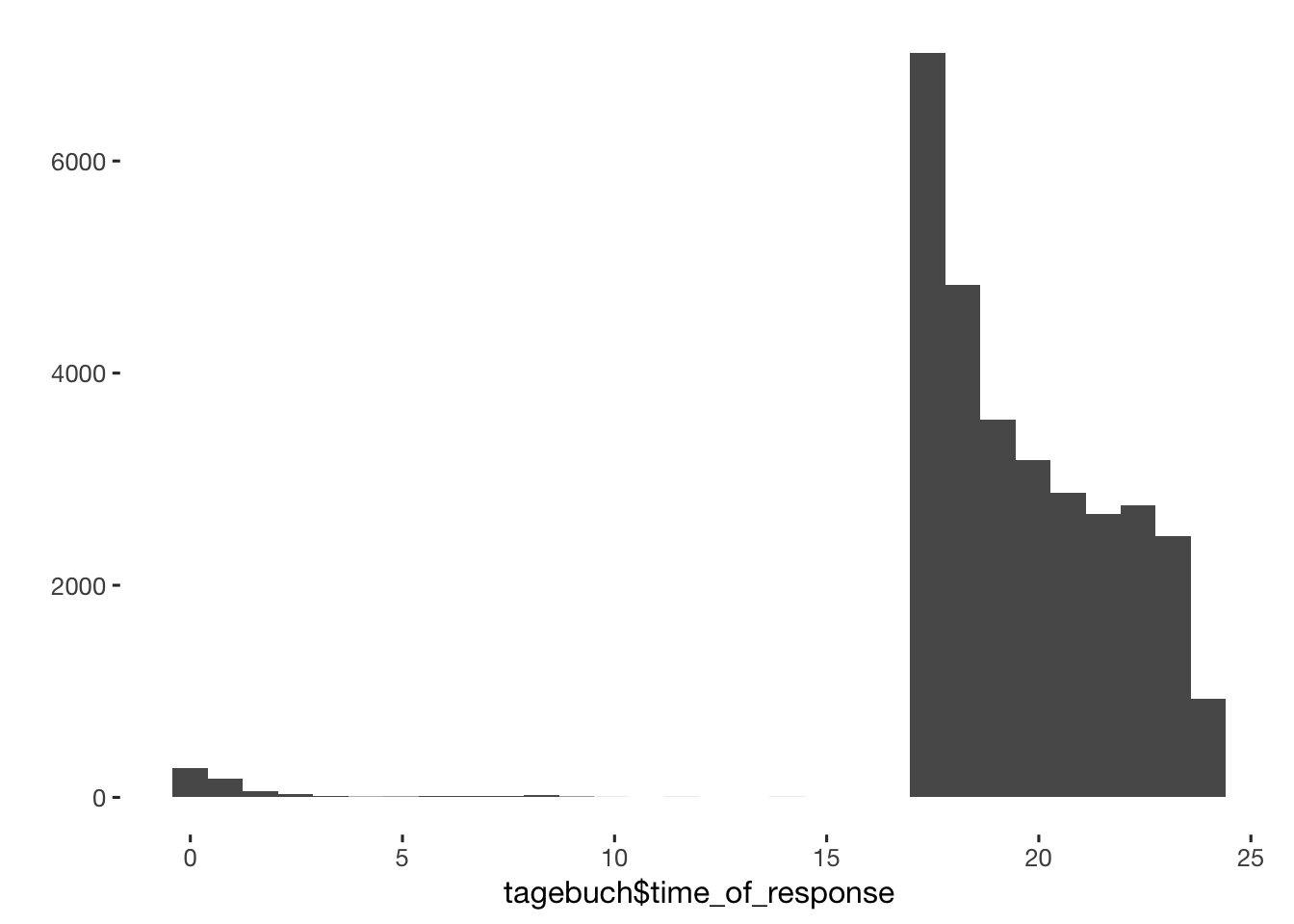table(tagebuch$time_of_response < 15 & tagebuch$time_of_response > 1) ## ## FALSE TRUE ## 30705 219 tagebuch$dodgy_data = if_else(tagebuch$time_of_response < 15 & tagebuch$time_of_response > 1, T, tagebuch$dodgy_data, F) tagebuch$time_of_response[which(tagebuch$time_of_response < 15 & tagebuch$time_of_response > 1)] = 1 # access time abberrations
tagebuch$time_of_response[which(tagebuch$time_of_response < 15)] =
tagebuch$time_of_response[which(tagebuch$time_of_response < 15)] + 24
qplot(tagebuch$time_of_response, binwidth = 5/60)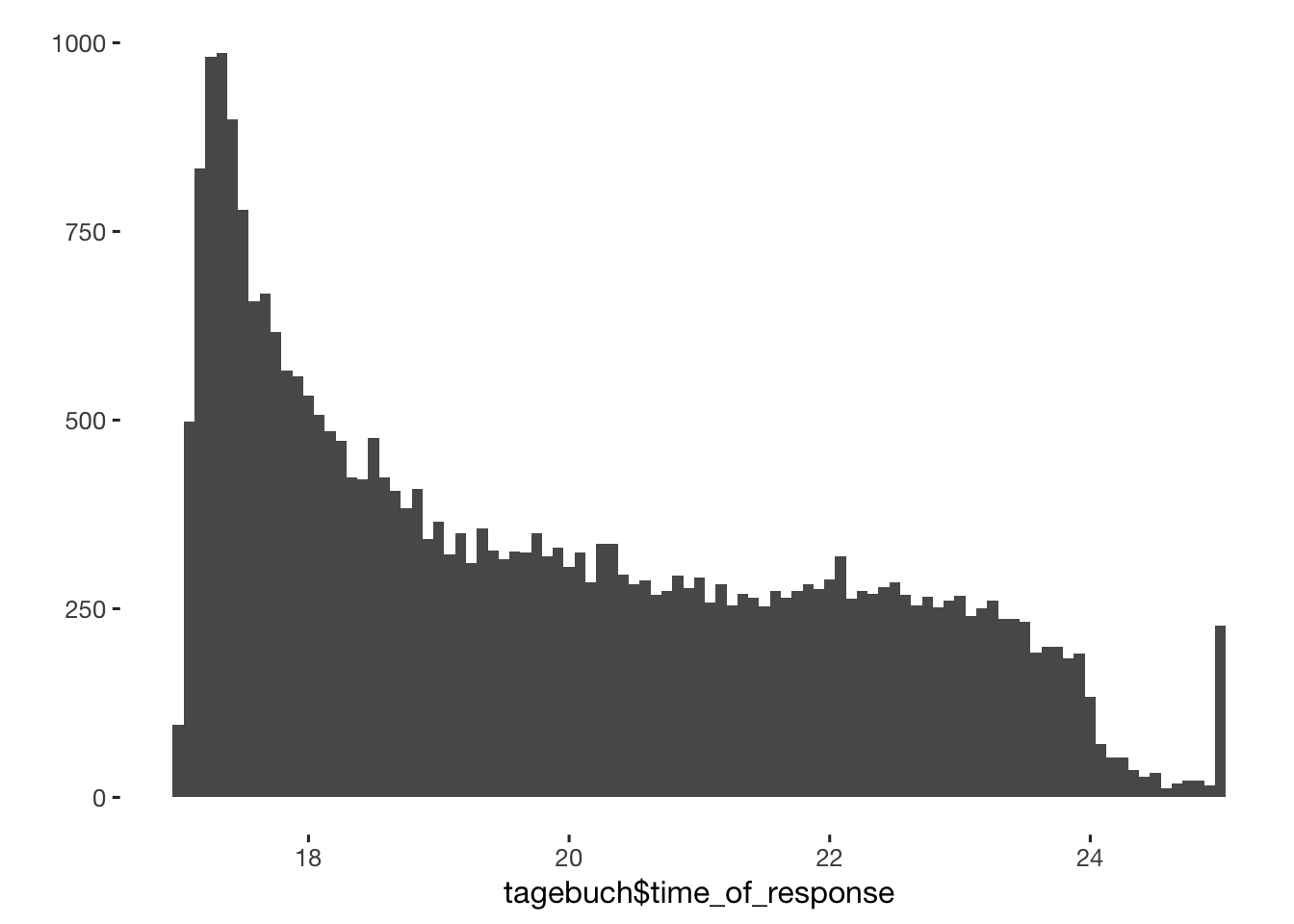tagebuch$time_for_response = as.numeric(tagebuch$ended - tagebuch$created, units = "mins")

table(tagebuch$time_for_response > 60*24) ## ## FALSE TRUE ## 30548 376 tagebuch$dodgy_data = if_else(tagebuch$time_for_response > 60*24, T, tagebuch$dodgy_data)
table(tagebuch$time_for_response < 1) ## ## FALSE TRUE ## 30894 30 tagebuch$dodgy_data = if_else(tagebuch$time_for_response < 1, T, tagebuch$dodgy_data)

table(tagebuch$time_for_response > 60*24) ## ## FALSE TRUE ## 30548 376 table(tagebuch$time_for_response > 60*7)
##
## FALSE  TRUE
## 29736  1188
tagebuch$time_for_response[tagebuch$time_for_response > 60 * 7] = NA

qplot(tagebuch$time_for_response) + scale_x_log10()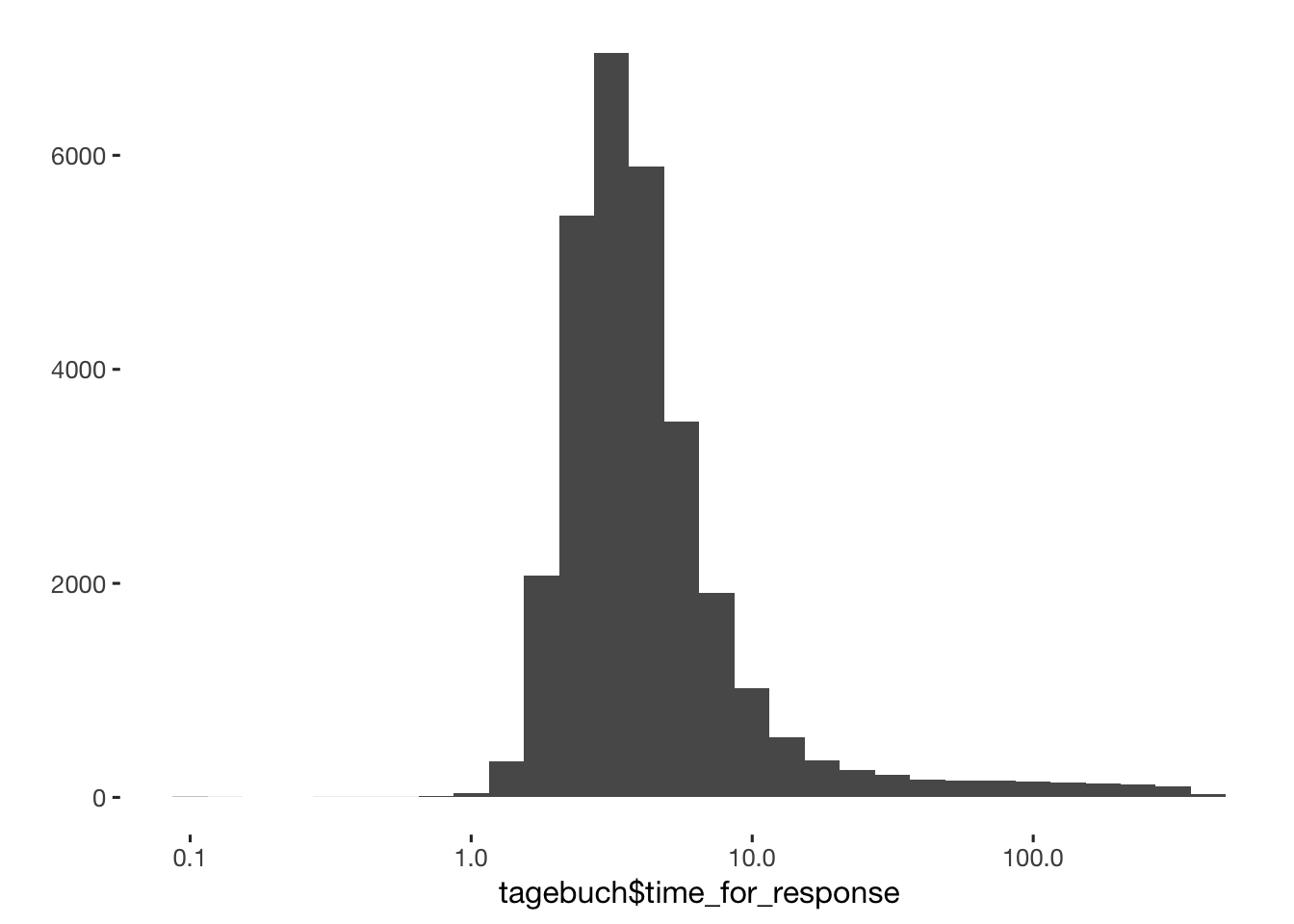qplot(tagebuch$time_for_response) + xlim(0,10)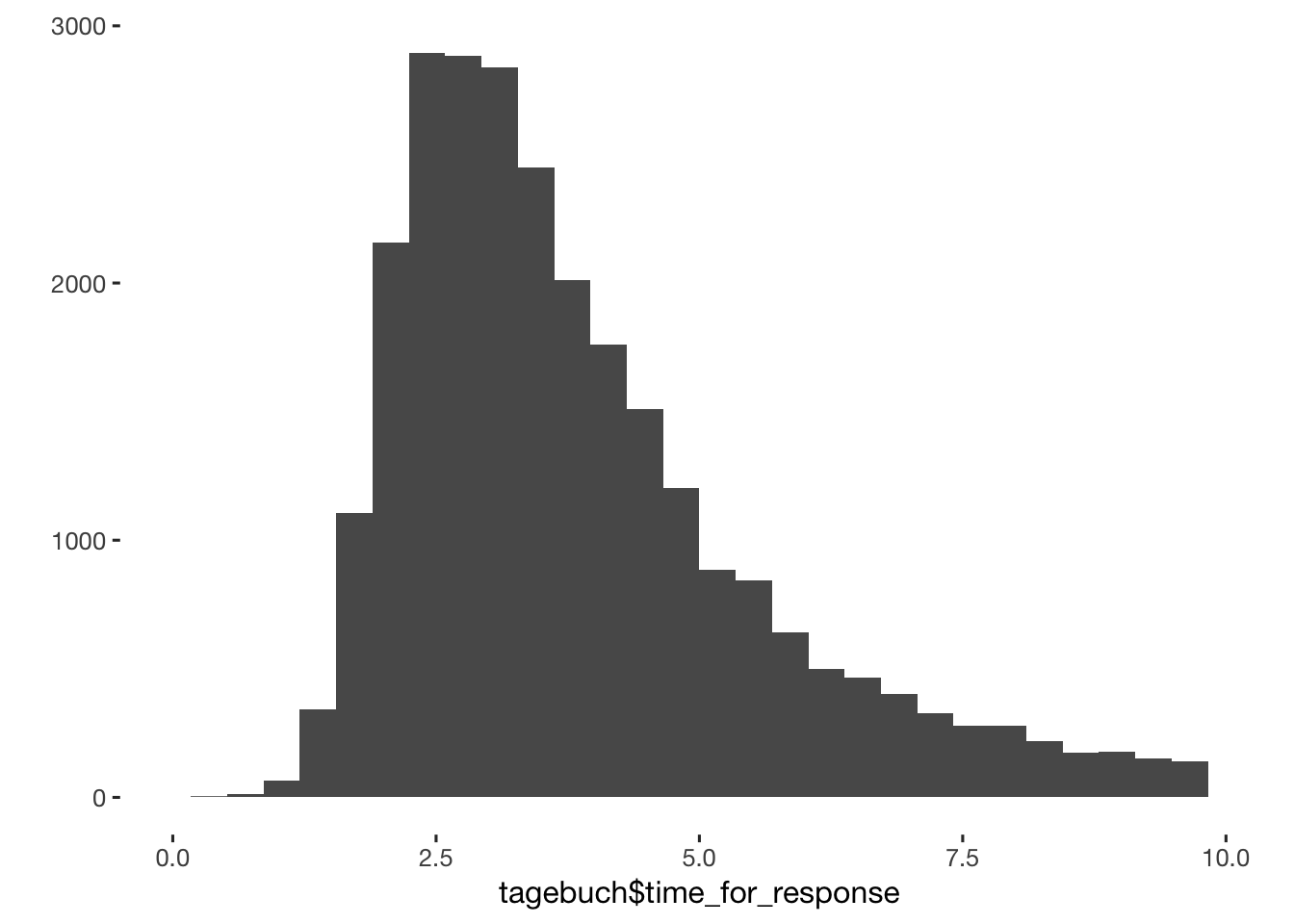table(tagebuch$time_for_response < 1) ## ## FALSE TRUE ## 29706 30 sd(tagebuch$time_for_response[tagebuch$time_for_response<20], na.rm = T) ##  2.695 mean(tagebuch$time_for_response[tagebuch$time_for_response<20], na.rm = T) ##  4.306 tagebuch$time_for_response[tagebuch$time_for_response>100] = NA tagebuch = tagebuch %>% group_by(session) %>% arrange(session,created, ended) %>% mutate(time_since_last_response = as.numeric(created - lag(ended) , units = "hours")) qplot(tagebuch$time_since_last_response) + scale_x_log10()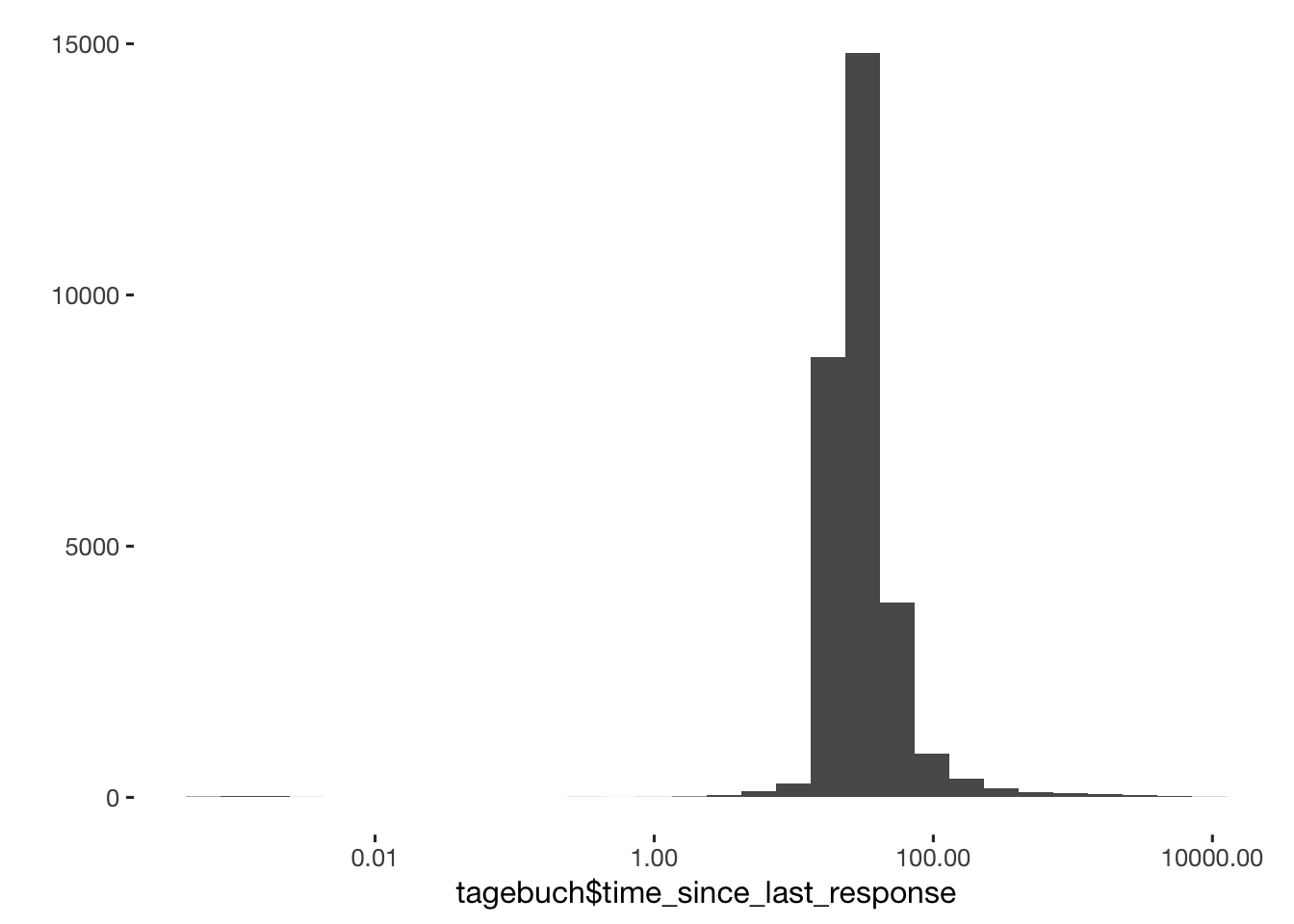table(round(tagebuch$time_since_last_response)) %>% head(24) ## ## -121 -18 -15 0 1 2 3 4 5 6 7 8 9 10 11 12 13 14 15 16 17 18 ## 1 1 1 73 8 8 28 23 36 40 44 65 68 52 34 39 39 25 34 28 117 419 ## 19 20 ## 830 1047 table(tagebuch$time_since_last_response < 17)
##
## FALSE  TRUE
## 29071   677
tagebuch = tagebuch %>%
mutate(
dodgy_data = if_else(time_since_last_response < 17, T, dodgy_data, dodgy_data),
# timing mishaps?
time_since_last_response = if_else(time_since_last_response < 17, NA_real_,
# skipped days
if_else(time_since_last_response > 31, NA_real_, time_since_last_response)))

qplot(tagebuch$time_since_last_response)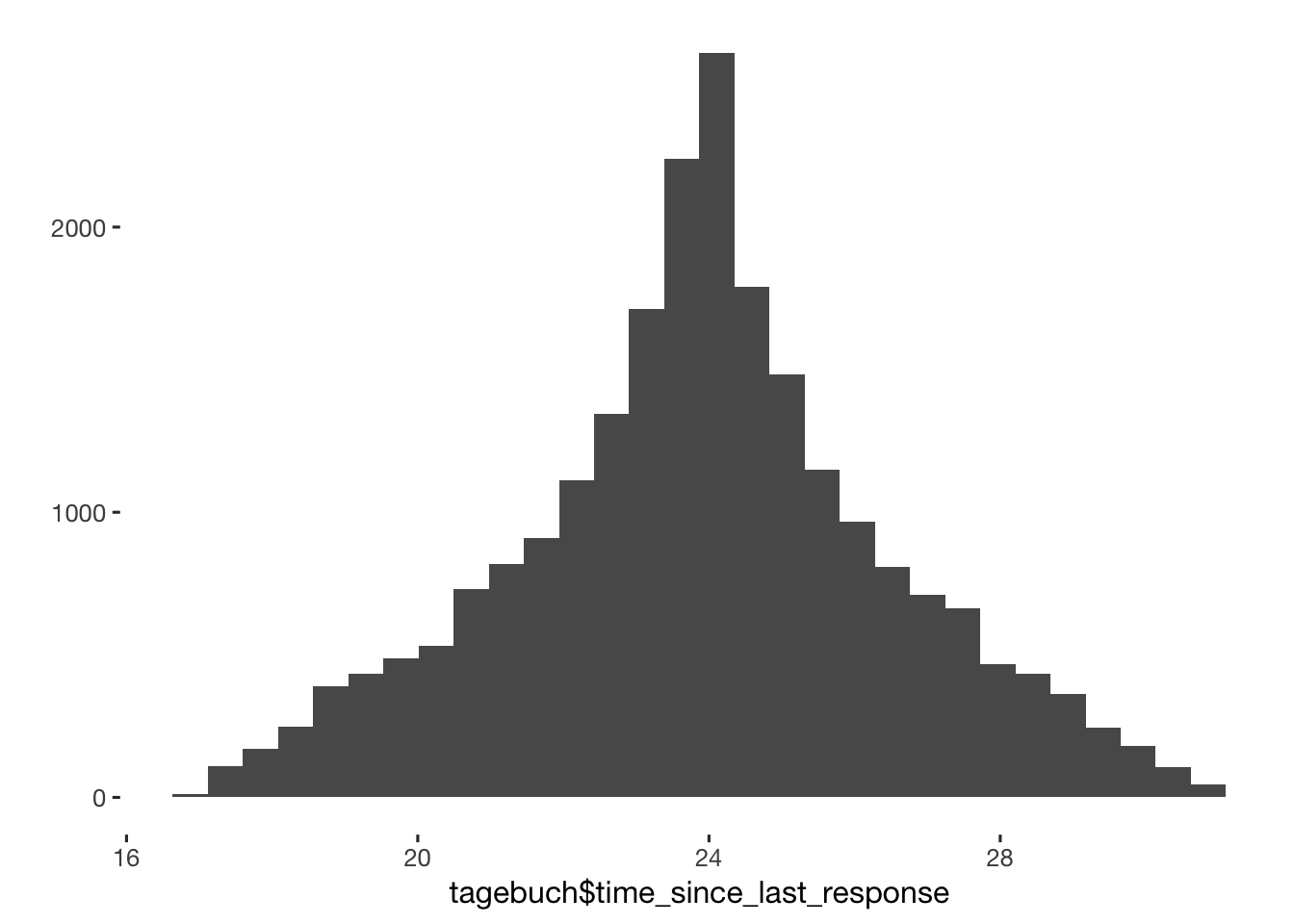tagebuch = tagebuch %>% ungroup() %>% tbl_df() ### Lazy days Check for days on which probands entered nonsensical data. likert_scales = tagebuch %>% select(session, created, choice_of_clothing_1, choice_of_clothing_2, choice_of_clothing_3, choice_of_clothing_4, choice_of_clothing_5, choice_of_clothing_6, choice_of_clothing_7, choice_of_clothing_8, self_esteem_1, desirability_1, desirability_partner, NARQ_admiration_1, NARQ_admiration_2, NARQ_admiration_3, NARQ_rivalry_1, NARQ_rivalry_2, NARQ_rivalry_3, jealousy_1, male_jealousy_1, male_jealousy_2, male_jealousy_3, male_mate_retention_1, male_mate_retention_2, mate_retention_3, mate_retention_4, mate_retention_5, mate_retention_6, attention_1, attention_2, male_attention_1, extra_pair_2, extra_pair_3, extra_pair_4, extra_pair_5, extra_pair_6, extra_pair_7, extra_pair_8, extra_pair_9, extra_pair_10, extra_pair_11, extra_pair_12, extra_pair_13) likert_scales = likert_scales %>% gather(variable, value, -session, -created) %>% group_by(session,created) # all 6-point scales crosstabs(~ variable + value, data = likert_scales) ## value ## variable 1 2 3 4 5 6 <NA> ## attention_1 2950 1413 3069 7681 7269 7492 1050 ## attention_2 7785 3194 4982 5502 4166 4245 1050 ## choice_of_clothing_1 6472 3381 5966 7768 4545 1764 1028 ## choice_of_clothing_2 628 1046 3185 9221 8696 7116 1032 ## choice_of_clothing_3 2782 2963 5880 8896 6298 3068 1037 ## choice_of_clothing_4 5639 4092 7002 8183 3918 1050 1040 ## choice_of_clothing_5 14708 5023 5030 3347 1400 377 1039 ## choice_of_clothing_6 3567 2175 4804 10377 6510 2450 1041 ## choice_of_clothing_7 10425 4983 7017 4902 2004 550 1043 ## choice_of_clothing_8 8689 4507 7087 5938 2720 941 1042 ## desirability_1 3063 2944 6093 9126 5787 2868 1043 ## desirability_partner 3019 2413 5435 9046 6032 3934 1045 ## extra_pair_10 24168 1382 1145 1629 781 768 1051 ## extra_pair_11 26789 1080 728 773 299 204 1051 ## extra_pair_12 16870 2130 2522 4655 2281 1415 1051 ## extra_pair_13 24682 1332 930 1396 663 870 1051 ## extra_pair_2 12039 3570 4078 5888 2883 1416 1050 ## extra_pair_3 19277 2631 2303 2754 1518 1390 1051 ## extra_pair_4 26262 1237 763 825 355 431 1051 ## extra_pair_5 21919 991 877 1804 1429 2853 1051 ## extra_pair_6 18929 1310 1403 2853 2036 3342 1051 ## extra_pair_7 22819 2032 1579 1865 875 703 1051 ## extra_pair_8 26492 1258 880 766 277 200 1051 ## extra_pair_9 25338 1245 995 1262 522 511 1051 ## jealousy_1 16788 1826 2111 3706 2333 3113 1047 ## male_attention_1 7147 2601 3986 5240 4890 6010 1050 ## male_jealousy_1 24376 2162 1432 1209 429 268 1048 ## male_jealousy_2 24805 1817 1312 1176 433 334 1047 ## male_jealousy_3 27378 1128 600 403 191 176 1048 ## male_mate_retention_1 17532 1787 2043 3564 2062 2888 1048 ## male_mate_retention_2 7590 1225 2136 3458 3508 11959 1048 ## mate_retention_3 10603 2841 4843 5519 3674 2396 1048 ## mate_retention_4 7577 1239 2205 3522 3550 11783 1048 ## mate_retention_5 22085 2475 1817 2071 824 604 1048 ## mate_retention_6 9646 3944 5689 6026 2967 1603 1049 ## NARQ_admiration_1 10545 4120 6077 6020 2289 826 1047 ## NARQ_admiration_2 10803 3991 5827 6000 2397 859 1047 ## NARQ_admiration_3 11050 3939 5601 5852 2510 925 1047 ## NARQ_rivalry_1 24605 2457 1357 960 276 221 1048 ## NARQ_rivalry_2 25617 1753 960 894 321 332 1047 ## NARQ_rivalry_3 26119 1829 1012 611 202 103 1048 ## self_esteem_1 1115 1290 3612 9965 9971 3928 1043 no_variation = likert_scales %>% summarise(no_variation = sd(value,na.rm = T) == 0) table(no_variation$no_variation)
##
## FALSE  TRUE
## 29868    23
tagebuch = tagebuch %>% left_join(no_variation, by = c("session", "created")) %>%
mutate(dodgy_data = if_else(no_variation, TRUE, dodgy_data, dodgy_data))

table(tagebuch$dodgy_data, exclude = NULL) ## ## FALSE TRUE ## 29660 1264 tagebuch = tagebuch %>% tbl_dt() ### Correcting errors in our surveys that we noticed while the study ran On April 25, 2015, we changed an item prior to the evening diary. The item relationship_satisfaction_1 had a mix-up in the response options 4 and 5, so that “mostly satisfied” came before “rather satisfied”. Hence, we cannot interpret 4 and 5 very well prior to 4/25 because we can’t know whether subjects respected the order or the phrasing. Hence, we decided to assign both 4s and 5s a 4.5 prior to April 25. We did the same thing for the item sexual_intercourse_4 on May 29. library(car) table(tagebuch$relationship_satisfaction_1)
##
##     1     2     3     4     5     6
##   707   880  2189  5569  9915 10642
tagebuch[, relationship_satisfaction_1 := as.numeric(relationship_satisfaction_1)]
tagebuch[created < as.POSIXct('2014-04-25') , relationship_satisfaction_1 := Recode(relationship_satisfaction_1, "4=4.5;5=4.5")]
table(tagebuch$relationship_satisfaction_1) ## ## 1 2 3 4 4.5 5 6 ## 707 880 2189 5036 1440 9008 10642 table(tagebuch$sexual_intercourse_4)
##
##    1    2    3    4    5    6
##   46   78  250 1060 2188 2889
tagebuch[, sexual_intercourse_4 := as.numeric(sexual_intercourse_4)]
tagebuch[created < as.POSIXct('2014-04-29') , sexual_intercourse_4 := Recode(sexual_intercourse_4, "4=4.5;5=4.5")]
table(tagebuch$sexual_intercourse_4) ## ## 1 2 3 4 4.5 5 6 ## 46 78 250 916 445 1887 2889 xsection$SOI_be = rowMeans(xsection[, list(SOI_R_1, SOI_R_2, SOI_R_3)])
xsection$SOI_im = rowMeans(xsection[, list(SOI_R_7, SOI_R_8, SOI_R_9)]) xsection$SOI_uv = rowMeans(xsection[, list(SOI_R_4, SOI_R_5, SOI_R_6)])

# this woman told us she reported masturbation as "intimacy with someone other than my partner"
tagebuch[session %begins_with% "ce8e40de7c", ]$extra_pair_1 = NA tagebuch[session %begins_with% "ce8e40de7c", ]$extra_pair_1b = NA
xsection$duration_relationship_total <- xsection$duration_relationship_months + xsection$duration_relationship_years*12 # create new variable duration_relationship_total ### Import pill dosage xsection[, hormonal_contraception := ifelse( contraception %contains% "contraceptive_pill" | contraception %contains% "other_hormonal", 1, 0) ] qplot(factor(xsection$hormonal_contraception))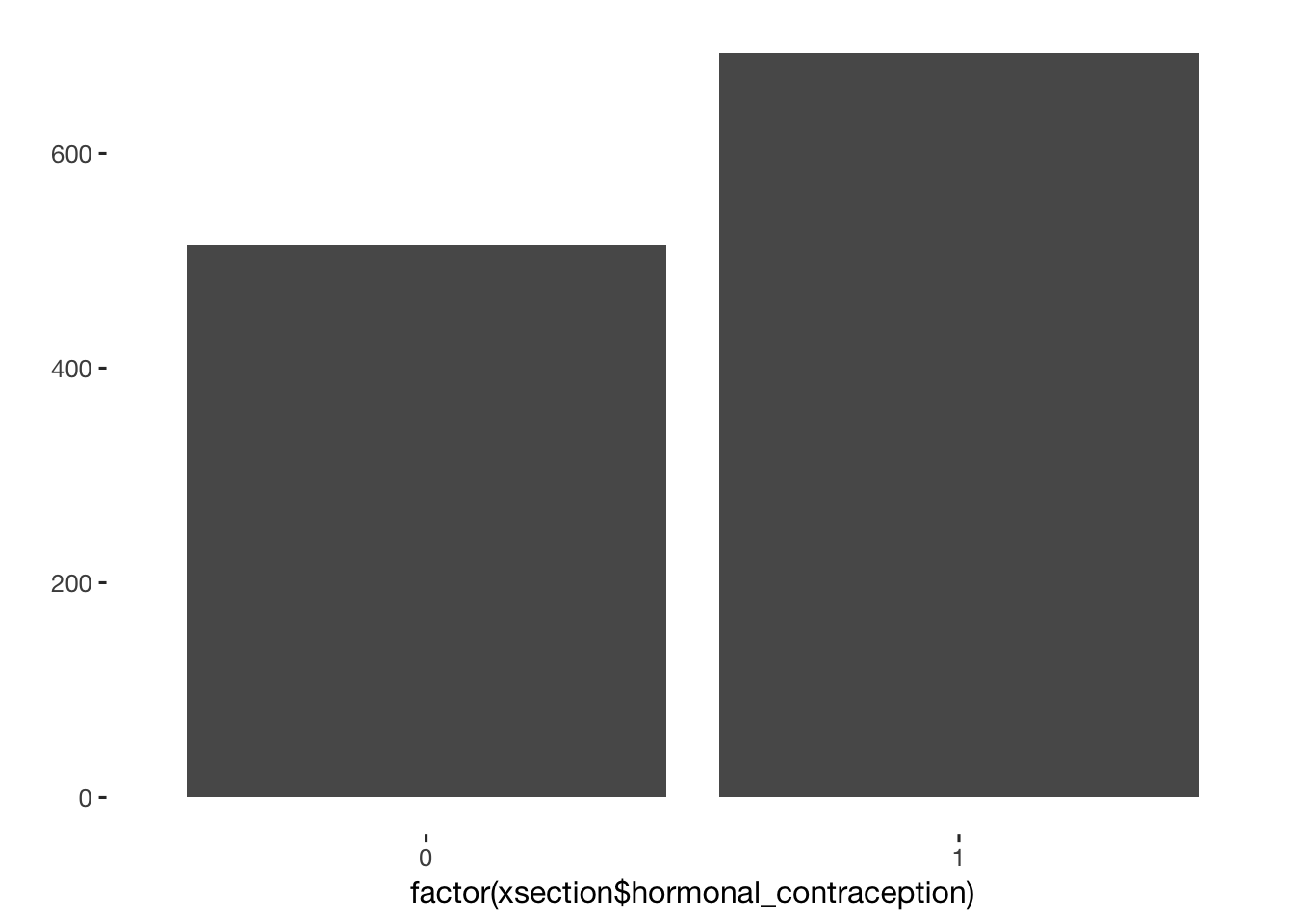xsection[, pill_contraception := ifelse( contraception %contains% "contraceptive_pill", 1, 0) ]
qplot(factor(xsection$pill_contraception))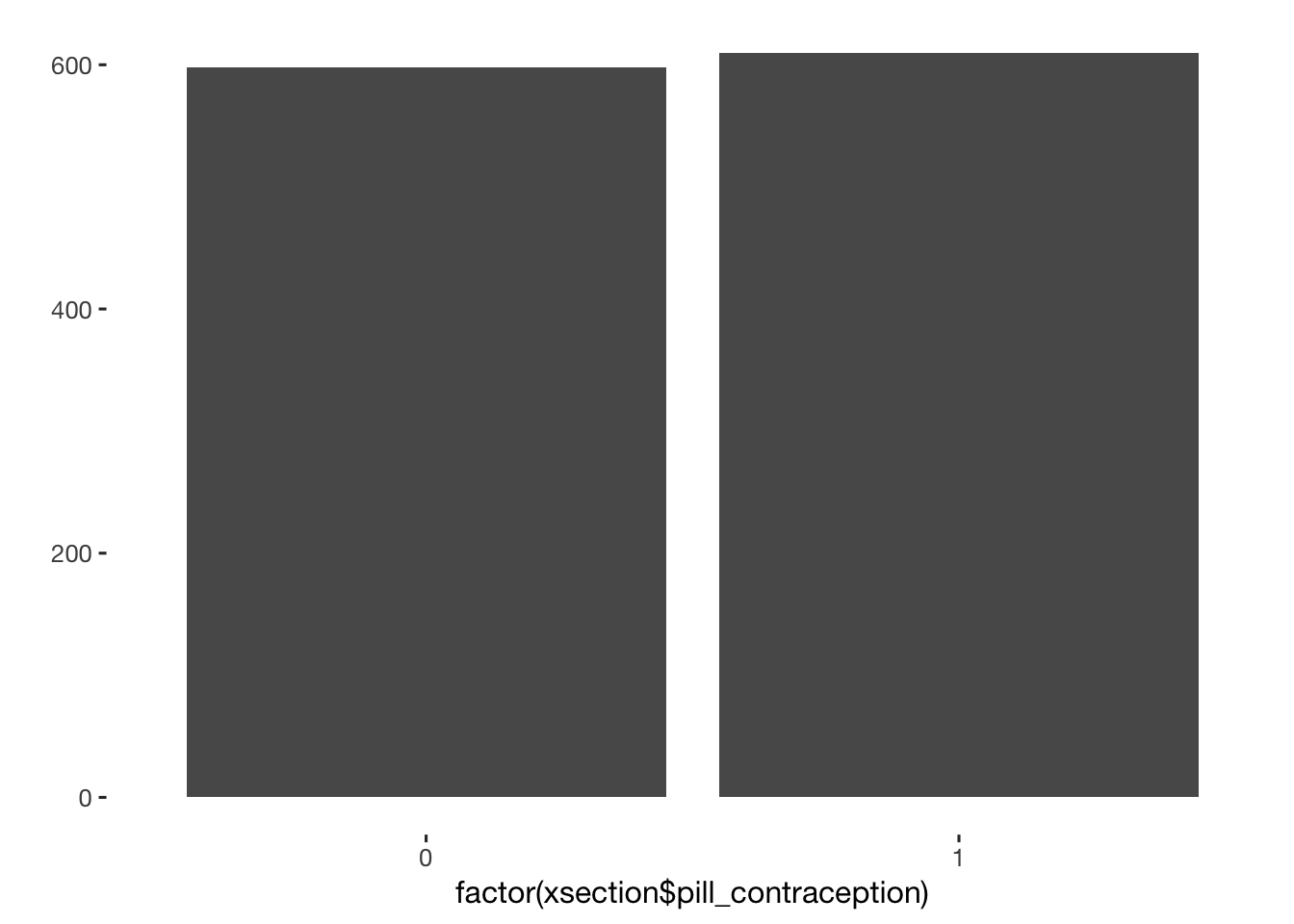# library(xlsx) # pillen2 = read.xlsx( "item_tables/Vorab_Fragebogen1-v3.xlsx", sheetName = "Choices") pillen = readxl::read_excel( "item_tables/Vorab_Fragebogen1-v3.xlsx",sheet = "Choices") %>% data.frame() pillen = as.data.table(pillen[ 1:97, ]) pillen[, gestagen_ug_merged := as.numeric(as.character( str_replace_all( str_replace_all( str_replace_all( Gestagen.Milligramm.pro.Pille, '500', ',500'), '000', '') ,pattern = ',','.') )) * 1000] pillen[, gestagen_cycle := as.numeric(as.character( str_replace_all( Gestagen.Milligramm.pro.Zyklus , pattern = ',','.')))] pillen[name == "yasmin", ] crosstabs(pillen$gestagen_ug_merged)
## pillen$gestagen_ug_merged ## 30 60 75 100 125 150 250 2000 2500 3000 <NA> ## 2 1 7 10 3 14 1 31 1 8 19 pillen[, estrogen_ug_cycle := as.numeric(as.character( Östrogenmikrogramm.pro.Zyklus )) ] pillen[, gestagen_type := as.character(Art.des.Gestagens) ] crosstabs(pillen$gestagen_type)
## pillen$gestagen_type ## CMA CPA DNG DSG DSP GSD LNG NES NGT NOMAC <NA> ## 16 10 8 18 8 3 26 4 2 1 1 pillen[, pills := as.character(name)] pillen[is.na(estrogen_ug_cycle), list(pills, Östrogenmikrogramm.pro.Zyklus) ] pillen[is.na(gestagen_ug_merged), list(pills, Gestagen.Milligramm.pro.Pille) ] pillen = pillen[ pills != 'other', ] pillen[is.na(Östrogenmikrogramm.pro.Zyklus), estrogen_ug_cycle := 0 ] pillen[is.na(Östrogenmikrogramm.pro.Pille), estrogen_ug_merged := 0 ] qplot(pillen$estrogen_ug_cycle)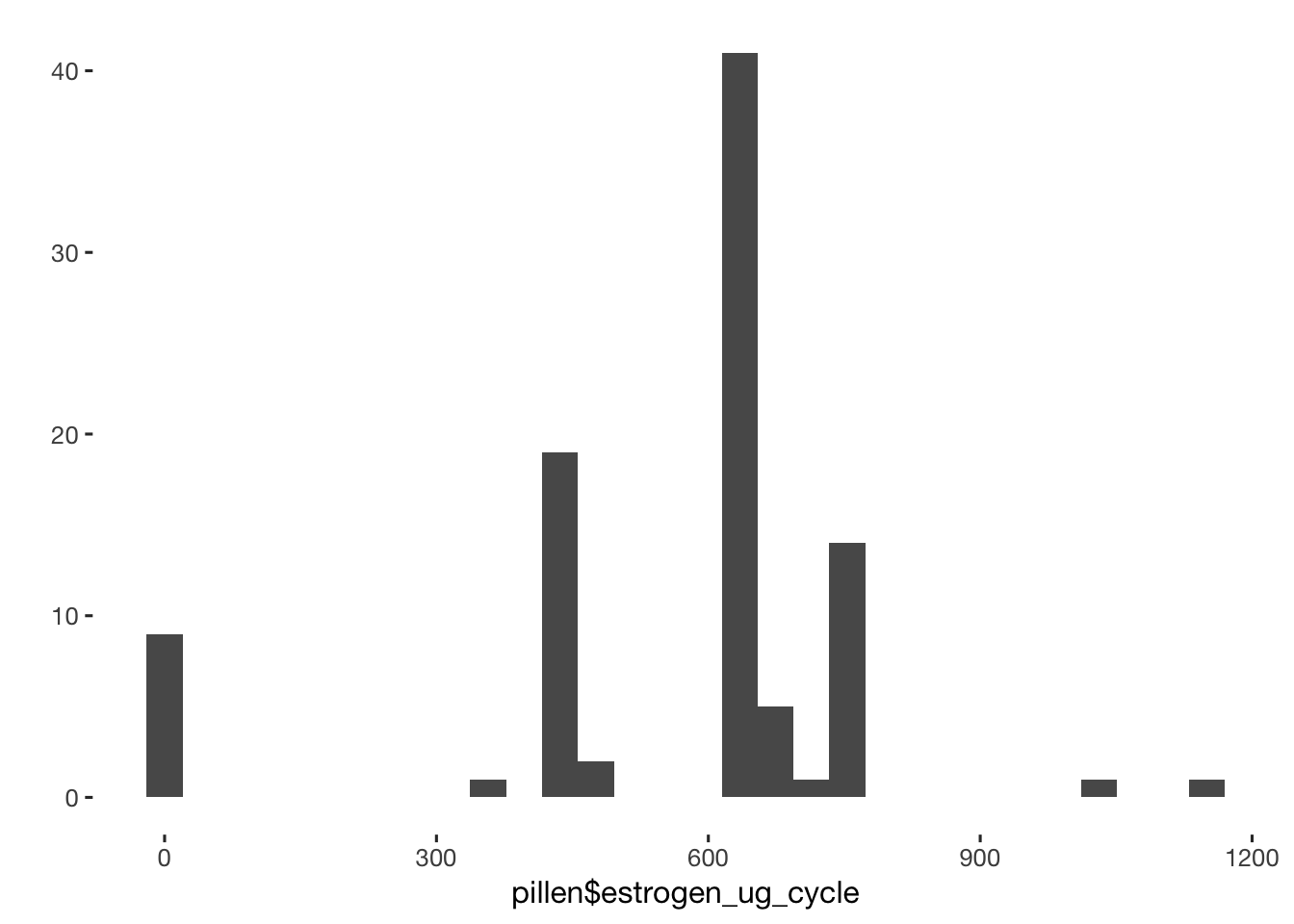table(xsection$pills == "qlaira") ## ## FALSE TRUE ## 606 4 table(xsection$pills == "zoely")
##
## FALSE  TRUE
##   602     8
xsection = merge(xsection, pillen[,list(pills, estrogen_ug_merged,  estrogen_ug_cycle
, gestagen_type,gestagen_ug_merged, gestagen_cycle
)], by = "pills", all.x = T)
table(xsection$pills == "other") ## ## FALSE TRUE ## 556 54 crosstabs(pillen[, estrogen_ug_cycle/estrogen_ug_merged]) ## pillen[, estrogen_ug_cycle/estrogen_ug_merged] ## NaN <NA> ## 9 87 ## Estrogen Levels for all people (also for those who didnt take the pill) crosstabs(xsection$estrogen_ug_cycle)
## xsection$estrogen_ug_cycle ## 0 360 420 480 630 665 680 730 735 1150 <NA> ## 26 1 141 11 328 3 4 2 26 2 664 xsection[, estrogen_ug_all := ifelse(hormonal_contraception, estrogen_ug_cycle, 0)] crosstabs(xsection$estrogen_ug_all)
## xsection$estrogen_ug_all ## 0 360 420 480 630 665 680 730 735 1150 <NA> ## 540 1 141 11 328 3 4 2 26 2 150 # Forming Categories xsection[, estrogen_categories := cut(estrogen_ug_all, breaks = c(0, 300, 600, 1200), include.lowest = T)] crosstabs(xsection$estrogen_categories)
## xsection$estrogen_categories ## [0,300] (300,600] (600,1.2e+03] ## 540 153 365 #### Code other pill names cat(xsection[ pills=='other' & is.na(estrogen_ug_cycle), str_c( str_sub(session, 1, 10),"\n", other_pill_name,"\n\n") ]) ## 033d3e1d21 ## yris mite ## ## 0643a31c42 ## swingo ## ## 0d9a341ceb ## desofemine 20 Nova ## ## 1528e9536b ## dienovel ## ## 1c93be41a1 ## Carlin ## ## 1f9fc2cc79 ## Kleodina ## ## 21054c807c ## Maitalon ## ## 2433cc44b9 ## ludéal glé ## ## 24af1309b4 ## Estinette ## ## 29220ba555 ## Swingo ## ## 319e2ce5cb ## Midane ## ## 354137dd7f ## Sibilla ## ## 37226a6cf2 ## Asumate 30 ## ## 3d0adfe3a2 ## Aristelle ## ## 4167741e29 ## yara hexal ## ## 4202863d09 ## Cedia ## ## 47b6e59dfa ## Layaisa ## ## 4aafa757ef ## Eliza ## ## 511189d8a0 ## Selina ## ## 59858e60ea ## Solera ## ## 5b4fad6f3f ## visanne ## ## 62f9888d39 ## Swingo 30 ## ## 640a7b047b ## aristelle ## ## 646c651ea4 ## Leanova AL ## ## 6da65c4e45 ## Dienovel ## ## 82aadd4d54 ## Dienovel ## ## 83c84a5860 ## Selina ## ## 86fb131347 ## Aristelle ## ## 8bd7c4624b ## Dienovel ## ## 8c118bfaaa ## Swingo ## ## 8f892266fb ## Yvette ## ## 9336f57640 ## Midane ## ## 9700064ee1 ## Dienovel ## ## 9b49e26d03 ## Minette ## ## 9c89af2b7f ## Microgynon ## ## 9f61438d5f ## Violette ## ## aa38292783 ## minesse ## ## aebdd368b4 ## Swingo 30 ## ## b726d9cd36 ## Swingo ## ## c09518da85 ## Lysandra beta ## ## c33d554719 ## Dienovel ## ## c6dd42db4b ## desofemono ## ## c8ca0944d9 ## Velafee ## ## cf37f837bc ## Dienogenance ## ## d30783c574 ## Mirelle ## ## d5b5462be5 ## Solera ## ## e0eeb02960 ## LISA - Studienpille ## ## e2f049ef95 ## mercilon ## ## e68978de78 ## Desogestrel aristo ## ## e7e992c12b ## Desogestrel atisto ## ## edb19a9192 ## Solgest ## ## f42ae3caec ## sibilla ## ## f64039d090 ## Swingo 30 ## ## f9454c5d18 ## Dienovel xsection[ short == "0643a31c42", estrogen_ug := 20 ] xsection[ short == "0643a31c42", gestagens_ug := 100] xsection[ short == "0643a31c42", gestagens := "LNG"] xsection[ short == "1528e9536b", estrogen_ug := 30 ] xsection[ short == "1528e9536b", gestagens_ug := 2000] xsection[ short == "1528e9536b", gestagens := "DNG"] xsection[ short == "21054c807c", estrogen_ug := 20 ] xsection[ short == "21054c807c", gestagens_ug := 3000] xsection[ short == "21054c807c", gestagens := "DSP"] #one person swapped their values xsection[ short == "24af1309b4", estrogen_ug := 20 ] xsection[ short == "24af1309b4", gestagens_ug := 75] xsection[ short == "24af1309b4", gestagens := "GSD"] xsection[ short == "4167741e29", estrogen_ug := 20 ] xsection[ short == "4167741e29", gestagens_ug := 3000] xsection[ short == "4167741e29", gestagens := "DSP"] # visanne is used as a contraceptive only in combination with something containing estrogen, but we don't know what was the case here xsection[ short == "5b4fad6f3f", estrogen_ug := NA] xsection[ short == "5b4fad6f3f", gestagens_ug := NA] xsection[ short == "5b4fad6f3f", gestagens := NA_character_] xsection[ short == "71f06ef90f", estrogen_ug := 30 ] xsection[ short == "71f06ef90f", gestagens_ug := 300] xsection[ short == "71f06ef90f", gestagens := "DNG"] xsection[ short == "82aadd4d54", estrogen_ug := 30 ] xsection[ short == "82aadd4d54", gestagens_ug := 2000] xsection[ short == "82aadd4d54", gestagens := "DNG"] xsection[ short == "83c84a5860", estrogen_ug := 30 ] xsection[ short == "83c84a5860", gestagens_ug := 150] xsection[ short == "83c84a5860", gestagens := "LNG"] xsection[ short == "8bd7c4624b", estrogen_ug := 30 ] xsection[ short == "8bd7c4624b", gestagens_ug := 2000] xsection[ short == "8bd7c4624b", gestagens := "DNG"] xsection[ short == "9b49e26d03", estrogen_ug := 30 ] xsection[ short == "9b49e26d03", gestagens_ug := 2000] xsection[ short == "9b49e26d03", gestagens := "CMA"] xsection[ short == "9f61438d5f", estrogen_ug := 30 ] xsection[ short == "9f61438d5f", gestagens_ug := 2000] xsection[ short == "9f61438d5f", gestagens := "DNG"] xsection[ short == "b726d9cd36", estrogen_ug := 20 ] xsection[ short == "b726d9cd36", gestagens_ug := 100] xsection[ short == "b726d9cd36", gestagens := "LNG"] xsection[ short == "c09518da85", estrogen_ug := 35 ] xsection[ short == "c09518da85", gestagen_ug := 250] xsection[ short == "c09518da85", gestagens := "NGT"] xsection[ short == "c6dd42db4b", estrogen_ug := 0 ] xsection[ short == "c6dd42db4b", gestagens_ug := 75] xsection[ short == "c6dd42db4b", gestagens := "DSG"] xsection[ short == "e2f049ef95", estrogen_ug := 20 ] xsection[ short == "e2f049ef95", gestagens_ug := 60] xsection[ short == "e2f049ef95", gestagens := "DSG"] xsection[ short == "59858e60ea", estrogen_ug := 20 ] xsection[ short == "59858e60ea", gestagens_ug := 60] xsection[ short == "59858e60ea", gestagens := "DSG"] xsection[ pills == "other", list(other_pill_name, estrogen_ug,estrogen_ug_cycle, gestagens, gestagens_ug)] ## could also use info on gestagens etc xsection[ pills == "other", estrogen_ug_merged := estrogen_ug] # per pill xsection[ pills == "other", estrogen_ug_cycle := 21 * estrogen_ug] # times 21 xsection[ pills == "other", gestagen_type := gestagens] xsection[ pills == "other", gestagen_ug_merged := gestagens_ug] crosstabs(~is.na(xsection$pills) + is.na(xsection$estrogen_ug_cycle)) # except qlaira and zoely ## is.na(xsection$estrogen_ug_cycle)
## is.na(xsection$pills) FALSE TRUE ## FALSE 597 13 ## TRUE 0 598 qplot(xsection$estrogen_ug_cycle)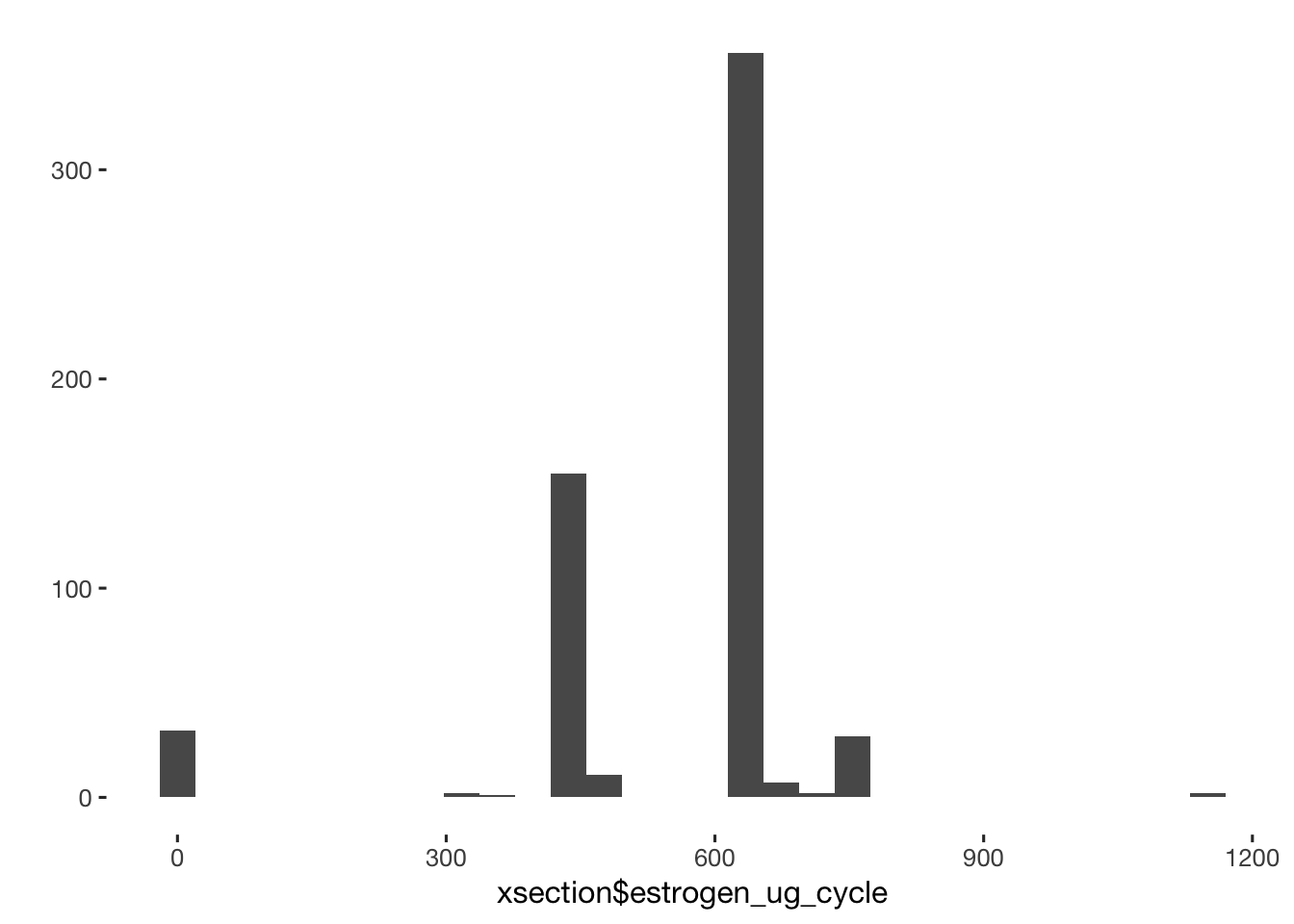# qplot(xsection$gestagen_ug_merged) # xsection[ gestagen_ug_merged < 100, ] # xsection[gestagen_ug_merged <= estrogen_ug_merged, list(pills, other_pill_name, gestagen_ug_merged, estrogen_ug_merged) ] qplot(data=xsection, estrogen_ug_cycle, estrogen_ug_merged, geom = 'jitter')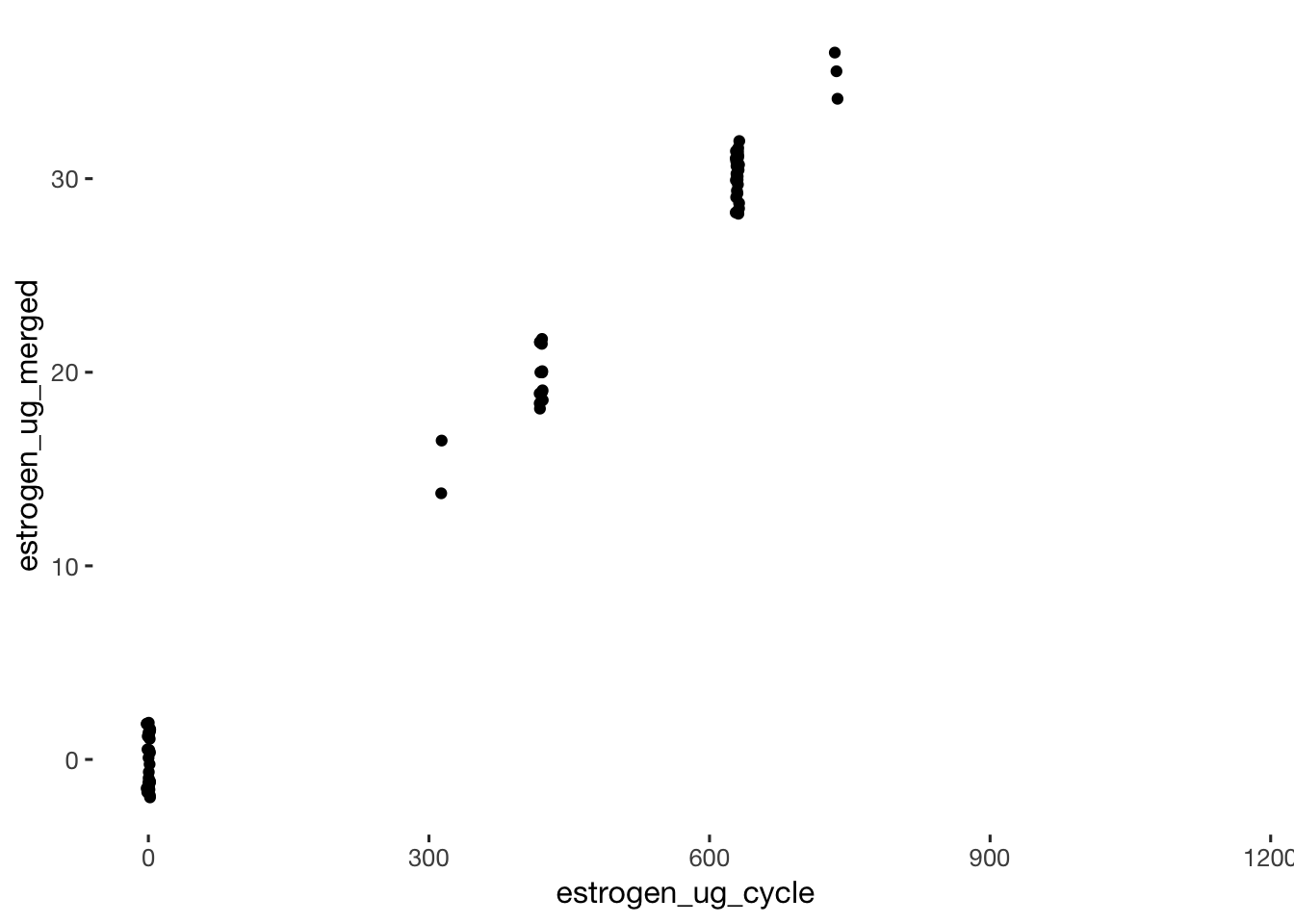### Use detailed info on non-pill hormonal contraception from post survey # library(xlsx) other_hormonal = readxl::read_excel( "item_tables/Nachbefragung_other_hormonal.xlsx", sheet = "Choices") %>% data.frame() other_hormonal = as.data.table(other_hormonal) other_hormonal[, hormonal_2 := as.character( name)] xsection = merge(xsection, other_hormonal[,list(hormonal_2, estrogen_ug_other)], by = "hormonal_2", all.x = T) nrow(xsection[!is.na(xsection$estrogen_ug_other),])
##  39
table(xsection$estrogen_ug_other) ## ## 0 315 420 ## 12 26 1 xsection[!is.na(estrogen_ug_other), estrogen_ug_cycle := estrogen_ug_other] ### Pill by weight qplot(data = xsection, estrogen_ug_merged, estrogen_ug_cycle)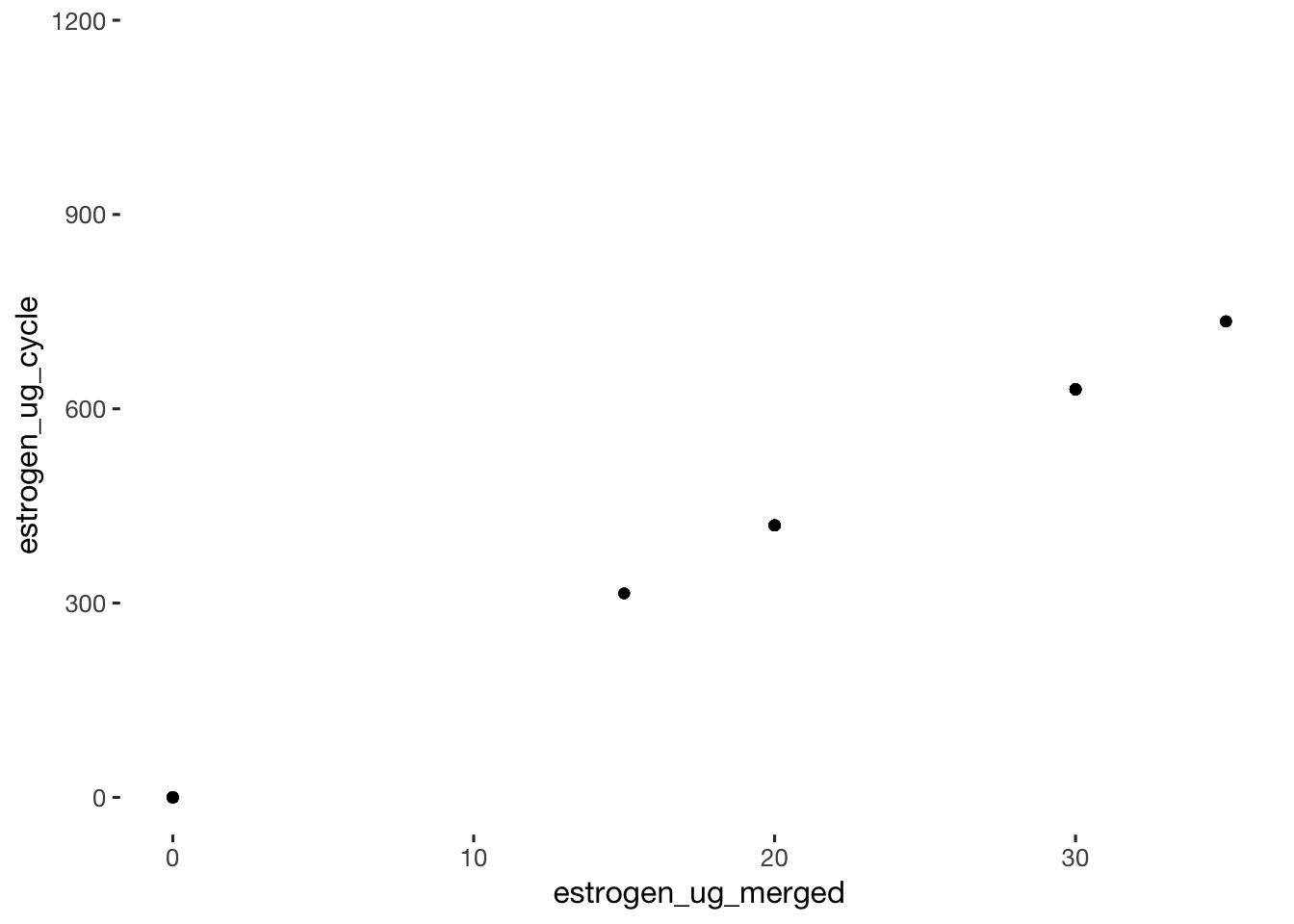qplot(data = xsection, estrogen_ug_cycle, weight) + geom_smooth() # no association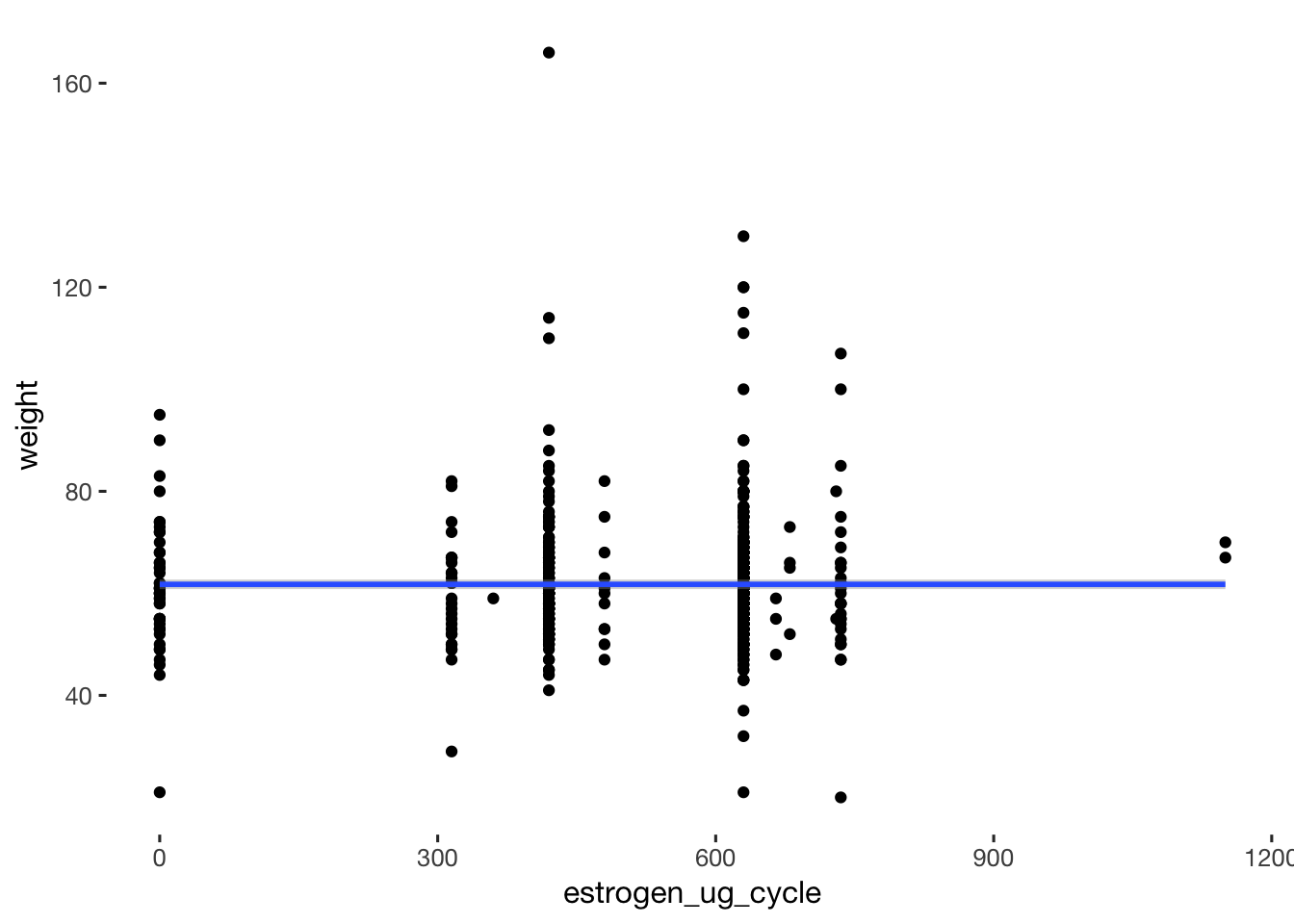qplot(data = xsection, estrogen_ug_cycle / weight)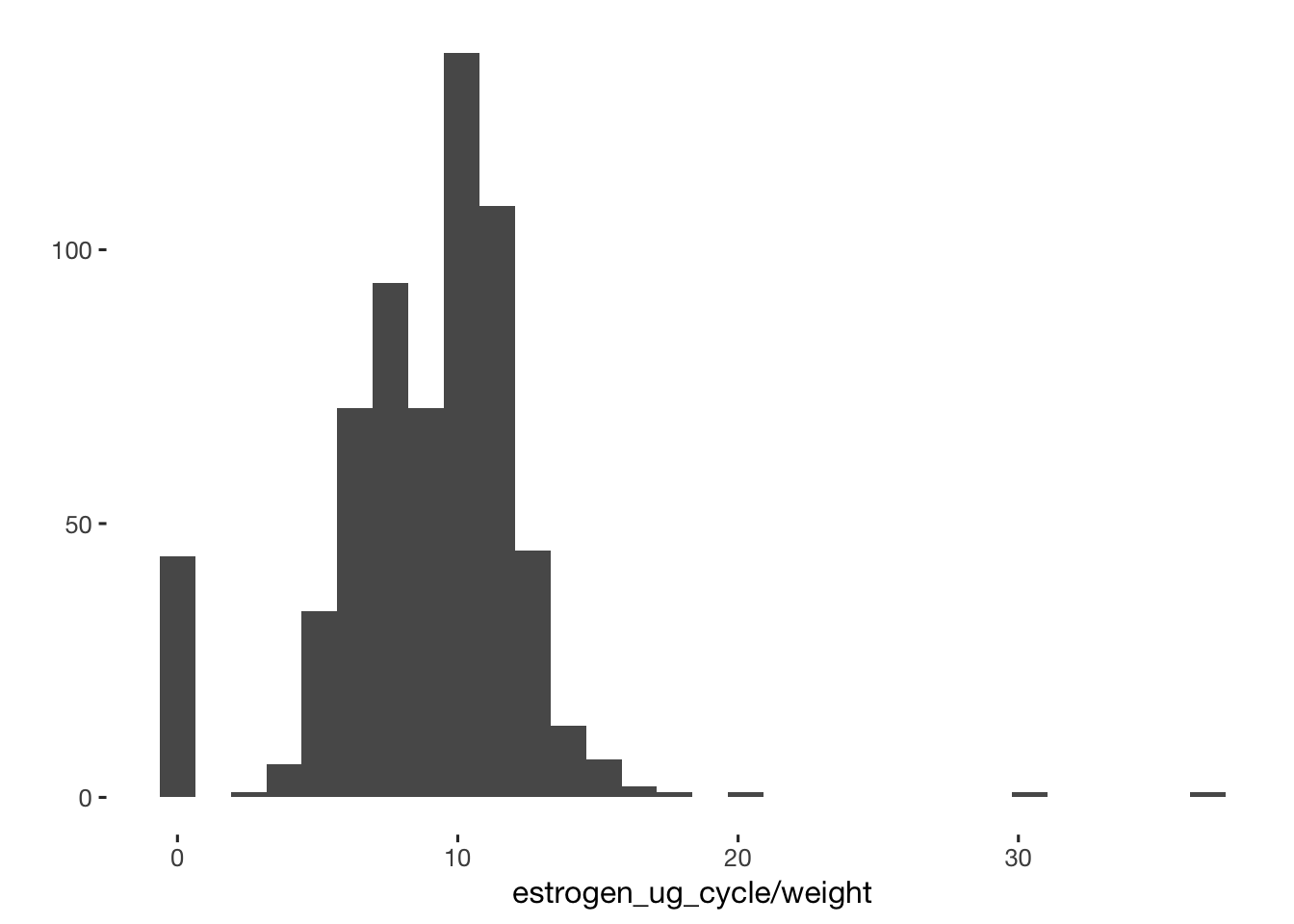table(xsection$weight)
##
##  20  21  22  28  29  30  32  35  37  41  43  44  45  46  47  48  49  50  51  52  53  54  55  56  57  58  59
##   4   3   1   1   2   1   2   1   2   2   4   3   6   6  13  11  19  38  21  38  42  37  73  36  42  73  45
##  60  61  62  63  64  65  66  67  68  69  70  71  72  73  74  75  76  77  78  79  80  81  82  83  84  85  86
##  82  33  45  45  36  61  39  25  34  21  45  12  15  10  10  29   7   7   9   6  25   3  11   5   5   6   2
##  87  88  89  90  91  92  93  95  97  99 100 101 105 107 110 111 112 113 114 115 120 130 134 143 166
##   1   4   2   5   1   1   1   5   2   3   5   1   3   2   1   2   1   1   1   2   6   1   1   1   1
xsection[, estrogen_ug_per_kg := estrogen_ug_cycle / weight]
xsection[ estrogen_ug_per_kg > 20, list(estrogen_ug_cycle, weight, height)]

crosstabs(xsection$estrogen_ug_cycle) ## xsection$estrogen_ug_cycle
##    0  315  360  420  480  630  665  680  730  735 1150 <NA>
##   44   28    1  156   11  356    3    4    2   29    2  572

## Scales

### diary scales

# create  NARQ_K_total scale

tagebuch$NARQ_K_total = rowMeans(tagebuch[, list(NARQ_admiration_1, NARQ_admiration_2, NARQ_admiration_3, NARQ_rivalry_1, NARQ_rivalry_2, NARQ_rivalry_3 ) ]) ###create extra-pair subscales #extra-pair desire/fantasies; extra_pair_7, extra_pair_10, extra_pair_11, extra_pair_12, extra_pair_13 tagebuch$extra_pair_desire = rowMeans(tagebuch[, list(extra_pair_7, extra_pair_10, extra_pair_11, extra_pair_12, extra_pair_13)])

tagebuch$extra_pair_sexual_fantasies = tagebuch$extra_pair_13

#extra-pair flirting; extra_pair_4, extra_pair_8, extra_pair_9
tagebuch$extra_pair_flirting = rowMeans(tagebuch[, list(extra_pair_4, extra_pair_8, extra_pair_9)]) #extra-pair compliments; extra_pair_2, extra_pair_3 tagebuch$extra_pair_compliments = rowMeans(tagebuch[, list(extra_pair_2, extra_pair_3)])

#extra-pair going out; extra_pair_5, extra_pair_6
tagebuch$extra_pair_going_out = rowMeans(tagebuch[, list(extra_pair_5, extra_pair_6)]) tagebuch$extra_pair = rowMeans( subset(tagebuch, select = extra_pair_2:extra_pair_13) )

tagebuch %>%
filter(!is.na(created)) %>%
arrange(session, created) %>%
rename(sexual_intercourse_satisfaction =  sexual_intercourse_4) %>%
mutate(
short_session = str_sub(session, 1, 6),
created_date = as.Date(created - dhours(9)),
partner_initiated_sexual_intercourse =  ifelse(sexual_intercourse_3 == 2, 1, 0),
had_sexual_intercourse =  ifelse(sexual_intercourse_2 == 3, 0, 1),
had_petting =  ifelse(sexual_intercourse_5 == 3, 0, 1),
extra_pair_intimacy = ifelse(extra_pair_1 == 1, 1, 0),
extra_pair_sex = ifelse(is.na(extra_pair_1b) | extra_pair_1b != 1, 0, 1)
) -> tagebuch

tagebuch %>% select(matches("choice_of_clothing_(4|6|7)")) %>% alpha()
##
## Reliability analysis
## Call: psych::alpha(x = data.frame(x))
##
##   raw_alpha std.alpha G6(smc) average_r S/N    ase mean  sd median_r
##       0.84      0.84    0.79      0.64 5.3 0.0016  3.1 1.2     0.62
##
##  lower alpha upper     95% confidence boundaries
## 0.84 0.84 0.85
##
##  Reliability if an item is dropped:
##                      raw_alpha std.alpha G6(smc) average_r S/N alpha se var.r med.r
## choice_of_clothing_4      0.72      0.72    0.56      0.56 2.5   0.0032    NA  0.56
## choice_of_clothing_6      0.85      0.85    0.74      0.74 5.7   0.0017    NA  0.74
## choice_of_clothing_7      0.77      0.77    0.62      0.62 3.3   0.0026    NA  0.62
##
##  Item statistics
##                          n raw.r std.r r.cor r.drop mean  sd
## choice_of_clothing_4 29884  0.90  0.90  0.85   0.77  3.1 1.4
## choice_of_clothing_6 29883  0.84  0.83  0.68   0.63  3.7 1.4
## choice_of_clothing_7 29881  0.88  0.88  0.80   0.72  2.5 1.4
##
## Non missing response frequency for each item
##                         1    2    3    4    5    6 miss
## choice_of_clothing_4 0.19 0.14 0.23 0.27 0.13 0.04 0.03
## choice_of_clothing_6 0.12 0.07 0.16 0.35 0.22 0.08 0.03
## choice_of_clothing_7 0.35 0.17 0.23 0.16 0.07 0.02 0.03
tagebuch$sexy_clothes = rowMeans(tagebuch %>% select(matches("choice_of_clothing_(4|6|7)")) , na.rm = T) tagebuch$showy_clothes = tagebuch$choice_of_clothing_8 tagebuch$sexual_intercourse_1_6scale = tagebuch$sexual_intercourse_1/5*6 tagebuch %>% select(sexual_intercourse_1_6scale, desirability_partner, attention_2) %>% alpha() ## ## Reliability analysis ## Call: psych::alpha(x = data.frame(x)) ## ## raw_alpha std.alpha G6(smc) average_r S/N ase mean sd median_r ## 0.86 0.87 0.82 0.69 6.6 0.0013 3.6 1.4 0.69 ## ## lower alpha upper 95% confidence boundaries ## 0.86 0.86 0.87 ## ## Reliability if an item is dropped: ## raw_alpha std.alpha G6(smc) average_r S/N alpha se var.r med.r ## sexual_intercourse_1_6scale 0.81 0.82 0.69 0.69 4.5 0.0021 NA 0.69 ## desirability_partner 0.79 0.80 0.66 0.66 3.9 0.0023 NA 0.66 ## attention_2 0.83 0.83 0.71 0.71 4.8 0.0020 NA 0.71 ## ## Item statistics ## n raw.r std.r r.cor r.drop mean sd ## sexual_intercourse_1_6scale 29902 0.88 0.89 0.80 0.74 3.7 1.5 ## desirability_partner 29879 0.89 0.90 0.82 0.77 3.8 1.5 ## attention_2 29874 0.90 0.88 0.79 0.73 3.3 1.8 tagebuch$in_pair_desire = rowMeans(tagebuch %>% select(sexual_intercourse_1_6scale, desirability_partner, attention_2))

tagebuch %>% select(male_jealousy_2, male_mate_retention_1, male_mate_retention_2, male_attention_1) %>% alpha()
##
## Reliability analysis
## Call: psych::alpha(x = data.frame(x))
##
##   raw_alpha std.alpha G6(smc) average_r  S/N    ase mean  sd median_r
##       0.49      0.48    0.44      0.18 0.91 0.0044  2.8 1.1     0.16
##
##  lower alpha upper     95% confidence boundaries
## 0.48 0.49 0.5
##
##  Reliability if an item is dropped:
##                       raw_alpha std.alpha G6(smc) average_r  S/N alpha se  var.r med.r
## male_jealousy_2            0.51      0.50    0.43      0.25 1.00   0.0048 0.0257  0.19
## male_mate_retention_1      0.47      0.43    0.38      0.20 0.74   0.0044 0.0428  0.12
## male_mate_retention_2      0.31      0.35    0.26      0.15 0.53   0.0064 0.0019  0.13
## male_attention_1           0.32      0.33    0.26      0.14 0.50   0.0062 0.0074  0.19
##
##  Item statistics
##                           n raw.r std.r r.cor r.drop mean   sd
## male_jealousy_2       29877  0.38  0.55  0.25   0.16  1.4 0.98
## male_mate_retention_1 29876  0.60  0.61  0.36   0.23  2.3 1.79
## male_mate_retention_2 29876  0.75  0.67  0.52   0.38  4.0 2.06
## male_attention_1      29874  0.72  0.67  0.53   0.38  3.5 1.84
##
## Non missing response frequency for each item
##                          1    2    3    4    5    6 miss
## male_jealousy_2       0.83 0.06 0.04 0.04 0.01 0.01 0.03
## male_mate_retention_1 0.59 0.06 0.07 0.12 0.07 0.10 0.03
## male_mate_retention_2 0.25 0.04 0.07 0.12 0.12 0.40 0.03
## male_attention_1      0.24 0.09 0.13 0.18 0.16 0.20 0.03
tagebuch$partner_mate_retention = rowMeans(tagebuch %>% select(male_jealousy_2, male_mate_retention_1, male_mate_retention_2, male_attention_1)) tagebuch %>% select(mate_retention_3, mate_retention_4, mate_retention_5, mate_retention_6, attention_1) %>% alpha() ## ## Reliability analysis ## Call: psych::alpha(x = data.frame(x)) ## ## raw_alpha std.alpha G6(smc) average_r S/N ase mean sd median_r ## 0.71 0.71 0.69 0.33 2.4 0.0024 3.1 1.1 0.37 ## ## lower alpha upper 95% confidence boundaries ## 0.71 0.71 0.72 ## ## Reliability if an item is dropped: ## raw_alpha std.alpha G6(smc) average_r S/N alpha se var.r med.r ## mate_retention_3 0.66 0.65 0.62 0.31 1.8 0.0030 0.0317 0.28 ## mate_retention_4 0.65 0.64 0.59 0.30 1.7 0.0031 0.0208 0.29 ## mate_retention_5 0.75 0.75 0.70 0.43 3.0 0.0023 0.0057 0.41 ## mate_retention_6 0.65 0.64 0.61 0.31 1.8 0.0030 0.0311 0.28 ## attention_1 0.61 0.60 0.55 0.28 1.5 0.0034 0.0118 0.28 ## ## Item statistics ## n raw.r std.r r.cor r.drop mean sd ## mate_retention_3 29876 0.71 0.70 0.58 0.50 2.9 1.7 ## mate_retention_4 29876 0.77 0.72 0.64 0.53 4.0 2.1 ## mate_retention_5 29876 0.42 0.49 0.26 0.22 1.6 1.2 ## mate_retention_6 29875 0.70 0.71 0.61 0.51 2.8 1.6 ## attention_1 29874 0.78 0.77 0.73 0.62 4.3 1.5 ## ## Non missing response frequency for each item ## 1 2 3 4 5 6 miss ## mate_retention_3 0.35 0.10 0.16 0.18 0.12 0.08 0.03 ## mate_retention_4 0.25 0.04 0.07 0.12 0.12 0.39 0.03 ## mate_retention_5 0.74 0.08 0.06 0.07 0.03 0.02 0.03 ## mate_retention_6 0.32 0.13 0.19 0.20 0.10 0.05 0.03 ## attention_1 0.10 0.05 0.10 0.26 0.24 0.25 0.03 tagebuch$female_mate_retention = rowMeans(tagebuch %>% select(mate_retention_3, mate_retention_4, mate_retention_5, mate_retention_6, attention_1), na.rm = T)
cor.test(tagebuch$female_mate_retention, tagebuch$in_pair_desire)
##
##  Pearson's product-moment correlation
##
## data:  tagebuch$female_mate_retention and tagebuch$in_pair_desire
## t = 140, df = 30000, p-value <2e-16
## alternative hypothesis: true correlation is not equal to 0
## 95 percent confidence interval:
##  0.6226 0.6363
## sample estimates:
##    cor
## 0.6295
tagebuch %>% select(jealousy_1, male_jealousy_1, male_jealousy_3) %>% alpha()
##
## Reliability analysis
## Call: psych::alpha(x = data.frame(x))
##
##   raw_alpha std.alpha G6(smc) average_r  S/N    ase mean   sd median_r
##       0.35      0.46    0.37      0.22 0.85 0.0055  1.7 0.83     0.18
##
##  lower alpha upper     95% confidence boundaries
## 0.34 0.35 0.36
##
##  Reliability if an item is dropped:
##                 raw_alpha std.alpha G6(smc) average_r  S/N alpha se var.r med.r
## jealousy_1           0.48      0.50    0.33      0.33 1.00   0.0055    NA  0.33
## male_jealousy_1      0.18      0.26    0.15      0.15 0.35   0.0061    NA  0.15
## male_jealousy_3      0.26      0.30    0.18      0.18 0.44   0.0069    NA  0.18
##
##  Item statistics
##                     n raw.r std.r r.cor r.drop mean   sd
## jealousy_1      29877  0.85  0.64  0.29   0.20  2.4 1.83
## male_jealousy_1 29876  0.61  0.73  0.50   0.27  1.4 0.96
## male_jealousy_3 29876  0.51  0.71  0.48   0.27  1.2 0.68
##
## Non missing response frequency for each item
##                    1    2    3    4    5    6 miss
## jealousy_1      0.56 0.06 0.07 0.12 0.08 0.10 0.03
## male_jealousy_1 0.82 0.07 0.05 0.04 0.01 0.01 0.03
## male_jealousy_3 0.92 0.04 0.02 0.01 0.01 0.01 0.03
tagebuch$female_jealousy = rowMeans(tagebuch %>% select(jealousy_1, male_jealousy_1, male_jealousy_3), na.rm = T) tagebuch %>% select(in_pair_desire, female_jealousy, female_mate_retention, partner_mate_retention) %>% cor(use='na.or.complete') %>% round(2) ## in_pair_desire female_jealousy female_mate_retention partner_mate_retention ## in_pair_desire 1.00 0.15 0.63 0.57 ## female_jealousy 0.15 1.00 0.22 0.46 ## female_mate_retention 0.63 0.22 1.00 0.70 ## partner_mate_retention 0.57 0.46 0.70 1.00 ### clean up some of our more messy items xsection$has_not_had_sex_yet = ifelse(xsection$first_time == 999, 1, 0) xsection$first_time = ifelse(xsection$first_time == 999 | xsection$first_time < 8, NA_real_, xsection$first_time) xsection[menarche == 1, menarche := NA_real_] # unlikely xsection[age < first_time, has_not_had_sex_yet := 1] # one person, presumably entered this info before we told people how to indicate that they have not had sex xsection[age < first_time, first_time := NA_real_] xsection$trying_to_get_pregnant = ifelse(xsection$wish_for_child == 2, "no", "yes") xsection$had_sex_with_partner_yet = ifelse(xsection$sexual_intercourse_partner == 2, "no", "yes") ###Tätigkeit_Cleaning # Die Gruppen "Auszubildende" hat ein Leerzeichen in der Gruppenbezeichnung xsection[occupation == "Auszubildende ", occupation := "Auszubildende" ] xsection[ , occupation_clean := occupation] xsection[ occupation %in% c('Angestellte','Beamtin','berufstätig'), occupation_clean := 'Berufstätig'] xsection[ occupation %in% c('Student (Chemie)','Examenskandidatin/Studentin'), occupation_clean := 'Studentin'] xsection[ occupation %in% c('wie "was"? Erwerbsstatus? ','In Bezug worauf?','ein Einhorn', 'glücklich', 'Mensch'), occupation_clean := NA] (common1 = count(as.data.frame(xsection), vars = occupation_clean)) ## # A tibble: 7 x 2 ## # ... with 7 more rows, and 2 variables: vars <chr>, n <int> #Religion_Cleaning xsection[ , religion_clean := religion] xsection[ religion %in% c('katholisch','Evangelisch','evangelisch', 'katholisch '), religion_clean := 'Christentum'] xsection[ religion %in% c('Atheist','keine','Atheismus', 'nicht organisiert gläubig', 'nichts', 'gar keiner', 'keiner'), religion_clean := 'Nicht gläubig'] xsection[ religion %in% c('agnostisch','Agnostizismus','evangelisch - buddhistisch'), religion_clean := NA] (common2 = count(as.data.frame(xsection), vars = religion_clean)) ## # A tibble: 6 x 2 ## # ... with 6 more rows, and 2 variables: vars <chr>, n <int> #Beziehungsstatus_Cleaning xsection[ , relationship_status_clean := relationship_status] xsection[ relationship_status %in% c('feste Beziehung, es kriselt ein wenig','vergeben','in einer festen Partnerschaft', 'feste Partnerschaft', 'Partnerschaft (nicht verheiratet)', 'in einer Beziehung', 'feste Beziehung', 'Fernbeziehung', 'Beziehung', 'Freundin', 'offen', 'frisch verliebt', 'es ist kriselt'), relationship_status_clean := 'Partnerschaft'] xsection[ relationship_status %in% c('verheiratet'), relationship_status_clean := 'Verheiratet'] (common3 = count(as.data.frame(xsection), vars = relationship_status_clean)) ## # A tibble: 3 x 2 ## # ... with 3 more rows, and 2 variables: vars <chr>, n <int> # Verhütungsmethode beim Kennenlernen_Cleaning xsection[ , method_meeting_clean := method_meeting] xsection[ method_meeting %in% c('pille', 'pille, wie bereits 30 mal gesagt'), method_meeting_clean := 'Pille'] xsection[ method_meeting %in% c('diese'), method_meeting_clean := NA] (common4 = count(as.data.frame(xsection), vars = method_meeting_clean)) ## # A tibble: 7 x 2 ## # ... with 7 more rows, and 2 variables: vars <chr>, n <int> xsection[age < 10+ duration_relationship_total/12, list(age, duration_relationship_total/12, duration_relationship_years, duration_relationship_months)] xsection[age < 10+ duration_relationship_total/12, duration_relationship_total := NA] qplot(as.numeric(xsection$ended.vorab - xsection$created.vorab),binwidth=1) + xlim(0,120)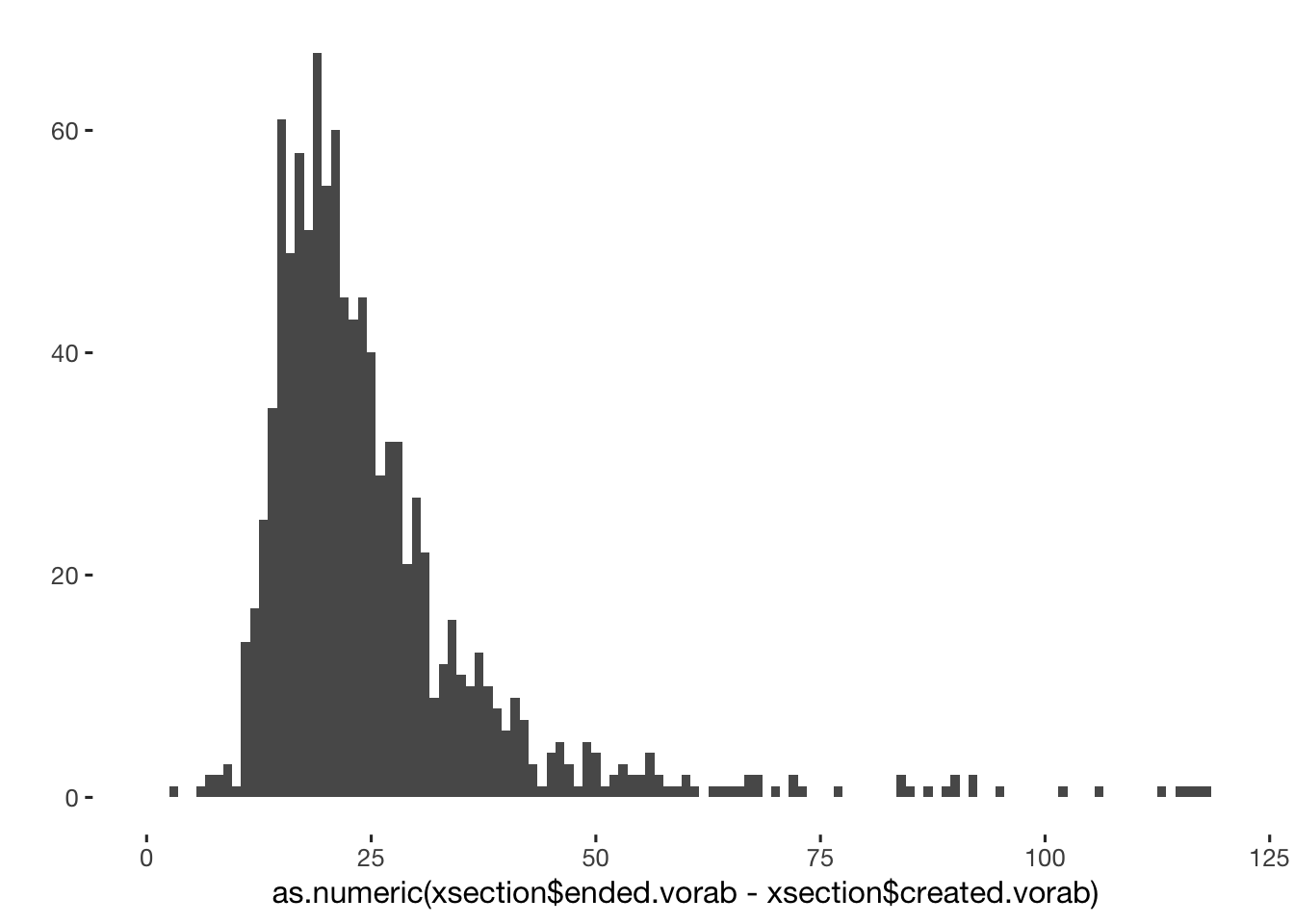qplot(as.numeric(xsection$ended.vorab - xsection$created.vorab)) + xlim(0,NA)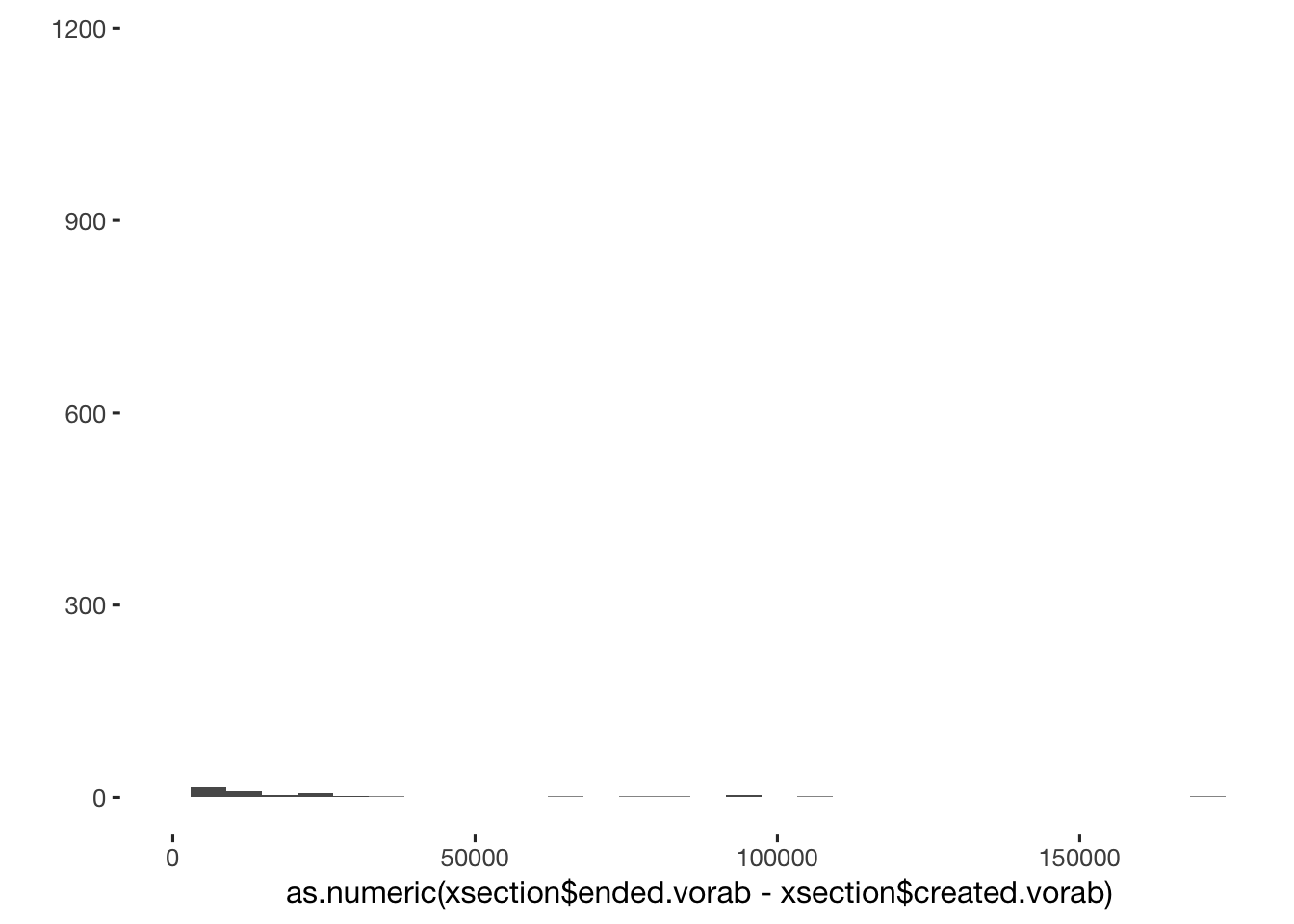#Verhütung count(as.data.frame(xsection), vars=contraception) %>% arrange(desc(n)) %>% head(15) ## # A tibble: 15 x 2 ## # ... with 15 more rows, and 2 variables: vars <chr>, n <int> contrabroad = count(as.data.frame(xsection), vars=contraception) %>% arrange(desc(n)) ## Transformation of contraceptive methods into broader categories xsection[ contraception == "none, contraceptive_pill, morning_after_pill, other_hormonal, infertile, partner_infertile, intrauterine_pessar, kalendermethode, coitus_interruptus, spermicide", contraception := NA] # this one simply clicked everything xsection$contraceptives_broad_categories = xsection$contraception xsection[contraceptives_broad_categories %in% contrabroad[contrabroad$n <= 10, ]$vars, contraceptives_broad_categories := "other"] xsection[ contraception %contains% "temperature_billings", contraceptives_broad_categories := "counting_and_or_temperature_methods"] xsection[ contraception %contains% "kalendermethode", contraceptives_broad_categories := "counting_and_or_temperature_methods"] xsection[ contraception %contains% "computer", contraceptives_broad_categories := "counting_and_or_temperature_methods"] xsection[ contraception %contains% "coitus_interruptus", contraceptives_broad_categories := "occasionally_use_coitus_interruptus"] (contrabroad = count(as.data.frame(xsection), vars=contraceptives_broad_categories) %>% arrange(desc(n))) ## # A tibble: 11 x 2 ## # ... with 11 more rows, and 2 variables: vars <chr>, n <int> # Contraception Categories "barrier or abstinence" vs "fertility awareness" vs "hormonal" xsection[, contraceptive_method := NA_character_] xsection[ contraception %contains% "condoms" | contraception %contains% "intrauterine_pessar" | contraception %contains% "partner_sterilised"| contraception %contains% "partner_infertile" | contraception %contains% "coitus_interruptus" | (contraception == "none" & had_sex_with_partner_yet == "no"), contraceptive_method := "barrier_or_abstinence"] xsection[ contraception %contains% "temperature_billings" | contraception %contains% "kalendermethode" | contraception %contains% "computer", contraceptive_method := "fertility_awareness"] xsection[ contraception %contains% "contraceptive_pill" | contraception %contains% "other_hormonal", contraceptive_method := "hormonal"] xsection[ contraception == "none", contraceptive_method := "none"] xsection[ is.na(contraceptive_method), contraceptive_method := "other"] xsection[, contraceptive_method_by_pearl := NA_character_] xsection[ contraception %contains% "temperature_billings" | contraception %contains% "kalendermethode" | contraception %contains% "computer", contraceptive_method_by_pearl := "fertility_awareness"] xsection[ contraception %contains% "condoms" | contraception %contains% "intrauterine_pessar" | contraception %contains% "partner_sterilised" | contraception %contains% "partner_infertile" | (contraception == "none" & had_sex_with_partner_yet == "no"), contraceptive_method_by_pearl := "barrier_or_abstinence"] xsection[ is.na(contraceptive_method_by_pearl) & contraception %contains% "coitus_interruptus" , contraceptive_method_by_pearl := "barrier_or_abstinence"] xsection[ contraception %contains% "contraceptive_pill" | contraception %contains% "other_hormonal", contraceptive_method_by_pearl := "hormonal"] xsection[ contraception == "none", contraceptive_method_by_pearl := "none"] xsection[ is.na(contraceptive_method_by_pearl), contraceptive_method_by_pearl := "other"] (count(as.data.frame(xsection[contraceptive_method == "other", ]), vars=contraception) %>% arrange(desc(n)) %>% data.frame()) ## vars n ## 1 andere 8 ## 2 sterilised 7 ## 3 2 ## 4 infertile 2 ## 5 none, andere 2 ## 6 infertile, sterilised 1 ## 7 morning_after_pill 1 ## 8 none, infertile 1 ## 9 none, morning_after_pill 1 ## 10 none, sterilised 1 ## 11 <NA> 1 (contramethod = count(as.data.frame(xsection), vars=contraceptive_method) %>% arrange(desc(n)) %>% data.frame()) ## vars n ## 1 hormonal 693 ## 2 barrier_or_abstinence 329 ## 3 none 98 ## 4 fertility_awareness 61 ## 5 other 27 crosstabs(~ contraceptive_method, data = xsection) ## contraceptive_method ## barrier_or_abstinence fertility_awareness hormonal none other ## 329 61 693 98 27 xsection[contraceptive_method == "other",list(contraception, had_sex_with_partner_yet == "yes", age, occupation, wish_for_child==1, breast_feeding==1, pregnant==1)] %>% na.omit()%>% pander() contraception V2 age occupation V5 V6 V7 TRUE 23 Studentin TRUE FALSE FALSE andere TRUE 28 Studentin FALSE FALSE FALSE sterilised TRUE 36 Berufstätig FALSE FALSE FALSE andere FALSE 24 Studentin FALSE FALSE FALSE andere TRUE 23 Studentin FALSE FALSE FALSE andere FALSE 23 Studentin FALSE FALSE FALSE andere TRUE 58 Berufstätig FALSE FALSE FALSE sterilised TRUE 37 Berufstätig FALSE FALSE FALSE none, infertile TRUE 25 Studentin FALSE FALSE FALSE sterilised TRUE 48 Hausfrau FALSE FALSE FALSE andere TRUE 41 Berufstätig FALSE FALSE FALSE infertile TRUE 57 Berufstätig FALSE FALSE FALSE none, sterilised TRUE 53 Berufstätig FALSE FALSE FALSE sterilised TRUE 50 Berufstätig FALSE FALSE FALSE none, andere TRUE 26 Studentin FALSE FALSE FALSE andere TRUE 28 Studentin FALSE TRUE TRUE none, morning_after_pill TRUE 28 Berufstätig FALSE FALSE FALSE sterilised TRUE 53 Berufstätig FALSE FALSE FALSE andere FALSE 19 Studentin FALSE FALSE FALSE sterilised TRUE 49 Berufstätig FALSE FALSE FALSE none, andere TRUE 25 Studentin FALSE FALSE TRUE infertile, sterilised TRUE 49 Berufstätig FALSE FALSE FALSE infertile TRUE 46 Berufstätig FALSE FALSE FALSE morning_after_pill FALSE 21 Studentin FALSE FALSE FALSE sterilised TRUE 50 Berufstätig FALSE FALSE FALSE TRUE 22 Studentin FALSE FALSE FALSE (contramethod = count(as.data.frame(xsection), vars = contraceptive_method) %>% arrange(desc(n)) %>% data.frame()) ## vars n ## 1 hormonal 693 ## 2 barrier_or_abstinence 329 ## 3 none 98 ## 4 fertility_awareness 61 ## 5 other 27 ##Transformation of contraceptive methods into natural and artifical categories # Hier müssen wir nochmal schauen, in welcher Reihenfolge wir das machen wollen. Im Moment werden - glaube ich? - die letzten Befehle als wichtigstes Kriterium eingeschätzt. xsection$contraceptives_categories_natural = xsection$contraception xsection[ contraception == "", contraceptives_categories_natural := NA_character_] xsection[ contraception %contains% "none", contraceptives_categories_natural := "none"] xsection[ contraception %contains% "condoms", contraceptives_categories_natural := "artifical"] xsection[ contraception %contains% "contraceptive_pill", contraceptives_categories_natural := "artifical"] xsection[ contraception %contains% "other_hormonal", contraceptives_categories_natural := "artifical"] xsection[ contraception %contains% "intrauterine_pessar", contraceptives_categories_natural := "artifical"] xsection[ contraception %contains% "morning_after_pill", contraceptives_categories_natural := "artifical"] xsection[ contraception %contains% "coitus_interruptus", contraceptives_categories_natural := "natural"] xsection[ contraception %contains% "kalendermethode", contraceptives_categories_natural := "natural"] xsection[ contraception %contains% "computer", contraceptives_categories_natural := "natural"] xsection[ contraception %contains% "temperature_billings", contraceptives_categories_natural := "natural"] xsection[ contraception %contains% "sterilised", contraceptives_categories_natural := NA_character_] xsection[ contraception %contains% "andere", contraceptives_categories_natural := NA_character_] xsection[ contraception %contains% "partner_sterilised", contraceptives_categories_natural := NA_character_] (contrabroadnatural = count(as.data.frame(xsection), vars=contraceptives_categories_natural) %>% arrange(desc(n))) ## # A tibble: 6 x 2 ## # ... with 6 more rows, and 2 variables: vars <chr>, n <int> ## Transformation of number of children (combined into: "children" and "no children") xsection$children_broad_categories = xsection$children xsection[ children == "", children_broad_categories := 'no_children'] xsection[ children %contains% 1, children_broad_categories := "children"] xsection[ children %contains% 2, children_broad_categories := "children"] xsection[ children %contains% 3, children_broad_categories := "children"] xsection[ children %contains% 4, children_broad_categories := "children"] xsection[ children %contains% 5, children_broad_categories := "no_children"] summary(xsection$children_broad_categories)
##    Length     Class      Mode
##      1208 character character
## Attractiveness_finance
xsection$attractiveness_finance = (xsection$attractiveness_finance_1 + xsection$attractiveness_finance_2)/2 ### last cleaning steps give proper variable labels for plots etc. xsection$breast_feeding_in_last_3_months = ifelse(xsection$breast_feeding == 2, "no", "yes") xsection$pregnant_in_last_3_months = ifelse(xsection$pregnant == 2, "no", "yes") xsection$hormonal_medication_in_last_3_months = ifelse(xsection$antibiotics == 2, "no", "yes") xsection$pill_in_last_3_months = ifelse(xsection$pille_control == 2, "no", "yes") childrenrecode = c("1" = "Biological children", "2" = "Adopted children", "3" = "Step children", "4" = "Foster children", "5" = "No children") # xsection$children_categories = str_replace_all(xsection$children, childrenrecode) xsection$children_narrow_categories = childrenrecode[xsection$children] xsection$children_narrow_categories[is.na(xsection$children_narrow_categories)] = "Complex family" xsection$children_narrow_categories[xsection$children == ""] = "No children" incomebrackets = c("1" = "< 500€", "2" = "500-1000€", "3" = "1000-2000€", "4" = "2000-3000€", "5" = "> 3000€") # xsection$children_categories = str_replace_all(xsection$children, childrenrecode) xsection$income = recode_ordered(incomebrackets, xsection$attractiveness_finance_self) xsection$income_partner = incomebrackets[xsection$attractiveness_finance_2] cohabitation = c("1" = "Long-distance", "2" = "Live in same city", "3" = "Live in same apartment") xsection$cohabitation = cohabitation[xsection$long_distance_relationship] living_situation = c("1" = "living alone", "2" = "living in flatshare") xsection$living_situation = living_situation[xsection$situation_of_living] xsection$living_situation = ifelse(xsection$long_distance_relationship == 3, "living with partner", xsection$living_situation)
xsection$living_situation = ifelse(xsection$living_situation == 'living in flatshare', ifelse(xsection$flat_share == 1, 'living in all-female flatshare', 'living in mixed-sex flatshare'), xsection$living_situation)
days_with_partner_per_month = c("1" = '< 3 days', '2' = '3-5 days', '3' = '5-7 days', '4' = '7-14 days', '5' =  '> 14 days')
xsection$days_with_partner_per_month = recode_ordered(days_with_partner_per_month, xsection$long_distance_relationship_2)
distance_to_partner_hours = c("1" = '< 1h', '2' =   '1-2h', '3' = '2-3h', '4'   = '3-5h', '5' = '5-9h', '6' = '9-12h', '7' = '>12h')
xsection$distance_to_partner_hours = recode_ordered(distance_to_partner_hours, xsection$distance_partner)
days_with_partner = c('1' = '< 3 days', '2' = '3-5 days', '3' = '7 days') # forgot 6...
nights_with_partner = c('1' = '< 3 nights', '2' = '3-5 nights', '3' = '7 nights')
xsection$days_with_partner = recode_ordered(days_with_partner, xsection$days_with_partner)
xsection$nights_with_partner = recode_ordered(nights_with_partner, xsection$nights_with_partner)
xsection$duration_relationship_years = xsection$duration_relationship_total/12
xsection$cycle_length = xsection$cyklus_3
xsection$cycle_length_groups = cut(xsection$cycle_length, breaks = c(19,25,30,35,41))
xsection$certainty_menstruation = factor(round(rowMeans(xsection[, list(cyklus_2, cyklus_4, cyklus_6)]))) xsection$cycle_regularity = factor(xsection$cyklus_5, levels = 1:3, labels = c("very regular,\nup to 2 days off", "slightly irregular,\nup to 5 days off", "irregular,\nmore than 5 days off")) xsection$sexual_intercourse_partner = NULL
xsection$wish_for_child = NULL ## Merge diary data with cross-sectional data prev_names = names(xsection) stopifnot(sum(duplicated(xsection$session)) == 0)
diary = merge(xsection, tagebuch, by = "session", all.x = T)
diary = setDF(diary)
table(is.na(diary$created.vorab)) ## ## FALSE ## 30956 # diary[is.na(created.vorab),list(session, choice_of_clothing_2, created.vorab, created.abschluss)] crosstabs(as.Date(diary$created) == as.Date(diary$created - dhours(9))) # we can left shift dates by 7 hours, because even though were supposed to start filling out before midnight, bugs in our survey software sometimes led to the invitations being sent a little and rarely a lot late) ## as.Date(diary$created) == as.Date(diary$created - dhours(9)) ## FALSE TRUE <NA> ## 188 30736 32 diary = diary %>% filter(!is.na(created.vorab)) diary$weekend = factor(weekdays(  diary$created) %in% c("Friday","Saturday","Sunday")) diary$weekday = factor(weekdays(  diary$created) , levels = c("Monday","Tuesday","Wednesday","Thursday","Friday","Saturday","Sunday")) diary$first_day = ave(diary$created_date, diary$session, FUN = min)
diary$day_number = as.integer(round(as.numeric(diary$created_date - diary$first_day, units = "days"))) combos = diary %>% select(session, day_number) combos$sess_day = paste(combos$session, combos$day_number)
table(duplicated(combos$sess_day)) ## ## FALSE ## 30956 # head(combos) diary$spent_night_with_partner = ifelse(diary$mate_retention_1 == 1, 1, 0) diary$in_pair_public_intimacy = ifelse(diary$mate_retention_2 != 3, 1, 0) diary2 = diary ## Compute menstrual onsets To compute menstrual onsets from the diary data, we have to clear a few hurdles: • diaries could be filled out until 3 am (and later in some special cases), but participants will tend to count backwards from the preceding day when asked when the last menstruation occurred • women could report the same menstrual onset several times (-> use the report closest to the onset, more accurate) • women reported a last menstrual onset in survey preceding the diary and in the follow-up survey following the diary • we need to count backward and forward from each menstrual onset • we need to include the dates from the demographic and the follow-up questionnaire without overwriting more pertinent dates from the diary • we want to “bridge gaps” between reports of menstruation that are at most 40 days wide (because wider gaps probably mean that there was something going on with the menstrual cycle such as a miscarriage, menopause, etc.), but non-reports mean that we cannot use the heuristic that gaps between rows are gaps between days (as days are missing from the diary) Therefore we use a multi-step procedure: 1. Collect unique menstrual onsets reported by each woman 2. Expand the onsets into time-series by participant. 3. “Merge”/prefer reports closer to the onset when several different reports were made 4. Count forward & backward. 5. Merge on participant & created_date. diary = diary2 diary = diary %>% setDF %>% group_by(session) %>% mutate( menstruation_dates = created_date, # get dates menstruation_dates = if_else(menstruation_1 == 2 | is.na(menstruation_1), as.Date(NA), menstruation_dates), # set to missing one dates where no menstruation was reported days = length(unique(created_date)), day_count = seq_along(created), first_diary_date = min(created_date), last_diary_date = max(created_date), timespan = as.numeric( last_diary_date - first_diary_date ), # diary[, timespan := ave(x= created_num, session, FUN = function(x) { round((max(x,na.rm=T)-min(x,na.rm=T))/(24*3600)) } )] days_responded_percentage = ifelse( timespan==0, NA_real_, days/timespan), sufficient_diary_coverage = days >= 30 & days_responded_percentage > 0.5, last_menstruation = if_else(all(is.na(menstruation_dates)), as.Date(NA), max(menstruation_dates, na.rm=T)), last_lag = ifelse(is.na(last_menstruation), NA_real_, as.numeric(last_diary_date - last_menstruation)) # how many days after their last observed menstruation did they last fill out the diary ) %>% data.table() crosstabs(diary$menstruation_2)
## diary$menstruation_2 ## 1 2 3 4 5 6 <NA> ## 781 897 824 800 766 726 26162 diary[, days_since_menstrual_onset := ifelse(menstruation_2 == 6, NA_real_, menstruation_2 - 1 )] # the way we collected this variable, we have to set "more than 4 days ago" to missing, because it's unspecific diary[, menstrual_onset_date_inferred := created_date - ddays(days_since_menstrual_onset)] ### count back from each current diary day, to create a menstrual onset date crosstabs(is.na(diary$menstrual_onset_date_inferred))
## is.na(diary$menstrual_onset_date_inferred) ## FALSE TRUE ## 4068 26888 ### define a variable containing the number of days since the last menstruation as counted on the current diary day # step 1 menstrual_onsets = diary %>% setDF() %>% group_by(session) %>% arrange(created) %>% select(session, created_date, menstrual_onset_date_inferred) followup_survey = nachbe %>% setDF() %>% mutate( menstrual_onset_date_inferred = if_else(menstrual_bleeding_1 == 1, as.Date(created), as.Date(menstrual_bleeding_2)), created_date = menstrual_onset_date_inferred) %>% select(session, created_date, menstrual_onset_date_inferred) pre_survey = vorab %>% setDF() %>% mutate(menstrual_onset_date_inferred = as.Date(cyklus_1), created_date = menstrual_onset_date_inferred) %>% select(session, created_date, menstrual_onset_date_inferred) mons = menstrual_onsets %>% select(session, created_date, menstrual_onset_date_inferred) %>% bind_rows( pre_survey, followup_survey ) %>% filter( !is.na(menstrual_onset_date_inferred)) %>% unique() %>% group_by(session) %>% arrange(session, created_date) %>% # step 3: prefer reports closer to event if they conflict mutate( onset_diff = abs( as.double( lag(menstrual_onset_date_inferred) - menstrual_onset_date_inferred, units = "days")), # was there a change in the reference menstrual onset onset_diff = if_else(onset_diff == 0, NA_real_, onset_diff, NA_real_), # we want to take the date that is freshest in memory. no switch is set to missing menstrual_onset_date_inferred = if_else(is.na(lag(menstrual_onset_date_inferred)), # if last date not known menstrual_onset_date_inferred, # use current date if_else(onset_diff < 7, # if last date is known, but is slightly different from current date as.Date(NA), # attribute it to memory, not extremely short cycle, use fresher date menstrual_onset_date_inferred, # if it's a big difference, use the current date as.Date(NA))), # if no date is assigned today, keep it like that menstrual_onset_date_inferred = formr::repeat_last(menstrual_onset_date_inferred)) %>% mutate(created_date = menstrual_onset_date_inferred) %>% filter(!is.na(menstrual_onset_date_inferred)) %>% select(-onset_diff) %>% unique() avg_cycle_lengths = mons %>% select(session, menstrual_onset_date_inferred) %>% unique() %>% arrange(session, menstrual_onset_date_inferred) %>% mutate( number_of_cycles = n(), cycle_nr = row_number(), cycle_length_diary = as.double(lead(menstrual_onset_date_inferred) - menstrual_onset_date_inferred, units = "days"), mean_cycle_length_diary = mean(cycle_length_diary, na.rm = TRUE), median_cycle_length_diary = median(cycle_length_diary, na.rm = TRUE)) menstrual_onsets = menstrual_onsets %>% select(session, created_date) %>% bind_rows( diary = ., demo = pre_survey %>% select(session, created_date), followup = followup_survey %>% select(session, created_date), .id = "date_origin") %>% filter(!is.na(created_date)) %>% unique() %>% group_by(session) %>% arrange(session, created_date) %>% # step 2 expand into time-series for participant complete(created_date = full_seq(created_date, period = 1)) %>% left_join(mons, by = c("session", "created_date")) %>% unique() menstrual_onsets = menstrual_onsets %>% group_by(session) %>% mutate( # carry the last observation (the last observed menstrual onset) backward/forward (within person). # first we carry it backward (because reporting is retrospective) next_menstrual_onset = formr::repeat_last(menstrual_onset_date_inferred, forward = FALSE), # then we carry it forward last_menstrual_onset = formr::repeat_last(menstrual_onset_date_inferred), # calculate the diff to current date menstrual_onset_days_until = as.numeric(created_date - next_menstrual_onset), menstrual_onset_days_since = as.numeric(created_date - last_menstrual_onset) ) # mons %>% filter(session %contains% "43a25b") %>% View() # menstrual_onsets %>% filter(session %contains% "43a25b") %>% View() # menstrual_onsets %>% filter(session %starts_with% "020f65ea45") %>% View() diary = diary %>% setDF() %>% left_join(menstrual_onsets %>% select(session, created_date, next_menstrual_onset, last_menstrual_onset, menstrual_onset_days_until, menstrual_onset_days_since) %>% unique() # unique because some women reported the last menstrual date in the diary again in the followup , by = c("session", "created_date") ) %>% left_join(avg_cycle_lengths %>% rename(last_menstrual_onset = menstrual_onset_date_inferred), by = c("session", "last_menstrual_onset")) %>% mutate( next_menstrual_onset_inferred = if_else(is.na(next_menstrual_onset), last_menstrual_onset + days(cyklus_3), next_menstrual_onset), RCD_inferred = as.numeric(created_date - next_menstrual_onset_inferred) ) %>% data.table() diary[!duplicated(session) & median_cycle_length_diary <= 40 & median_cycle_length_diary >= 20, qplot(median_cycle_length_diary, cyklus_3) + geom_smooth()]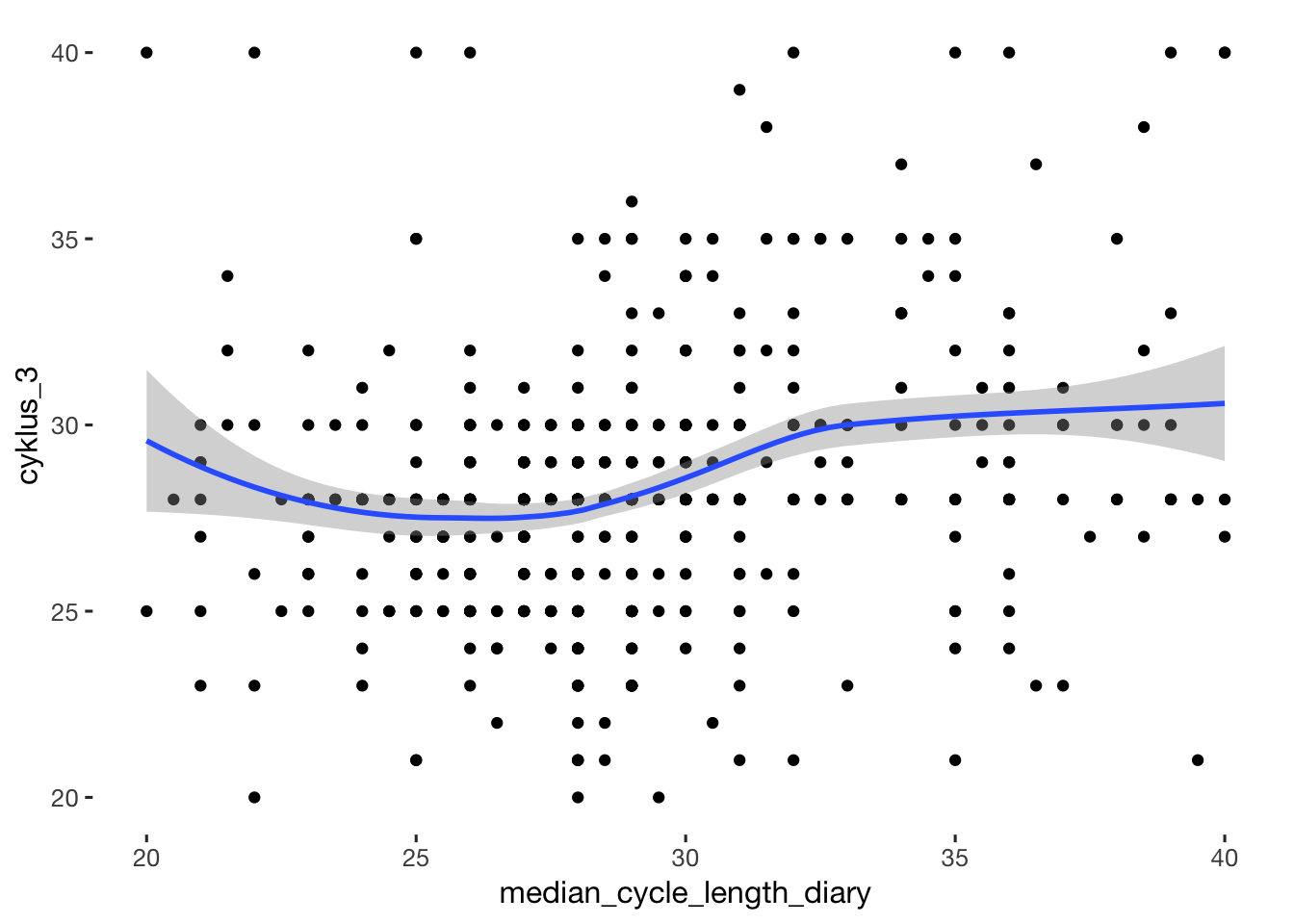diary[!duplicated(session) & median_cycle_length_diary <= 40 & median_cycle_length_diary >= 20, lm(median_cycle_length_diary ~ cyklus_3)] ## ## Call: ## lm(formula = median_cycle_length_diary ~ cyklus_3) ## ## Coefficients: ## (Intercept) cyklus_3 ## 20.681 0.287 # diary %>% filter(session %starts_with% "020f65ea45") %>% select(created_date, next_menstrual_onset, last_menstrual_onset, menstrual_onset_days_until, menstrual_onset_days_since) %>% View() xtabs(~ is.na(menstrual_onset_days_until) + is.na(menstrual_onset_days_since), diary) ## is.na(menstrual_onset_days_since) ## is.na(menstrual_onset_days_until) FALSE TRUE ## FALSE 18614 0 ## TRUE 12310 32 diary = diary %>% mutate( FCD = menstrual_onset_days_since + 1, RCD = menstrual_onset_days_until, RCD_for_merge = (RCD * -1) + 1, RCD_squished = if_else( cycle_length_diary - FCD < 14, 29 - (cycle_length_diary - FCD), round((FCD/ (cycle_length_diary - 14) ) * 15)), RCD_inferred = (RCD_inferred * -1) + 1, # add 15 days to the reverse cycle days to arrive at the estimated day of ovulation RCD_rel_to_ovulation = RCD + 15 ) crosstabs(diary$RCD_squished)
## diary$RCD_squished ## 0 1 2 3 4 5 6 7 8 9 10 11 12 13 14 15 16 17 ## 74 426 444 382 437 458 467 310 547 488 534 509 641 574 663 643 606 612 ## 18 19 20 21 22 23 24 25 26 27 28 29 <NA> ## 663 668 694 716 762 768 801 780 820 828 846 857 12938 crosstabs(diary$RCD_for_merge)
## diary$RCD_for_merge ## 1 2 3 4 5 6 7 8 9 10 11 12 13 14 15 16 17 18 ## 934 857 846 828 820 780 801 768 759 715 693 667 661 609 601 582 563 542 ## 19 20 21 22 23 24 25 26 27 28 29 30 31 32 33 34 35 36 ## 529 499 477 449 419 401 370 342 305 252 159 119 100 77 65 49 49 44 ## 37 38 39 40 41 42 43 44 45 46 47 48 49 50 51 52 53 54 ## 37 34 24 33 28 31 32 25 27 24 17 19 14 21 20 18 12 16 ## 55 56 57 58 59 60 61 62 63 64 65 66 67 68 69 70 71 72 ## 15 10 13 8 10 9 9 11 7 8 4 5 8 8 7 8 10 9 ## 73 74 75 76 77 78 79 80 81 82 83 84 85 86 87 88 89 90 ## 9 7 9 8 7 5 9 4 5 3 4 2 2 6 6 5 3 2 ## 91 92 93 94 95 96 97 98 99 100 101 102 103 104 105 106 107 108 ## 5 3 5 3 4 4 3 2 3 2 5 3 6 5 2 1 2 1 ## 109 112 113 114 115 117 118 120 121 122 123 124 125 126 127 128 129 130 ## 3 1 2 1 2 1 1 2 1 2 1 1 1 2 1 1 2 2 ## 131 132 133 134 135 136 137 138 139 140 141 142 143 144 145 150 151 155 ## 2 1 2 3 1 2 2 2 1 1 1 1 2 2 2 1 1 1 ## 156 157 158 159 161 163 164 166 167 168 170 171 174 175 178 179 182 183 ## 1 1 2 2 1 1 1 1 1 1 1 1 2 1 1 1 1 1 ## 186 187 189 190 191 192 193 194 195 196 197 198 199 200 201 203 205 208 ## 2 1 2 1 1 1 1 1 1 1 2 2 1 1 1 1 1 1 ## 209 210 211 218 224 227 242 248 249 250 251 252 253 265 282 300 301 302 ## 1 1 1 1 1 1 1 1 1 1 1 1 1 1 1 1 1 1 ## 315 317 318 319 323 344 347 348 349 350 351 352 353 354 355 356 357 358 ## 1 1 1 1 1 1 1 1 1 1 2 1 1 1 1 1 1 1 ## 359 360 <NA> ## 1 1 12342 crosstabs(diary$FCD)
## diary$FCD ## 1 2 3 4 5 6 7 8 9 10 11 12 13 14 15 16 17 18 19 20 21 22 ## 934 951 928 978 1003 951 938 978 957 960 939 939 936 948 923 899 933 883 896 924 882 872 ## 23 24 25 26 27 28 29 30 31 32 33 34 35 36 37 38 39 40 41 42 43 44 ## 861 849 812 743 723 644 415 271 215 195 172 150 142 140 116 101 97 90 92 85 91 82 ## 45 46 47 48 49 50 51 52 53 54 55 56 57 58 59 60 61 62 63 64 65 66 ## 91 82 77 84 80 70 64 58 63 65 56 54 38 45 41 36 47 49 75 77 74 73 ## 67 68 69 70 71 72 73 74 75 76 77 78 79 80 81 82 83 84 85 86 87 88 ## 63 67 63 65 51 51 58 50 49 56 49 45 52 43 44 38 42 36 36 40 34 33 ## 89 90 91 92 93 94 95 96 97 98 99 100 101 102 103 104 105 106 107 108 109 110 ## 35 32 28 31 31 32 30 26 27 21 26 26 21 21 14 19 13 8 8 10 8 9 ## 111 112 113 114 115 116 117 118 119 120 121 122 123 124 125 126 127 128 129 130 131 132 ## 4 6 4 5 5 4 6 10 6 6 5 2 1 2 6 4 4 2 7 3 5 3 ## 133 134 135 136 137 138 139 140 141 142 143 144 145 146 147 148 149 150 151 152 153 154 ## 8 2 3 2 4 2 4 3 6 3 3 2 4 5 2 2 2 1 1 2 2 2 ## 155 156 157 158 159 160 161 162 163 164 165 166 167 168 169 170 171 172 173 174 175 176 ## 2 2 4 5 2 1 3 4 3 4 1 3 1 2 3 2 1 2 1 4 2 2 ## 178 179 181 183 186 188 192 195 196 197 198 200 201 204 206 207 208 209 211 214 216 218 ## 2 1 1 1 1 2 2 2 1 3 1 1 2 1 1 1 1 1 3 2 2 3 ## 220 221 222 223 225 229 233 234 235 236 239 240 242 245 247 250 251 252 257 258 260 261 ## 1 2 1 1 2 1 1 1 1 1 1 1 1 1 1 2 1 1 1 2 3 1 ## 262 263 264 265 267 268 271 272 275 280 281 283 284 285 292 294 299 300 301 303 304 310 ## 1 1 1 1 3 1 2 2 1 2 2 2 1 1 1 2 1 1 2 2 1 1 ## 311 312 313 318 321 322 326 329 330 331 332 333 334 336 337 338 342 351 375 396 397 398 ## 1 2 1 2 1 1 1 1 2 1 1 1 1 1 1 2 1 2 1 1 1 1 ## 404 432 440 483 615 <NA> ## 1 1 1 1 1 32 crosstabs(~RCD_squished+ RCD_for_merge, diary %>% filter(cycle_length_diary<40)) ## RCD_for_merge ## RCD_squished 1 2 3 4 5 6 7 8 9 10 11 12 13 14 15 16 17 18 19 20 21 22 23 24 ## 1 255 0 0 0 0 0 0 0 0 0 0 0 0 0 0 0 0 0 0 0 0 0 0 0 ## 2 21 0 0 0 0 0 0 0 0 0 0 0 0 0 0 0 0 0 0 0 0 0 0 0 ## 3 4 0 0 0 0 0 0 0 0 0 0 0 0 0 0 0 0 0 0 0 0 0 8 7 ## 4 4 0 0 0 0 0 0 0 0 0 0 0 0 0 0 0 0 0 0 0 3 4 7 19 ## 5 3 0 0 0 0 0 0 0 0 0 0 0 0 0 0 0 0 0 0 2 0 9 18 31 ## 6 0 0 0 0 0 0 0 0 0 0 0 0 0 0 0 0 0 0 3 3 4 6 35 146 ## 7 0 0 0 0 0 0 0 0 0 0 0 0 0 0 0 0 0 0 0 0 10 20 48 55 ## 8 1 0 0 0 0 0 0 0 0 0 0 0 0 0 0 0 0 3 3 16 27 78 184 62 ## 9 0 0 0 0 0 0 0 0 0 0 0 0 0 0 0 0 0 4 6 8 91 205 47 25 ## 10 0 0 0 0 0 0 0 0 0 0 0 0 0 0 0 0 2 4 17 116 216 56 25 19 ## 11 0 0 0 0 0 0 0 0 0 0 0 0 0 0 0 0 2 5 59 250 64 28 4 0 ## 12 0 0 0 0 0 0 0 0 0 0 0 0 0 0 0 0 9 82 339 62 17 0 0 0 ## 13 0 0 0 0 0 0 0 0 0 0 0 0 0 0 0 0 17 358 56 2 0 0 0 0 ## 14 0 0 0 0 0 0 0 0 0 0 0 0 0 0 0 0 481 32 0 0 0 0 0 0 ## 15 0 0 0 0 0 0 0 0 0 0 0 0 0 0 0 533 0 0 0 0 0 0 0 0 ## 16 5 0 0 0 0 0 0 0 0 0 0 0 0 0 542 0 0 0 0 0 0 0 0 0 ## 17 3 0 0 0 0 0 0 0 0 0 0 0 0 555 0 0 0 0 0 0 0 0 0 0 ## 18 2 0 0 0 0 0 0 0 0 0 0 0 599 0 0 0 0 0 0 0 0 0 0 0 ## 19 1 0 0 0 0 0 0 0 0 0 0 608 0 0 0 0 0 0 0 0 0 0 0 0 ## 20 1 0 0 0 0 0 0 0 0 0 629 0 0 0 0 0 0 0 0 0 0 0 0 0 ## 21 1 0 0 0 0 0 0 0 0 644 0 0 0 0 0 0 0 0 0 0 0 0 0 0 ## 22 3 0 0 0 0 0 0 0 681 0 0 0 0 0 0 0 0 0 0 0 0 0 0 0 ## 23 0 0 0 0 0 0 0 685 0 0 0 0 0 0 0 0 0 0 0 0 0 0 0 0 ## 24 0 0 0 0 0 0 720 0 0 0 0 0 0 0 0 0 0 0 0 0 0 0 0 0 ## 25 0 0 0 0 0 703 0 0 0 0 0 0 0 0 0 0 0 0 0 0 0 0 0 0 ## 26 0 0 0 0 742 0 0 0 0 0 0 0 0 0 0 0 0 0 0 0 0 0 0 0 ## 27 0 0 0 749 0 0 0 0 0 0 0 0 0 0 0 0 0 0 0 0 0 0 0 0 ## 28 0 0 772 0 0 0 0 0 0 0 0 0 0 0 0 0 0 0 0 0 0 0 0 0 ## 29 0 772 0 0 0 0 0 0 0 0 0 0 0 0 0 0 0 0 0 0 0 0 0 0 ## RCD_for_merge ## RCD_squished 25 26 27 28 29 30 31 32 33 34 35 36 37 38 ## 1 0 0 0 0 0 0 0 0 0 0 6 4 5 1 ## 2 0 29 36 79 41 20 25 20 13 13 6 8 2 0 ## 3 16 39 83 40 19 28 10 6 11 10 6 0 0 0 ## 4 30 92 48 40 31 19 10 9 6 1 0 0 0 0 ## 5 133 46 48 10 17 11 12 8 1 0 0 0 0 0 ## 6 56 46 23 25 11 6 5 0 0 0 0 0 0 0 ## 7 48 25 16 13 6 1 0 0 0 0 0 0 0 0 ## 8 29 27 17 5 0 0 0 0 0 0 0 0 0 0 ## 9 21 4 0 0 0 0 0 0 0 0 0 0 0 0 ## 10 1 0 0 0 0 0 0 0 0 0 0 0 0 0 ## 11 0 0 0 0 0 0 0 0 0 0 0 0 0 0 ## 12 0 0 0 0 0 0 0 0 0 0 0 0 0 0 ## 13 0 0 0 0 0 0 0 0 0 0 0 0 0 0 ## 14 0 0 0 0 0 0 0 0 0 0 0 0 0 0 ## 15 0 0 0 0 0 0 0 0 0 0 0 0 0 0 ## 16 0 0 0 0 0 0 0 0 0 0 0 0 0 0 ## 17 0 0 0 0 0 0 0 0 0 0 0 0 0 0 ## 18 0 0 0 0 0 0 0 0 0 0 0 0 0 0 ## 19 0 0 0 0 0 0 0 0 0 0 0 0 0 0 ## 20 0 0 0 0 0 0 0 0 0 0 0 0 0 0 ## 21 0 0 0 0 0 0 0 0 0 0 0 0 0 0 ## 22 0 0 0 0 0 0 0 0 0 0 0 0 0 0 ## 23 0 0 0 0 0 0 0 0 0 0 0 0 0 0 ## 24 0 0 0 0 0 0 0 0 0 0 0 0 0 0 ## 25 0 0 0 0 0 0 0 0 0 0 0 0 0 0 ## 26 0 0 0 0 0 0 0 0 0 0 0 0 0 0 ## 27 0 0 0 0 0 0 0 0 0 0 0 0 0 0 ## 28 0 0 0 0 0 0 0 0 0 0 0 0 0 0 ## 29 0 0 0 0 0 0 0 0 0 0 0 0 0 0 ggplot(diary %>% filter(cycle_length_diary<40), aes(FCD, RCD_squished)) + geom_point() + facet_wrap(~cycle_length_diary)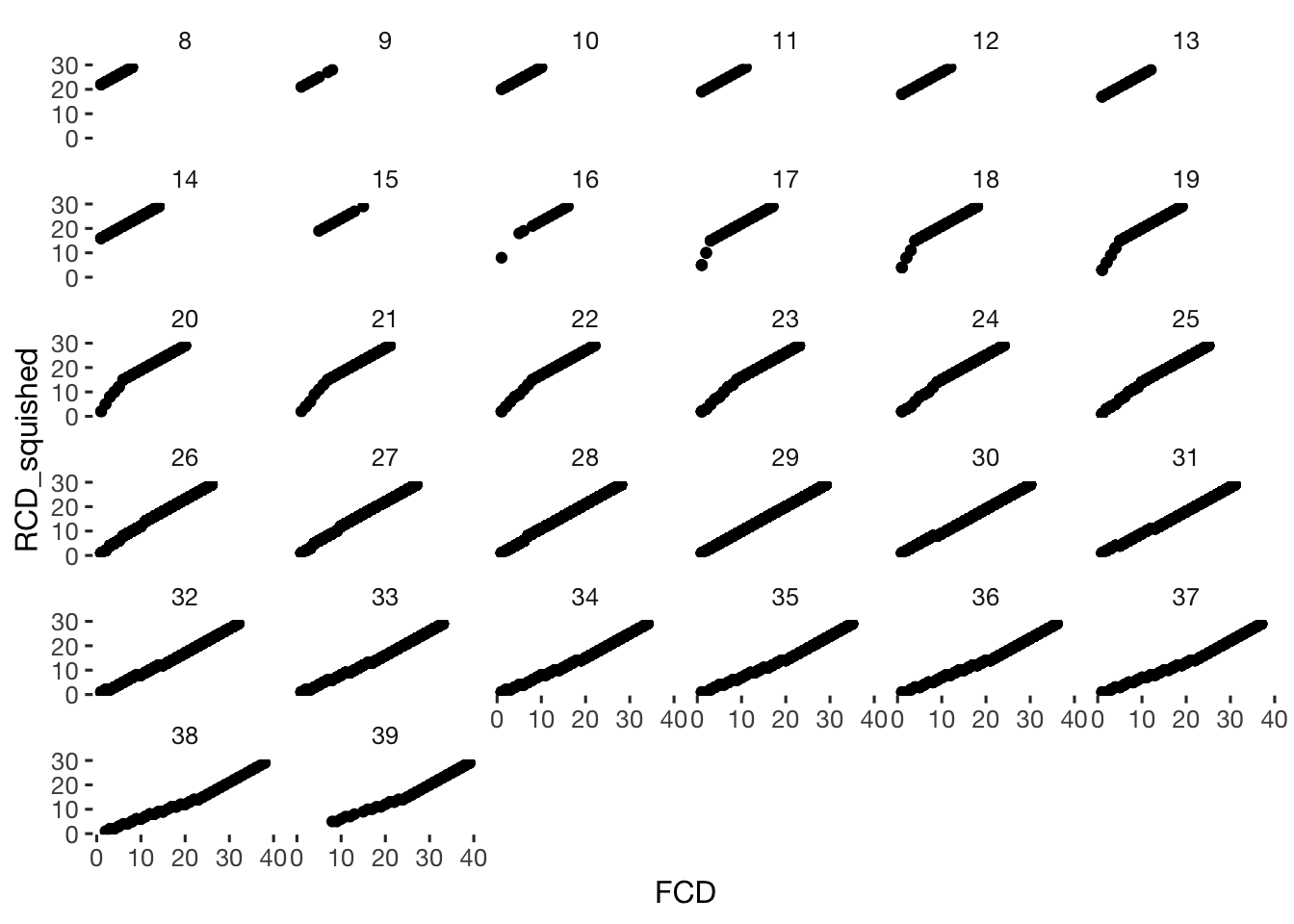days = data.frame( RCD_for_merge = c(29:1, 30:41), FCD = c(1:41), prc_stirn_b = c(.01, .01, .02, .03, .05, .09, .16, .27, .38, .48, .56, .58, .55, .48, .38, .28, .20, .14, .10, .07, .06, .04, .03, .02, .01, .01, .01, .01, .01, rep(.01, times = 12)), # rep(.01, times = 70)), # gangestad uses .01 here, but I think such cases are better thrown than kept, since we might simply have missed a mens prc_wcx_b = c(.000, .000, .001, .002, .004, .009, .018, .032, .050, .069, .085, .094, .093, .085, .073, .059, .047, .036, .028, .021, .016, .013, .010, .008, .007, .006, .005, .005, .005, rep(.005, times = 12)) ) # rep(NA_real_, times = 70)) # gangestad uses .005 here, but I think such cases are better thrown than kept, since we might simply have missed a mens days = days %>% mutate( fertile_narrow = ifelse(between(RCD_for_merge,15,19), mean(prc_stirn_b[between(RCD_for_merge,15, 19)], na.rm = T), ifelse(between(RCD_for_merge,4,12), mean(prc_stirn_b[between(RCD_for_merge,4,12)], na.rm = T), NA_real_)), # these days are likely infertile fertile_broad = ifelse(between(RCD_for_merge,14,22), mean(prc_stirn_b[between(RCD_for_merge,14,22)], na.rm = T), ifelse(between(RCD_for_merge,4,12), mean(prc_stirn_b[between(RCD_for_merge,4,12)], na.rm = T), NA_real_)), # these days are likely infertile fertile_window = factor(ifelse(fertile_broad, ifelse(!is.na(fertile_narrow), "narrow", "broad"),"infertile"), levels = c("infertile","broad", "narrow")), premenstrual_phase = ifelse(between(RCD_for_merge, 2, 7), TRUE, FALSE) ) rcd_days = days %>% select(-FCD) diary = left_join(diary %>% setDF(), rcd_days, by = "RCD_for_merge") fcd_days = days %>% select(-RCD_for_merge) names(fcd_days) = paste0(names(fcd_days), "_forward_counted") fcd_days = fcd_days %>% rename(FCD = FCD_forward_counted) diary = left_join(diary, fcd_days, by = "FCD") rcd_inferred_days = days %>% select(-FCD) names(rcd_inferred_days) = paste0(names(rcd_inferred_days), "_backward_inferred") rcd_inferred_days = rcd_inferred_days %>% rename(RCD_inferred = RCD_for_merge_backward_inferred) diary = left_join(diary, rcd_inferred_days, by = "RCD_inferred") rcd_squished = days %>% select(-RCD_for_merge) names(rcd_squished) = paste0(names(rcd_squished), "_squished") rcd_squished = rcd_squished %>% rename(RCD_squished = FCD_squished) diary = left_join(diary, rcd_squished, by = "RCD_squished") ggplot(diary %>% filter(cycle_length_diary<40), aes(FCD, prc_stirn_b_squished))+geom_point() + facet_wrap(~cycle_length_diary )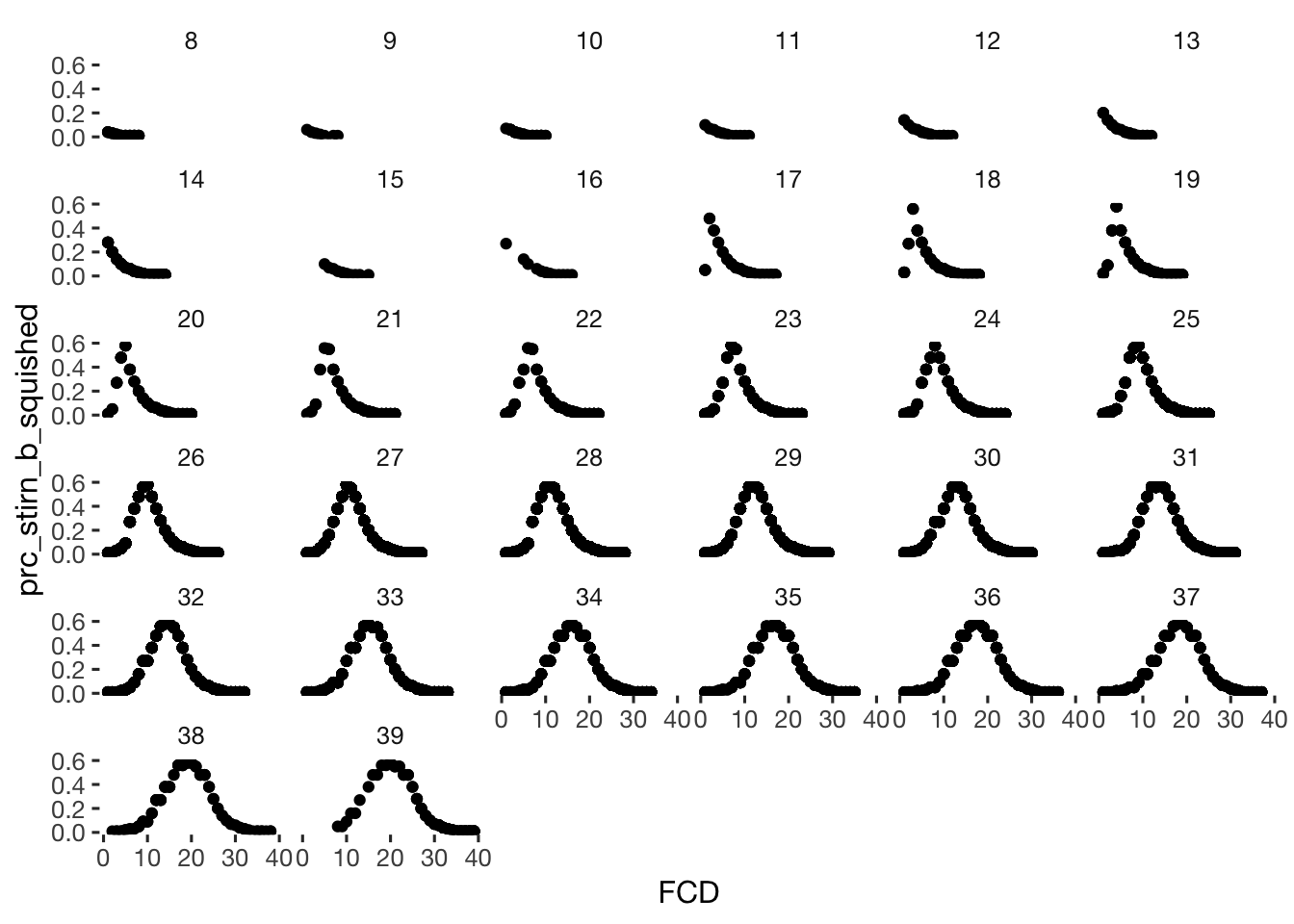ggplot(diary %>% filter(cycle_length_diary<40), aes(RCD, prc_stirn_b_squished))+geom_point() + facet_wrap(~cycle_length_diary )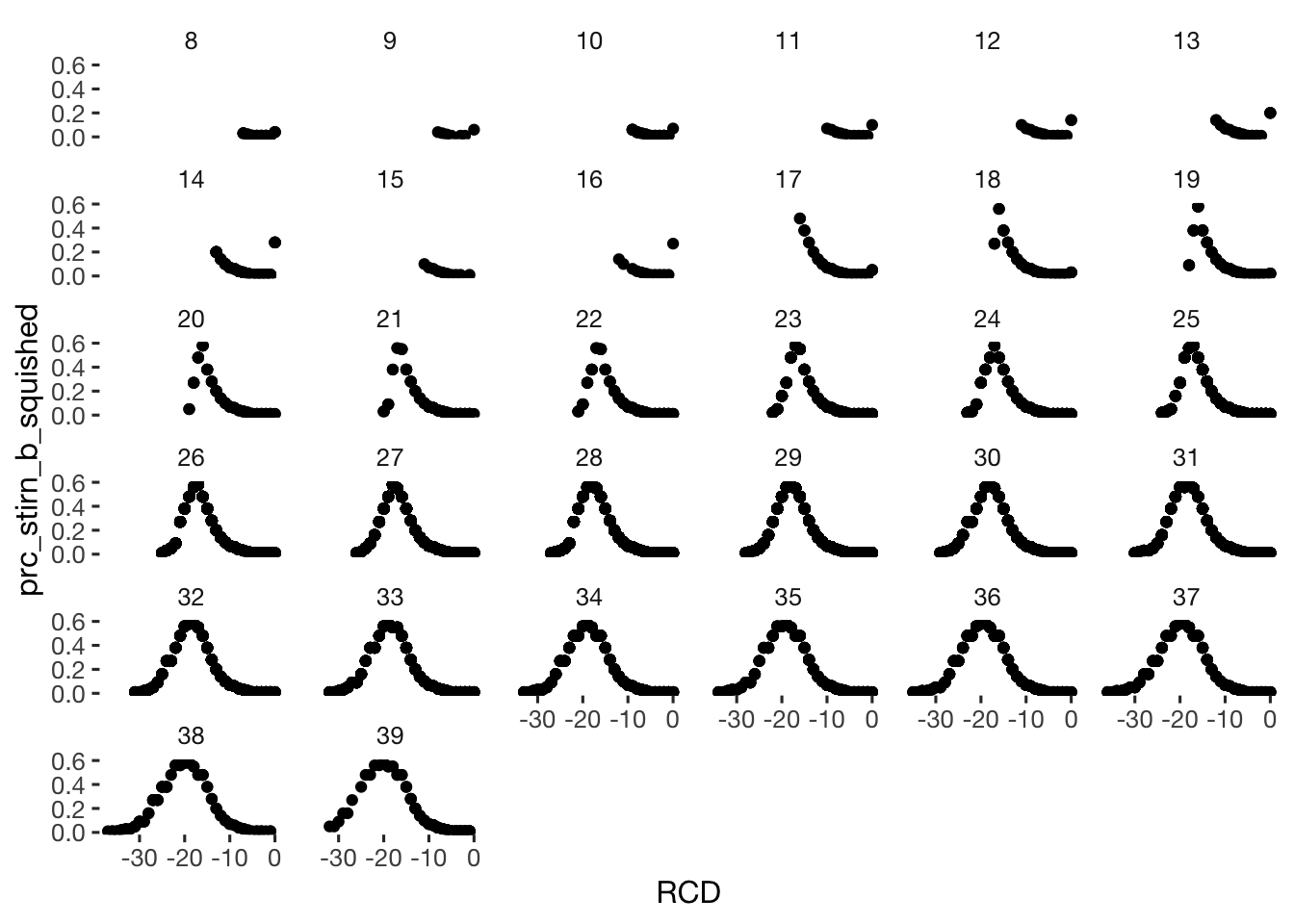ggplot(diary %>% filter(cycle_length_diary<40) %>% select(FCD,RCD,prc_stirn_b,prc_stirn_b_squished,cycle_length_diary) %>% gather(approach,prc_stirn_b,-RCD,-FCD, -cycle_length_diary), aes(RCD, prc_stirn_b, colour = approach))+geom_point() + facet_wrap(~cycle_length_diary ) + geom_vline(aes(xintercept = cycle_length_diary/2 * -1))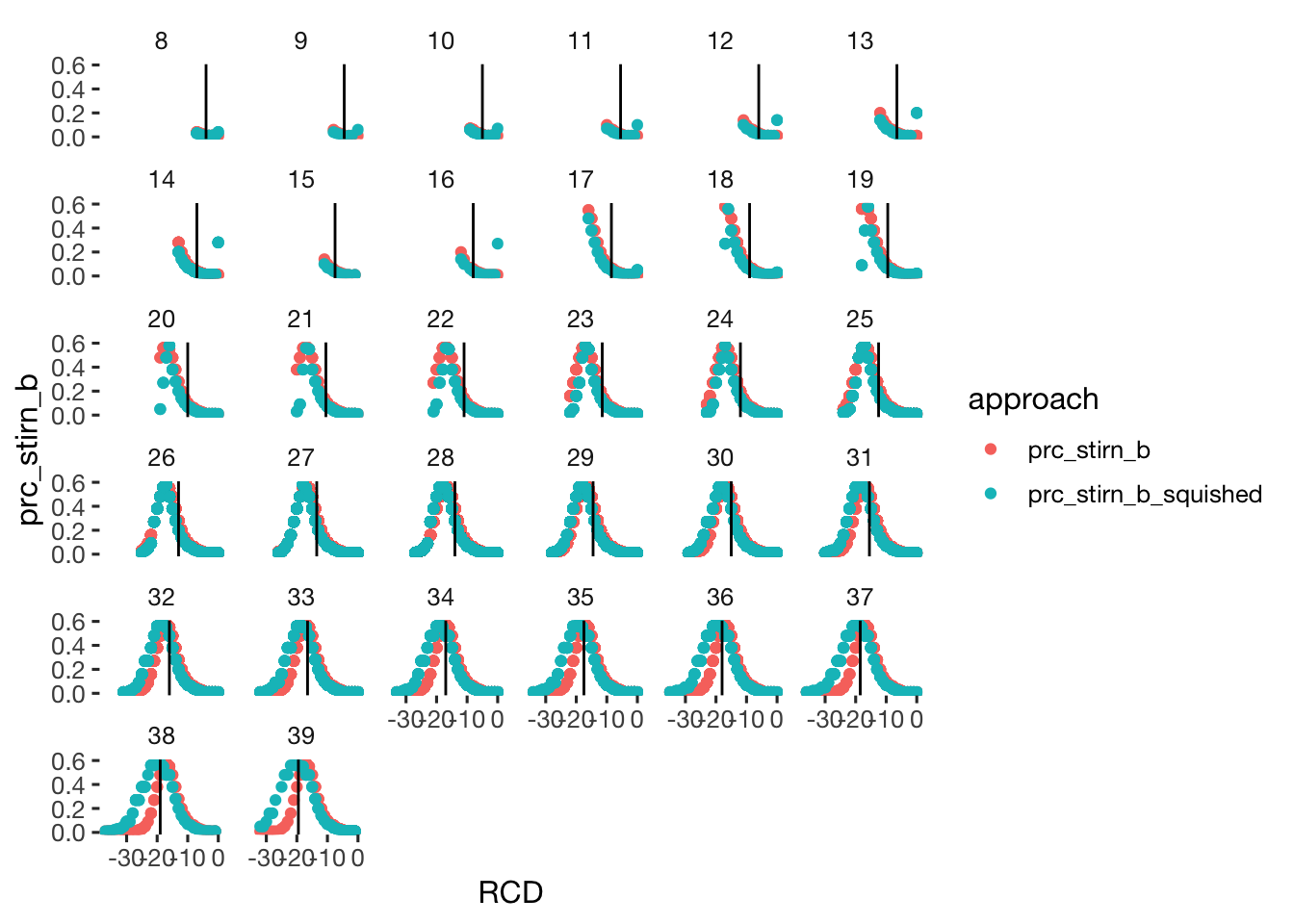diary %>% select(starts_with("prc_stirn_b")) %>% cor(use='pairwise.complete.obs') ## prc_stirn_b prc_stirn_b_forward_counted prc_stirn_b_backward_inferred ## prc_stirn_b 1.0000 0.6846 1.0000 ## prc_stirn_b_forward_counted 0.6846 1.0000 0.7153 ## prc_stirn_b_backward_inferred 1.0000 0.7153 1.0000 ## prc_stirn_b_squished 0.8578 0.6695 0.8578 ## prc_stirn_b_squished ## prc_stirn_b 0.8578 ## prc_stirn_b_forward_counted 0.6695 ## prc_stirn_b_backward_inferred 0.8578 ## prc_stirn_b_squished 1.0000 pander(missingness_patterns(diary %>% ungroup %>% select(prc_stirn_b, prc_stirn_b_forward_counted, prc_stirn_b_backward_inferred))) index col missings 1 prc_stirn_b 13069 2 prc_stirn_b_backward_inferred 3813 3 prc_stirn_b_forward_counted 3576 Pattern Freq Culprit _____ 16782 _ 1____ 9256 prc_stirn_b 1_2_3 2471 1_2__ 1342 ____3 1105 prc_stirn_b_forward_counted diary = diary %>% mutate(fertile_fab = if_else(is.na(prc_stirn_b), prc_stirn_b_backward_inferred, prc_stirn_b), premenstrual_phase_fab = if_else(is.na(premenstrual_phase), premenstrual_phase_backward_inferred, premenstrual_phase), fertile_forward_and_backward = fertile_fab ) %>% data.table() diary[, menstruated_at_all := menstruation_1 == 1] diary[ is.na(menstruated_at_all), menstruated_at_all := F] table(diary$menstruated_at_all, exclude = NULL)
##
## FALSE  TRUE
## 26151  4805
diary[, menstruation := factor(ifelse(menstruated_at_all,"yes", ifelse(premenstrual_phase, "pre","no")), levels = c("no", "pre", "yes"))]
diary[is.na(menstruation), menstruation := "no"]

# diary %>% select(short, created_date, ended, last_menstrual_onset,next_menstrual_onset, menstrual_onset_days_until, menstrual_onset_days_since, prc_stirn_b, prc_stirn_b_forward_counted, fertile_fab)  %>% View()

### Find fertile window

diary[, ever_menstruated := ave(menstruated_at_all, session, FUN = any)]
diary[, any_RCD := !ave(is.na(RCD), session, FUN = all)]
diary[, menstruation_strength := menstruation_3]
diary[ is.na(menstruation_strength), menstruation_strength := 0]
diary[, menstruation_strength := factor(menstruation_strength)]
diary[, menstrual_onset := created_date == next_menstrual_onset]
diary[, we_know_fertile_days := ave(!is.na(prc_stirn_b) | !is.na(prc_stirn_b_forward_counted), session, FUN = any)]
crosstabs(diary[!duplicated(session),]$we_know_fertile_days) ## diary[!duplicated(session), ]$we_know_fertile_days
## FALSE  TRUE
##    96  1112
diary$fertile_cont = diary$prc_stirn_b

diary %>% filter(sufficient_diary_coverage==T & ever_menstruated == T & any_RCD == T) %>%
ggplot(aes(x = menstrual_onset_days_until, y = as.factor(as.numeric(as.factor(session)))))+
scale_y_discrete("Person") +
geom_tile(aes(fill = fertile_cont)) +
scale_x_continuous("Relative to last observed menstrual onset", limits= c(-40, 15), breaks = seq(-40,15, by = 5)) +
geom_point(aes(colour=menstruation_strength)) +
scale_fill_distiller() +
scale_color_manual(values = c("0"="transparent","1"="pink","2"="red","3"="darkred"))  +
geom_vline(aes(xintercept = limits, linetype = Window),data = data.frame(limits= c(-14.5,-19.5, -13.5,-22.5,-3.5,-12.5), Window = rep(c("narrow","broad","infertile"),each=2)),color = 'black', size = 0.9, alpha = 0.9,show.legend = T) +
scale_linetype_manual(values = c("narrow"="solid","broad"="dashed","infertile"="dotted"))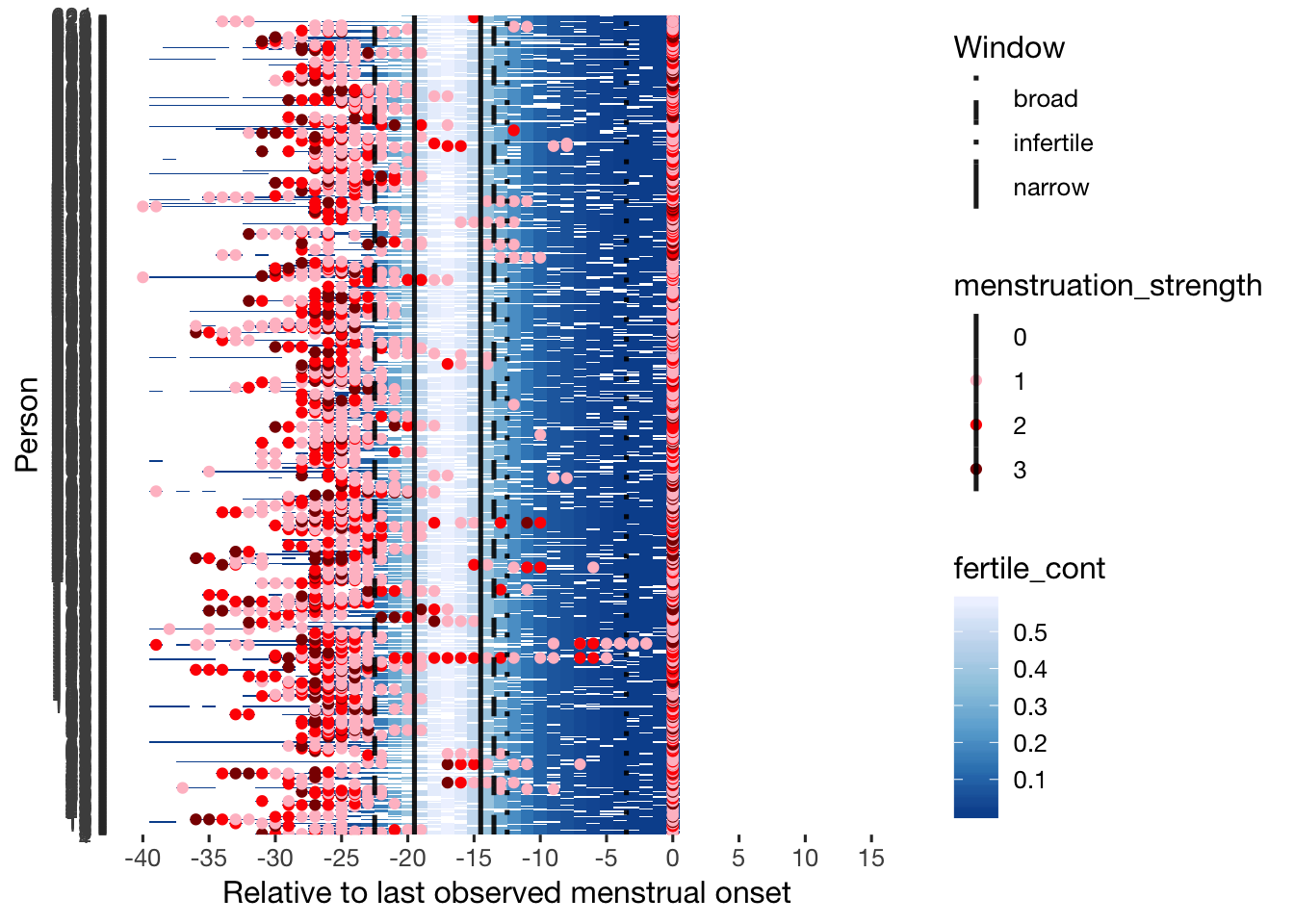# a case where the nachbe helped
# diary[session %contains% "eaca39", list(created_date,  menstruation_dates, next_menstrual_onset, last_menstrual_onset, menstruated_at_all, menstruation_2,RCD, fertile_cont,fertile_broad) ] %>% arrange(created_date) %>% View()
# a case where we observed a menstruation at the end and in the beginning, but missed the onsets
# diary[session %contains% "b270e1", list(created_date,  menstruation_dates, next_menstrual_onset, last_menstrual_onset, menstruated_at_all, menstruation_2,RCD, fertile_cont,fertile_broad) ] %>% arrange(created_date) %>% View()
# a case where we observed a menstruation at the end and in the beginning
# diary[session %contains% "0b8734", list(created_date,  menstruation_dates, next_menstrual_onset, last_menstrual_onset, menstruated_at_all, menstruation_2,RCD, fertile_cont,fertile_broad) ] %>% arrange(created_date) %>% View()

# a case where she first indicated menstrual onset on the day it occurred (likely correct), then two days later reported that it occurred yesterday (likely incorrect). problem solved by not treating extremely close together menstrual onsets as separate, instead using the earlier date (closer to onset, more likely to be correct)
# diary[session %contains% "c7cc8e", list(created_date,  menstruation_dates, next_menstrual_onset, last_menstrual_onset, menstruated_at_all, menstruation_2,RCD, fertile_cont,fertile_broad) ] %>% arrange(created_date) %>% View()

## two cases with a little misreporting of ongoing periods
# diary[session %contains% "43a25b", list(created_date,  menstruation_dates, next_menstrual_onset, last_menstrual_onset, menstruated_at_all, menstruation_2,RCD, fertile_cont,fertile_broad) ] %>% arrange(created_date) %>% head(60) %>% View()
# diary[session %contains% "8d4629", list(created_date,  menstruation_dates, next_menstrual_onset, last_menstrual_onset, menstruated_at_all, menstruation_2,RCD, fertile_cont,fertile_broad) ] %>% arrange(created_date) %>% head(60) %>% View()

qplot(data = diary[!duplicated(session) & between(median_cycle_length_diary, 20,40), ], median_cycle_length_diary, cyklus_3, colour =  factor(cyklus_4)) + geom_smooth(method = 'lm')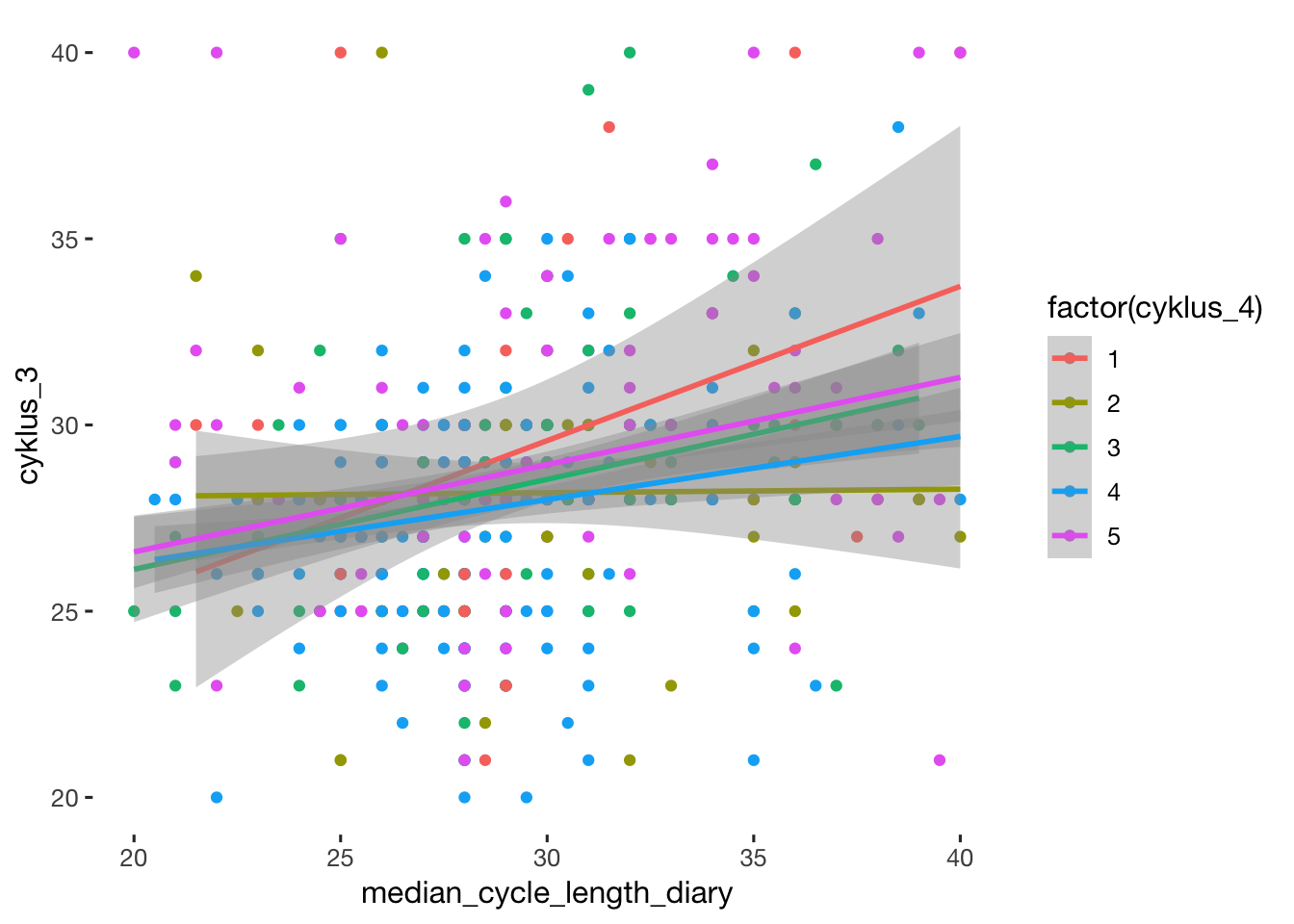cor.test(diary[!duplicated(session) & between(median_cycle_length_diary, 20,40) & cyklus_4 > 4, ]$median_cycle_length_diary, diary[!duplicated(session) & between(median_cycle_length_diary, 20,40) & cyklus_4 > 4, ]$cyklus_3)
##
##  Pearson's product-moment correlation
##
## data:  diary[!duplicated(session) & between(median_cycle_length_diary,  and diary[!duplicated(session) & between(median_cycle_length_diary,     20, 40) & cyklus_4 > 4, ]$median_cycle_length_diary and 20, 40) & cyklus_4 > 4, ]$cyklus_3
## t = 4.5, df = 250, p-value = 0.00001
## alternative hypothesis: true correlation is not equal to 0
## 95 percent confidence interval:
##  0.1537 0.3815
## sample estimates:
##    cor
## 0.2714
diary$week_number = cut(diary$day_number, c(0,7,14,21,28,35,600), include.lowest = T)

diary = diary %>%
group_by(session, cycle_nr) %>%
mutate(minimum_cycle_length_diary = if_else(!is.na(cycle_length_diary), cycle_length_diary,
max(FCD,na.rm = T)),
minimum_cycle_length_diary = if_else(minimum_cycle_length_diary == -Inf, NA_real_, minimum_cycle_length_diary)
) %>%
group_by(session) %>%
data.table()

## Applying various inclusion criteria

pipeline$completed_pre_survey = nrow(xsection) ### include_all some preparations: library(dplyr) min_max_diff = function(x) {as.numeric(max(as.Date(x),na.rm=T) - min(as.Date(x)),na.rm=T) } diary_summaries = diary %>% group_by(session) %>% summarise(took_days = min_max_diff(created), n_days = sum(!is.na(ended) & !is.na(communication_partner_1)), # look at first item too, because some had ended due to being "closed" after some time of non-response, a software thing avg_diff = mean(as.numeric(diff(created), units = "days"),na.rm = T), biggest_diff = max(as.numeric(diff(created), units = "days"),na.rm = T), had_any_period = any(menstruation_1 == 1, na.rm = T), fertile_days_known_backward = sum(!is.na(ended) & !is.na(communication_partner_1) & !is.na(prc_stirn_b)), fertile_days_known_backward_inferred = sum(!is.na(ended) & !is.na(communication_partner_1) & !is.na(prc_stirn_b_backward_inferred)), fertile_days_known_forward = sum(!is.na(ended) & !is.na(communication_partner_1) & !is.na(prc_stirn_b_forward_counted)) ) diary_summaries[, any_fertile_days_known := fertile_days_known_backward_inferred > 0] crosstabs(~ any_fertile_days_known + had_any_period, diary_summaries) ## had_any_period ## any_fertile_days_known FALSE TRUE ## FALSE 124 6 ## TRUE 174 904 crosstabs(~ had_any_period + (n_days > 20), data = diary_summaries) ## n_days > 20 ## had_any_period FALSE TRUE ## FALSE 229 69 ## TRUE 163 747 diary_summaries[any_fertile_days_known == T, had_any_period := T] xsection = merge(xsection, diary_summaries, by = "session", all.x = T) %>% data.table() crosstabs(~ age + had_any_period, data = xsection[n_days > 35, ]) ## had_any_period ## age FALSE TRUE ## 18 1 10 ## 19 0 28 ## 20 0 41 ## 21 0 32 ## 22 2 27 ## 23 2 34 ## 24 1 14 ## 25 0 17 ## 26 0 15 ## 27 0 9 ## 28 0 7 ## 29 0 7 ## 30 0 5 ## 31 0 5 ## 32 0 7 ## 33 1 2 ## 34 0 4 ## 35 0 3 ## 36 0 2 ## 37 0 3 ## 38 0 1 ## 39 0 3 ## 40 0 3 ## 41 0 3 ## 42 0 2 ## 43 0 1 ## 45 0 3 ## 46 0 2 ## 47 0 3 ## 48 0 2 ## 49 2 2 ## 50 1 3 ## 51 0 1 ## 53 1 0 ## 55 0 1 ## 58 0 1 ## 60 1 0 crosstabs(~ age + any_fertile_days_known, data = xsection[n_days > 35, ]) ## any_fertile_days_known ## age FALSE TRUE ## 18 1 10 ## 19 0 28 ## 20 0 41 ## 21 0 32 ## 22 2 27 ## 23 2 34 ## 24 1 14 ## 25 0 17 ## 26 0 15 ## 27 0 9 ## 28 0 7 ## 29 0 7 ## 30 0 5 ## 31 0 5 ## 32 0 7 ## 33 1 2 ## 34 0 4 ## 35 0 3 ## 36 0 2 ## 37 0 3 ## 38 0 1 ## 39 0 3 ## 40 0 3 ## 41 0 3 ## 42 0 2 ## 43 0 1 ## 45 0 3 ## 46 0 2 ## 47 0 3 ## 48 0 2 ## 49 2 2 ## 50 1 3 ## 51 0 1 ## 53 1 0 ## 55 0 1 ## 58 0 1 ## 60 1 0 crosstabs(~ age + (fertile_days_known_backward > 0), data = xsection[n_days > 35, ]) ## fertile_days_known_backward > 0 ## age FALSE TRUE ## 18 1 10 ## 19 1 27 ## 20 3 38 ## 21 1 31 ## 22 3 26 ## 23 4 32 ## 24 1 14 ## 25 0 17 ## 26 1 14 ## 27 0 9 ## 28 0 7 ## 29 0 7 ## 30 1 4 ## 31 0 5 ## 32 0 7 ## 33 1 2 ## 34 0 4 ## 35 0 3 ## 36 0 2 ## 37 0 3 ## 38 1 0 ## 39 0 3 ## 40 0 3 ## 41 0 3 ## 42 0 2 ## 43 1 0 ## 45 0 3 ## 46 1 1 ## 47 1 2 ## 48 0 2 ## 49 2 2 ## 50 3 1 ## 51 0 1 ## 53 1 0 ## 55 1 0 ## 58 1 0 ## 60 1 0 crosstabs(~ age + (fertile_days_known_forward > 0), data = xsection) ## fertile_days_known_forward > 0 ## age FALSE TRUE ## 18 7 29 ## 19 6 68 ## 20 8 85 ## 21 8 104 ## 22 9 96 ## 23 11 118 ## 24 7 101 ## 25 3 73 ## 26 5 80 ## 27 5 47 ## 28 7 39 ## 29 3 32 ## 30 5 24 ## 31 0 16 ## 32 0 20 ## 33 4 23 ## 34 1 11 ## 35 0 11 ## 36 0 13 ## 37 1 9 ## 38 0 9 ## 39 1 12 ## 40 1 9 ## 41 1 8 ## 42 0 3 ## 43 0 3 ## 44 0 7 ## 45 2 7 ## 46 0 3 ## 47 1 7 ## 48 1 3 ## 49 3 6 ## 50 3 8 ## 51 1 3 ## 52 2 1 ## 53 3 2 ## 54 1 0 ## 55 1 1 ## 57 0 1 ## 58 1 1 ## 60 2 0 ## 69 0 1 unique(xsection[n_days > 0, list(age, n_days, fertile_days_known_forward, fertile_days_known_backward, had_any_period,any_fertile_days_known)]) %>% arrange(age) %>% tail(40) unique(xsection[n_days >= 30, list(age, n_days, fertile_days_known_forward, fertile_days_known_backward, had_any_period,any_fertile_days_known)]) %>% arrange(age) %>% tail(40) xsection[age > 45 & !had_any_period, any_fertile_days_known := FALSE] unique(xsection[n_days >= 30 & any_fertile_days_known == T, list(age, n_days, fertile_days_known_forward, fertile_days_known_backward, had_any_period,any_fertile_days_known)]) %>% arrange(age) %>% tail(40) #### non-debatable exclusion criteria: • menopausal (indirectly defined by us still observing periods) • use hormonal contraception • pregnant excluded_old = NULL xsection[, include_all := T] # default is to include everybody xsection[ include_all == T, include_all := !is.na(n_days) & n_days > 0] # ever responded to diary pipeline$never_did_diary = n_excluded(xsection$include_all) ## 54 excluded xsection[str_sub(session, 1,10) == "cd78621db4", include_all := F] # reported hormonal treatment in preparation for IVF xsection[ include_all == T, include_all := ! pregnant %contains% "1"] # only non-pregnant (in last 3 months) pipeline$pregnant = n_excluded(xsection$include_all) ## 24 excluded xsection[ include_all == T, include_all := ! contraception %contains_word% "infertile"] # only not infertile pipeline$infertile = n_excluded(xsection$include_all) ## 5 excluded xsection[ include_all == T, include_all := ! contraception %contains_word% "sterilised"] # only non-pregnant (in last 3 months) pipeline$sterilised = n_excluded(xsection$include_all) ## 12 excluded xsection[ include_all == T, include_all := any_fertile_days_known] # only those where we could infer fertility on at least one day pipeline$fertility_not_estimable = n_excluded(xsection$include_all) ## 59 excluded # here we allow those who breastfed in last 3 months, because those we excluded those with lactational amenorrhoe already above # xsection[ include_all == T, include_all := ! breast_feeding %contains% "1"] # only non-breast_feeding # n_excluded(xsection$include_all)

xsection[, hormonal_all := include_all]
pipeline$naturally_cycling = sum(xsection[ include_all == T, ! contraception %contains% "contraceptive_pill" & !contraception %contains% "other_hormonal"], na.rm = T) xsection[ include_all == T, include_all := ! contraception %contains% "contraceptive_pill" & !contraception %contains% "other_hormonal"] # only non-hormonally contracepting, in this case we leave women who take the morning after pill with the naturally cycling women pipeline_hc$HC_users = sum(xsection[ hormonal_all == T,
contraception %contains% "contraceptive_pill"  |
contraception %contains% "other_hormonal"], na.rm = T)
xsection[ hormonal_all == T, hormonal_all :=
contraception %contains% "contraceptive_pill"  |
contraception %contains% "other_hormonal"] # only hormonally contracepting

xsection[, included_bool := include_all & !hormonal_all]
xsection[hormonal_all == F & include_all == F, included_bool := NA]
xsection[, included_all := factor(ifelse(included_bool, "cycling",  "horm_contra"))]
crosstabs(~ included_all, xsection)
## included_all
##     cycling horm_contra
##         429         625

429 non-hormonally contracepting women and 625 hormonally contracepting women are included in the sample with only mandatory exclusion criteria.

### include_lax

1. nur die obligatorischen Ausschlusskriterien (finished study, didn’t take too long, use hormonal contraception, switched to hormonal contraception, certain medication, took pill/hormonal antibiotics during the last three months, certain illness, breastfeeding, pregnant, irregular cycle) #### exclusion criteria:
• age >= 40
• use hormonal contraception
• switched to hormonal contraception
• certain medication
• tool pill/hormonal antibiotics during the last three months
• certain illness
• breastfeeding
• pregnant
• irregular cycle
• guessed the hypothesis
excluded_old = NULL
xsection[, include_lax := include_all] # default is to include everybody
n_excluded(xsection$include_lax) ## 779 excluded ##  779 xsection[ include_lax == T, include_lax := age < 40] # include only those who are younger than 40 pipeline$older_than_40 = n_excluded(xsection$include_lax) ## 54 excluded xsection[ include_lax == T, include_lax := ! is.na (ended.abschluss)] # include only those who finished the abschluss survey pipeline$finished_post_survey = n_excluded(xsection$include_lax) ## 137 excluded xsection[ include_lax == T, include_lax := ! contraception %contains% "contraceptive_pill" & ! contraception %contains% "morning_after_pill" & !contraception %contains% "other_hormonal" & !contraception %contains% "infertile"] # only non-hormonally contracepting pipeline$hc_users_broad = n_excluded(xsection$include_lax) ## 2 excluded xsection[ include_lax == T, include_lax := ! breast_feeding %contains% "1"] # only non-breast_feeding pipeline$breast_feeding = n_excluded(xsection$include_lax) ## 6 excluded xsection[ include_lax == T, include_lax := !pille_control %contains% "1"] # pill in the last 3 months pipeline$pill_last_3m = n_excluded(xsection$include_lax) ## 14 excluded xsection[ include_lax == T, include_lax := !antibiotics %contains% "1"] # no hormonal medication pipeline$hormonal_medicine = n_excluded(xsection$include_lax) ## 25 excluded table(xsection$cyklus_3) #show cycle lengths
##
##  20  21  22  23  24  25  26  27  28  29  30  31  32  33  34  35  36  37  38  39  40
##  13  22   7  23  28  87  66  57 504  84 150  29  31  13   9  36   2   2   2   1  42
xsection[, hormonal_contra := contraception %contains% "contraceptive_pill" & ! contraception %contains% "morning_after_pill" & !contraception %contains% "other_hormonal" & !contraception %contains% "infertile"]
crosstabs(~ xsection$hormonal_contra + xsection$cyklus_4)
##                         xsection$cyklus_4 ## xsection$hormonal_contra   1   2   3   4   5
##                    FALSE  62  66 119 169 189
##                    TRUE   33  46  95 194 234
##                    <NA>    0   0   0   1   0
xtabs(~ cyklus_3 + cyklus_4, data = xsection)
##         cyklus_4
## cyklus_3   1   2   3   4   5
##       20   4   0   1   4   4
##       21   1   3   4   7   7
##       22   2   1   1   2   1
##       23   1   1  10   7   4
##       24   2   0   3  14   9
##       25  16  13  17  26  15
##       26   4   4  11  33  14
##       27   4   9  12  16  16
##       28  17  28  70 141 248
##       29   1   5  15  34  29
##       30  17  26  39  42  26
##       31   3   0   4  12  10
##       32   3   7   6   5  10
##       33   0   3   3   4   3
##       34   0   1   2   3   3
##       35   4   4   9   7  12
##       36   1   0   0   0   1
##       37   0   0   1   0   1
##       38   1   0   0   1   0
##       39   0   0   1   0   0
##       40  14   7   5   6  10
xsection[ include_lax == T, include_lax := cyklus_3 > 21 & cyklus_3 < 38] #exclude those with wrong cycle lengths
pipeline$long_or_short_cycle = n_excluded(xsection$include_lax)
## 10 excluded
crosstabs(~ include_lax + hypothesis_guessed, data = xsection)
##            hypothesis_guessed
## include_lax     hormones menstruation pille zyklus zyklus, pille
##       FALSE 986        6            1     3     29             1
##       TRUE  157        0            0     3     21             0
##       <NA>    1        0            0     0      0             0
xsection[ include_lax == T, include_lax := is.na(hypothesis_guessed) | ! hypothesis_guessed %contains% "zyklus"] #exclude those who guessed hypotheses
pipeline$guessed_hypothesis = n_excluded(xsection$include_lax)
## 21 excluded
xsection[ include_lax == T, include_lax := !(took_days > 70 | avg_diff > 10)] #exclude laggards
pipeline$laggards = n_excluded(xsection$include_lax)
## 17 excluded
pipeline$criterion_lax = sum(xsection$include_lax, na.rm = T)

143 non-hormonally contracepting women and 0 hormonally contracepting women are included in the sample with lax inclusion criteria.

#### hormonally contracepting control group

excluded_old = NULL
xsection[, hormonal_lax := hormonal_all] # default is to include everybody
n_excluded(xsection$hormonal_lax) ## 583 excluded ##  583 xsection[ hormonal_lax == T, hormonal_lax := age < 40] # include only those below 40 pipeline_hc$older_than_40 = n_excluded(xsection$hormonal_lax) ## 10 excluded xsection[ hormonal_lax == T, hormonal_lax := ! is.na (ended.abschluss)] # include only those who finished the abschluss survey pipeline_hc$finished_post_survey = n_excluded(xsection$hormonal_lax) ## 168 excluded xsection[ hormonal_lax == T, hormonal_lax := ! breast_feeding %contains% "1"] # only non-breast_feeding pipeline_hc$breast_feeding = n_excluded(xsection$hormonal_lax) ## 2 excluded xsection[ hormonal_lax == T, hormonal_lax := cyklus_3 > 21 & cyklus_3 < 38] #exclude those with wrong cycle lengths pipeline_hc$long_or_short_cycle = n_excluded(xsection$hormonal_lax) ## 28 excluded xsection[ hormonal_lax == T, hormonal_lax := ! hypothesis_guessed %contains% "zyklus" & ! hypothesis_guessed %contains% "hormones"] #exclude those who guessed hypotheses pipeline_hc$guessed_hypothesis = n_excluded(xsection$hormonal_lax) ## 19 excluded xsection[ hormonal_lax == T, hormonal_lax := !(took_days > 70 | avg_diff > 10)] #exclude laggards pipeline_hc$laggards = n_excluded(xsection$hormonal_lax) ## 24 excluded xsection[, included_bool := include_lax & !hormonal_lax] xsection[hormonal_lax == F & include_lax == F, included_bool := NA] xsection[, included_lax := factor(ifelse(included_bool, "cycling", "horm_contra"))] is.na(xsection$included_lax)[is.na(xsection$included_bool)] <- TRUE crosstabs( ~factor(xsection$included_lax,exclude = NULL))
## factor(xsection$included_lax, exclude = NULL) ## cycling horm_contra <NA> ## 143 374 691 pipeline_hc$criterion_lax = sum(xsection$hormonal_lax, na.rm = T) ### include_conservative #### conservative exclusion criteria: • BMI • weight loss • cigs • intensive sports • those with irregular cycle but only if they are fairly sure about regularity excluded_old = NULL xsection[, include_conservative := include_lax] n_excluded(xsection$include_conservative)
## 1065 excluded
##  1065
xsection[include_conservative == T, include_conservative := ! cigarettes > 10]
pipeline$heavy_smokers = n_excluded(xsection$include_conservative)
## 4 excluded
xsection[include_conservative == T, include_conservative := ! intensive_sports > 7]
pipeline$intensive_sports = n_excluded(xsection$include_conservative)
## 6 excluded
xsection[include_conservative == T, include_conservative := ! breakup == 1]
pipeline$broke_up = n_excluded(xsection$include_conservative)
## 3 excluded
xsection[include_conservative == T, include_conservative := ! change_contraception_1 %contains% "1"]
pipeline$changed_contraception = n_excluded(xsection$include_conservative)
## 0 excluded
xsection[include_conservative == T, include_conservative := weight_post - weight > -8]
pipeline$lost_more_than_8kg = n_excluded(xsection$include_conservative)
## 2 excluded
xsection[, BMI := ((weight_post + weight)/2) /((height/100)^2)] #creating new variable "BMI" in dataset
qplot(xsection$BMI)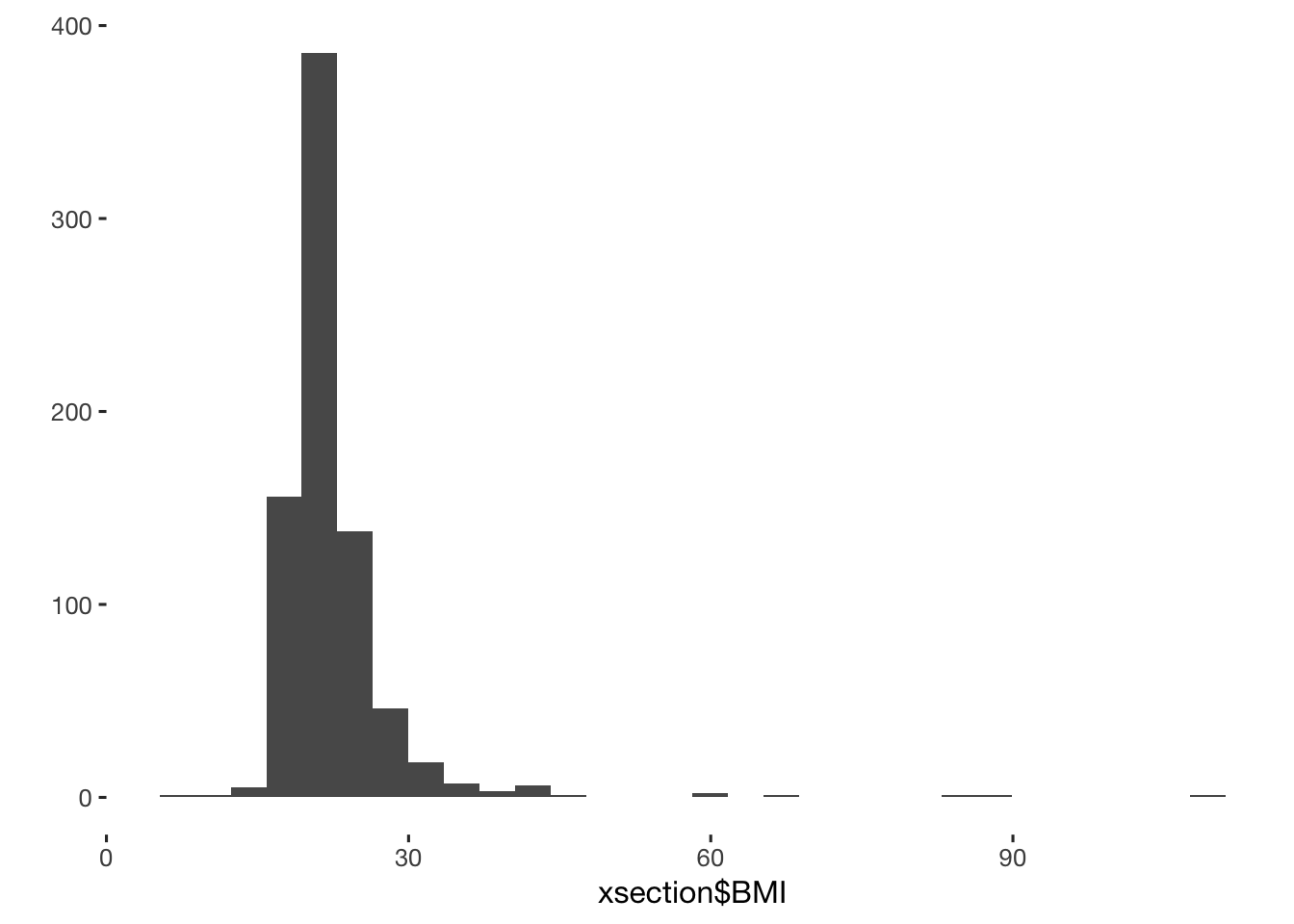xsection[include_conservative == T, include_conservative := BMI > 17] pipeline$BMI_lt_17 = n_excluded(xsection$include_conservative) ## 3 excluded xsection[include_conservative == T, include_conservative := BMI < 30] pipeline$BMI_gt_30 = n_excluded(xsection$include_conservative) ## 8 excluded table(xsection$cyklus_5)  #show if and how much cycle was off during the last three months
##
##   1   2   3
## 779 237 192
xtabs(~ cyklus_5 + cyklus_6, data = xsection)
##         cyklus_6
## cyklus_5   1   2   3   4   5
##        1  18  10  23 128 600
##        2   9  15  34  72 107
##        3  22   4  12  31 123
xsection[include_conservative == T, include_conservative := cyklus_5 < 3 | cyklus_6 == 5] #exclude those with irregular cycles, IF they have expressed some certainty in this
pipeline$confident_that_cycle_irregular = n_excluded(xsection$include_conservative)
## 5 excluded
pipeline$criterion_conservative = sum(xsection$include_conservative, na.rm = T)
excluded_old = NULL
xsection[, hormonal_conservative := hormonal_lax]
n_excluded(xsection$hormonal_conservative) ## 834 excluded ##  834 xsection[hormonal_conservative == T, hormonal_conservative := ! cigarettes > 10] pipeline_hc$heavy_smokers = n_excluded(xsection$hormonal_conservative) ## 10 excluded xsection[hormonal_conservative == T, hormonal_conservative := ! intensive_sports > 7] pipeline_hc$intensive_sports = n_excluded(xsection$hormonal_conservative) ## 18 excluded xsection[hormonal_conservative == T, hormonal_conservative := ! breakup == 1] pipeline_hc$broke_up = n_excluded(xsection$hormonal_conservative) ## 10 excluded xsection[hormonal_conservative == T, hormonal_conservative := ! change_contraception_1 %contains% "1"] pipeline_hc$changed_contraception = n_excluded(xsection$hormonal_conservative) ## 14 excluded xsection[hormonal_conservative == T, hormonal_conservative := weight_post - weight > -8] pipeline_hc$lost_more_than_8kg = n_excluded(xsection$hormonal_conservative) ## 6 excluded xsection[, BMI := ((weight_post + weight)/2) /((height/100)^2)] #creating new variable "BMI" in dataset qplot(xsection$BMI)xsection[hormonal_conservative == T, hormonal_conservative := BMI > 17]
pipeline_hc$BMI_lt_17 = n_excluded(xsection$hormonal_conservative)
## 7 excluded
xsection[hormonal_conservative == T, hormonal_conservative := BMI < 30]
pipeline_hc$BMI_gt_30 = n_excluded(xsection$hormonal_conservative)
## 10 excluded
table(xsection$cyklus_5) #show if and how much cycle was off during the last three months ## ## 1 2 3 ## 779 237 192 xtabs(~ cyklus_5 + cyklus_6, data = xsection) ## cyklus_6 ## cyklus_5 1 2 3 4 5 ## 1 18 10 23 128 600 ## 2 9 15 34 72 107 ## 3 22 4 12 31 123 xsection[hormonal_conservative == T, hormonal_conservative := cyklus_5 < 3 | cyklus_6 == 5] #exclude those with irregular cycles, IF they have expressed some certainty in this pipeline_hc$confident_that_cycle_irregular = n_excluded(xsection$hormonal_conservative) ## 6 excluded xsection[, included_bool := include_conservative & ! hormonal_conservative] xsection[ hormonal_conservative == F & include_conservative == F, included_bool := NA] xsection[, included_conservative := factor(ifelse(included_bool, "cycling", "horm_contra"))] is.na(xsection$included_conservative)[is.na(xsection$included_bool)] <- TRUE crosstabs( ~factor(xsection$included_conservative,exclude = NULL))
## factor(xsection$included_conservative, exclude = NULL) ## cycling horm_contra <NA> ## 112 294 802 pipeline_hc$criterion_conservative = sum(xsection$hormonal_conservative, na.rm = T) 112 non-hormonally contracepting women and 293 hormonally contracepting women are included in the sample with conservative exclusion criteria. ### include_strict • stressed • those with irregular cycle even if they are basically guessing about regularity excluded_old = NULL xsection[, include_strict := include_conservative] n_excluded(xsection$include_strict)
## 1096 excluded
##  1096
xsection[include_strict == T, include_strict := cyklus_5 < 3] #exclude those with irregular cycles, even the uncertain ones
pipeline$cycle_possibly_irregular = n_excluded(xsection$include_strict)
## 12 excluded
xsection[include_strict == T, include_strict := ! stress == 1]
pipeline$stressed = n_excluded(xsection$include_strict)
## 43 excluded
pipeline$criterion_strict = sum(xsection$include_strict, na.rm = T)
excluded_old = NULL
xsection[, hormonal_strict := hormonal_conservative]
n_excluded(xsection$hormonal_strict) ## 915 excluded ##  915 xsection[hormonal_strict == T, hormonal_strict := cyklus_5 < 3] #exclude those with irregular cycles, even the uncertain ones pipeline_hc$cycle_possibly_irregular = n_excluded(xsection$hormonal_strict) ## 12 excluded xsection[hormonal_strict == T, hormonal_strict := ! stress == 1] pipeline_hc$stressed = n_excluded(xsection$hormonal_strict) ## 120 excluded pipeline_hc$criterion_strict = sum(xsection$hormonal_strict, na.rm = T) xsection[, included_bool := include_strict & ! hormonal_strict] xsection[ hormonal_strict == F & include_strict == F, included_bool := NA] xsection[, included_strict := factor(ifelse(included_bool, "cycling", "horm_contra"))] is.na(xsection$included_strict)[is.na(xsection$included_bool)] <- TRUE crosstabs( ~factor(xsection$included_strict,exclude = NULL))
## factor(xsection$included_strict, exclude = NULL) ## cycling horm_contra <NA> ## 57 162 989 crosstabs(~ factor(included_strict, exclude = NULL), xsection[!is.na(included_strict), ]) ## factor(included_strict, exclude = NULL) ## cycling horm_contra ## 57 162 57 non-hormonally contracepting women and 161 hormonally contracepting women are included in the sample with overly strict exclusion criteria. ### by strictness crosstabs(~ factor(included_strict, exclude = NULL), xsection[!is.na(included_strict), ]) ## factor(included_strict, exclude = NULL) ## cycling horm_contra ## 57 162 xsection$included_levels = ifelse(!is.na(xsection$included_all),"all", NA_character_) xsection$included_levels = ifelse(!is.na(xsection$included_lax), "lax", xsection$included_levels)
xsection$included_levels = ifelse(!is.na(xsection$included_conservative), "conservative", xsection$included_levels) xsection$included_levels = ifelse(!is.na(xsection$included_strict), "strict", xsection$included_levels)
xsection$included_levels = factor(xsection$included_levels, levels = c("all", "lax", "conservative", "strict"))
xsection[, hormonal := contraception %contains% "contraceptive_pill" | contraception %contains% "other_hormonal"]
crosstabs(~ factor(xsection$included_levels, exclude = NULL)) ## factor(xsection$included_levels, exclude = NULL)
##          all          lax conservative       strict         <NA>
##          537          111          187          219          154
crosstabs(~ factor(included_levels, exclude = NULL) + hormonal, data = xsection)
##                                        hormonal
## factor(included_levels, exclude = NULL) FALSE TRUE <NA>
##                            all            286  251    0
##                            lax             31   80    0
##                            conservative    55  132    0
##                            strict          57  162    0
##                            <NA>            85   68    1

## Participant flow

See descriptives.

library(DiagrammeR)
pipeline\$hc = pipeline_hc
save(pipeline, file = "pipeline.rdata")

## Final step

Save the data.

new_names = c("session", setdiff(names(xsection), prev_names))
diary = merge(xsection %>% select(one_of(new_names)), diary, by = "session", all.x = T)
save( xsection, diary, file = "full_data.rdata")